You are reading an old version of the documentation (v2.0.2). For the latest version see https://matplotlib.org/stable/Travis-CI:# pyplot¶

## `matplotlib.pyplot`¶

Provides a MATLAB-like plotting framework.

`pylab` combines pyplot with numpy into a single namespace. This is convenient for interactive work, but for programming it is recommended that the namespaces be kept separate, e.g.:

```import numpy as np
import matplotlib.pyplot as plt

x = np.arange(0, 5, 0.1);
y = np.sin(x)
plt.plot(x, y)
```
`matplotlib.pyplot.``acorr`(x, hold=None, data=None, **kwargs)

Plot the autocorrelation of `x`.

Parameters: Returns: x : sequence of scalar hold : boolean, optional, deprecated, default: True detrend : callable, optional, default: `mlab.detrend_none` x is detrended by the `detrend` callable. Default is no normalization. normed : boolean, optional, default: True if True, input vectors are normalised to unit length. usevlines : boolean, optional, default: True if True, Axes.vlines is used to plot the vertical lines from the origin to the acorr. Otherwise, Axes.plot is used. maxlags : integer, optional, default: 10 number of lags to show. If None, will return all 2 * len(x) - 1 lags. (lags, c, line, b) : where: `lags` are a length 2`maxlags+1 lag vector. `c` is the 2`maxlags+1 auto correlation vectorI `line` is a `Line2D` instance returned by `plot`. `b` is the x-axis. linestyle : `Line2D` prop, optional, default: None Only used if usevlines is False. marker : string, optional, default: ‘o’

Notes

The cross correlation is performed with `numpy.correlate()` with `mode` = 2.

Examples

`xcorr` is top graph, and `acorr` is bottom graph.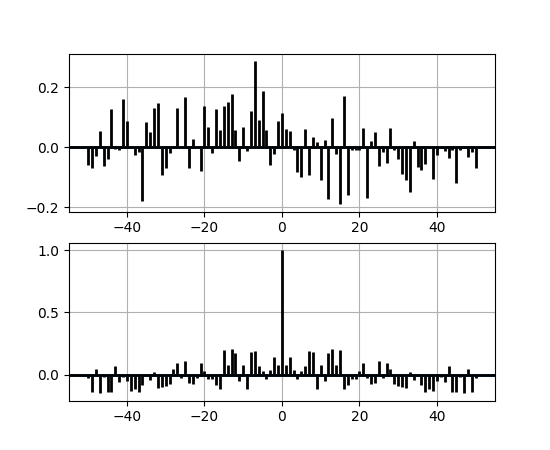Note

In addition to the above described arguments, this function can take a data keyword argument. If such a data argument is given, the following arguments are replaced by data[<arg>]:

• All arguments with the following names: ‘x’.
`matplotlib.pyplot.``angle_spectrum`(x, Fs=None, Fc=None, window=None, pad_to=None, sides=None, hold=None, data=None, **kwargs)

Plot the angle spectrum.

Call signature:

```angle_spectrum(x, Fs=2, Fc=0,  window=mlab.window_hanning,
```

Compute the angle spectrum (wrapped phase spectrum) of x. Data is padded to a length of pad_to and the windowing function window is applied to the signal.

Parameters:

x : 1-D array or sequence

Array or sequence containing the data

Fs : scalar

The sampling frequency (samples per time unit). It is used to calculate the Fourier frequencies, freqs, in cycles per time unit. The default value is 2.

window : callable or ndarray

A function or a vector of length NFFT. To create window vectors see `window_hanning()`, `window_none()`, `numpy.blackman()`, `numpy.hamming()`, `numpy.bartlett()`, `scipy.signal()`, `scipy.signal.get_window()`, etc. The default is `window_hanning()`. If a function is passed as the argument, it must take a data segment as an argument and return the windowed version of the segment.

sides : [ ‘default’ | ‘onesided’ | ‘twosided’ ]

Specifies which sides of the spectrum to return. Default gives the default behavior, which returns one-sided for real data and both for complex data. ‘onesided’ forces the return of a one-sided spectrum, while ‘twosided’ forces two-sided.

The number of points to which the data segment is padded when performing the FFT. While not increasing the actual resolution of the spectrum (the minimum distance between resolvable peaks), this can give more points in the plot, allowing for more detail. This corresponds to the n parameter in the call to fft(). The default is None, which sets pad_to equal to the length of the input signal (i.e. no padding).

Fc : integer

The center frequency of x (defaults to 0), which offsets the x extents of the plot to reflect the frequency range used when a signal is acquired and then filtered and downsampled to baseband.

**kwargs :

Keyword arguments control the `Line2D` properties:

Property Description
`agg_filter` unknown
`alpha` float (0.0 transparent through 1.0 opaque)
`animated` [True | False]
`antialiased` or aa [True | False]
`axes` an `Axes` instance
`clip_box` a `matplotlib.transforms.Bbox` instance
`clip_on` [True | False]
`clip_path` [ (`Path`, `Transform`) | `Patch` | None ]
`color` or c any matplotlib color
`contains` a callable function
`dash_capstyle` [‘butt’ | ‘round’ | ‘projecting’]
`dash_joinstyle` [‘miter’ | ‘round’ | ‘bevel’]
`dashes` sequence of on/off ink in points
`drawstyle` [‘default’ | ‘steps’ | ‘steps-pre’ | ‘steps-mid’ | ‘steps-post’]
`figure` a `matplotlib.figure.Figure` instance
`fillstyle` [‘full’ | ‘left’ | ‘right’ | ‘bottom’ | ‘top’ | ‘none’]
`gid` an id string
`label` string or anything printable with ‘%s’ conversion.
`linestyle` or ls [‘solid’ | ‘dashed’, ‘dashdot’, ‘dotted’ | (offset, on-off-dash-seq) | `'-'` | `'--'` | `'-.'` | `':'` | `'None'` | `' '` | `''`]
`linewidth` or lw float value in points
`marker` `A valid marker style`
`markeredgecolor` or mec any matplotlib color
`markeredgewidth` or mew float value in points
`markerfacecolor` or mfc any matplotlib color
`markerfacecoloralt` or mfcalt any matplotlib color
`markersize` or ms float
`markevery` [None | int | length-2 tuple of int | slice | list/array of int | float | length-2 tuple of float]
`path_effects` unknown
`picker` float distance in points or callable pick function `fn(artist, event)`
`pickradius` float distance in points
`rasterized` [True | False | None]
`sketch_params` unknown
`snap` unknown
`solid_capstyle` [‘butt’ | ‘round’ | ‘projecting’]
`solid_joinstyle` [‘miter’ | ‘round’ | ‘bevel’]
`transform` a `matplotlib.transforms.Transform` instance
`url` a url string
`visible` [True | False]
`xdata` 1D array
`ydata` 1D array
`zorder` any number
Returns:

spectrum : 1-D array

The values for the angle spectrum in radians (real valued)

freqs : 1-D array

The frequencies corresponding to the elements in spectrum

line : a `Line2D` instance

The line created by this function

`magnitude_spectrum()`
`angle_spectrum()` plots the magnitudes of the corresponding frequencies.
`phase_spectrum()`
`phase_spectrum()` plots the unwrapped version of this function.
`specgram()`
`specgram()` can plot the angle spectrum of segments within the signal in a colormap.
In addition to the above described arguments, this function can take a data keyword argument. If such a data argument is given, the following arguments are replaced by data[<arg>]: * All arguments with the following names: ‘x’.

Examples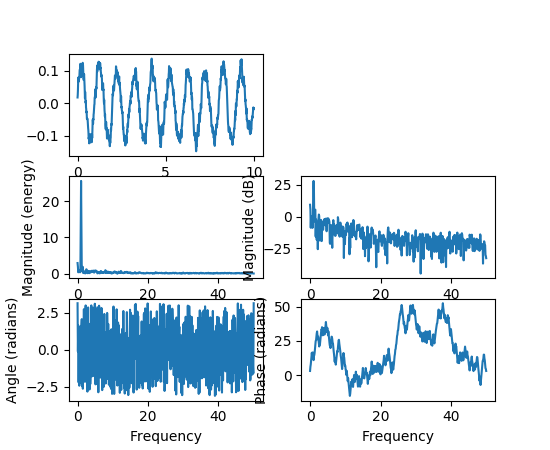`matplotlib.pyplot.``annotate`(*args, **kwargs)

Annotate the point `xy` with text `s`.

Additional kwargs are passed to `Text`.

Parameters:

s : str

The text of the annotation

xy : iterable

Length 2 sequence specifying the (x,y) point to annotate

xytext : iterable, optional

Length 2 sequence specifying the (x,y) to place the text at. If None, defaults to `xy`.

xycoords : str, Artist, Transform, callable or tuple, optional

The coordinate system that `xy` is given in.

For a `str` the allowed values are:

Property Description
‘figure points’ points from the lower left of the figure
‘figure pixels’ pixels from the lower left of the figure
‘figure fraction’ fraction of figure from lower left
‘axes points’ points from lower left corner of axes
‘axes pixels’ pixels from lower left corner of axes
‘axes fraction’ fraction of axes from lower left
‘data’ use the coordinate system of the object being annotated (default)
‘polar’ (theta,r) if not native ‘data’ coordinates

If a `Artist` object is passed in the units are fraction if it’s bounding box.

If a `Transform` object is passed in use that to transform `xy` to screen coordinates

If a callable it must take a `RendererBase` object as input and return a `Transform` or `Bbox` object

If a `tuple` must be length 2 tuple of str, `Artist`, `Transform` or callable objects. The first transform is used for the x coordinate and the second for y.

See Advanced Annotation for more details.

Defaults to `'data'`

textcoords : str, `Artist`, `Transform`, callable or tuple, optional

The coordinate system that `xytext` is given, which may be different than the coordinate system used for `xy`.

All `xycoords` values are valid as well as the following strings:

Property Description
‘offset points’ offset (in points) from the xy value
‘offset pixels’ offset (in pixels) from the xy value

defaults to the input of `xycoords`

arrowprops : dict, optional

If not None, properties used to draw a `FancyArrowPatch` arrow between `xy` and `xytext`.

If `arrowprops` does not contain the key `'arrowstyle'` the allowed keys are:

Key Description
width the width of the arrow in points
headwidth the width of the base of the arrow head in points
headlength the length of the arrow head in points
shrink fraction of total length to ‘shrink’ from both ends
? any key to `matplotlib.patches.FancyArrowPatch`

If the `arrowprops` contains the key `'arrowstyle'` the above keys are forbidden. The allowed values of `'arrowstyle'` are:

Name Attrs
`'-'` None
`'->'` head_length=0.4,head_width=0.2
`'-['` widthB=1.0,lengthB=0.2,angleB=None
`'|-|'` widthA=1.0,widthB=1.0
`'-|>'` head_length=0.4,head_width=0.2
`'<-'` head_length=0.4,head_width=0.2
`'<->'` head_length=0.4,head_width=0.2
`'<|-'` head_length=0.4,head_width=0.2
`'<|-|>'` head_length=0.4,head_width=0.2
`'fancy'` head_length=0.4,head_width=0.4,tail_width=0.4
`'simple'` head_length=0.5,head_width=0.5,tail_width=0.2
`'wedge'` tail_width=0.3,shrink_factor=0.5

Valid keys for `FancyArrowPatch` are:

Key Description
arrowstyle the arrow style
connectionstyle the connection style
relpos default is (0.5, 0.5)
patchA default is bounding box of the text
patchB default is None
shrinkA default is 2 points
shrinkB default is 2 points
mutation_scale default is text size (in points)
mutation_aspect default is 1.
? any key for `matplotlib.patches.PathPatch`

Defaults to None

annotation_clip : bool, optional

Controls the visibility of the annotation when it goes outside the axes area.

If `True`, the annotation will only be drawn when the `xy` is inside the axes. If `False`, the annotation will always be drawn regardless of its position.

The default is `None`, which behave as `True` only if xycoords is “data”.

Returns:

Annotation

`matplotlib.pyplot.``arrow`(x, y, dx, dy, hold=None, **kwargs)

Add an arrow to the axes.

Draws arrow on specified axis from (`x`, `y`) to (`x` + `dx`, `y` + `dy`). Uses FancyArrow patch to construct the arrow.

Parameters:

x : float

X-coordinate of the arrow base

y : float

Y-coordinate of the arrow base

dx : float

Length of arrow along x-coordinate

dy : float

Length of arrow along y-coordinate

Returns:

a : FancyArrow

patches.FancyArrow object

Other Parameters:

Optional kwargs (inherited from FancyArrow patch) control the arrow

construction and properties:

Constructor arguments

width: float (default: 0.001)

width of full arrow tail

length_includes_head: [True | False] (default: False)

True if head is to be counted in calculating the length.

head_width: float or None (default: 3*width)

total width of the full arrow head

head_length: float or None (default: 1.5 * head_width)

length of arrow head

shape: [‘full’, ‘left’, ‘right’] (default: ‘full’)

draw the left-half, right-half, or full arrow

overhang: float (default: 0)

fraction that the arrow is swept back (0 overhang means triangular shape). Can be negative or greater than one.

head_starts_at_zero: [True | False] (default: False)

if True, the head starts being drawn at coordinate 0 instead of ending at coordinate 0.

Other valid kwargs (inherited from :class:`Patch`) are:

Property Description
`agg_filter` unknown
`alpha` float or None
`animated` [True | False]
`antialiased` or aa [True | False] or None for default
`axes` an `Axes` instance
`capstyle` [‘butt’ | ‘round’ | ‘projecting’]
`clip_box` a `matplotlib.transforms.Bbox` instance
`clip_on` [True | False]
`clip_path` [ (`Path`, `Transform`) | `Patch` | None ]
`color` matplotlib color spec
`contains` a callable function
`edgecolor` or ec mpl color spec, None, ‘none’, or ‘auto’
`facecolor` or fc mpl color spec, or None for default, or ‘none’ for no color
`figure` a `matplotlib.figure.Figure` instance
`fill` [True | False]
`gid` an id string
`hatch` [‘/’ | ‘\’ | ‘|’ | ‘-‘ | ‘+’ | ‘x’ | ‘o’ | ‘O’ | ‘.’ | ‘*’]
`joinstyle` [‘miter’ | ‘round’ | ‘bevel’]
`label` string or anything printable with ‘%s’ conversion.
`linestyle` or ls [‘solid’ | ‘dashed’, ‘dashdot’, ‘dotted’ | (offset, on-off-dash-seq) | `'-'` | `'--'` | `'-.'` | `':'` | `'None'` | `' '` | `''`]
`linewidth` or lw float or None for default
`path_effects` unknown
`picker` [None|float|boolean|callable]
`rasterized` [True | False | None]
`sketch_params` unknown
`snap` unknown
`transform` `Transform` instance
`url` a url string
`visible` [True | False]
`zorder` any number

Notes

The resulting arrow is affected by the axes aspect ratio and limits. This may produce an arrow whose head is not square with its stem. To create an arrow whose head is square with its stem, use `annotate()` for example:

```ax.annotate("", xy=(0.5, 0.5), xytext=(0, 0),
arrowprops=dict(arrowstyle="->"))
```

Examples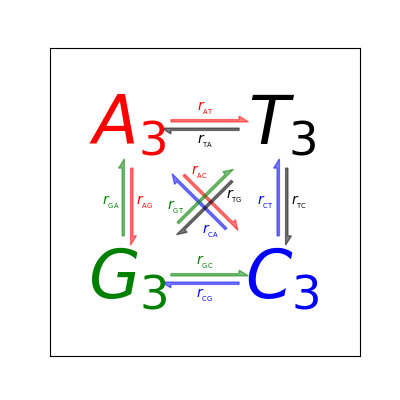`matplotlib.pyplot.``autoscale`(enable=True, axis='both', tight=None)

Autoscale the axis view to the data (toggle).

Convenience method for simple axis view autoscaling. It turns autoscaling on or off, and then, if autoscaling for either axis is on, it performs the autoscaling on the specified axis or axes.

enable: [True | False | None]
True (default) turns autoscaling on, False turns it off. None leaves the autoscaling state unchanged.
axis: [‘x’ | ‘y’ | ‘both’]
which axis to operate on; default is ‘both’
tight: [True | False | None]
If True, set view limits to data limits; if False, let the locator and margins expand the view limits; if None, use tight scaling if the only artist is an image, otherwise treat tight as False. The tight setting is retained for future autoscaling until it is explicitly changed.

Returns None.

`matplotlib.pyplot.``autumn`()

set the default colormap to autumn and apply to current image if any. See help(colormaps) for more information

`matplotlib.pyplot.``axes`(*args, **kwargs)

Add an axes to the figure.

The axes is added at position rect specified by:

• `axes()` by itself creates a default full `subplot(111)` window axis.
• `axes(rect, facecolor='w')` where rect = [left, bottom, width, height] in normalized (0, 1) units. facecolor is the background color for the axis, default white.
• `axes(h)` where h is an axes instance makes h the current axis. An `Axes` instance is returned.
kwarg Accepts Description
facecolor color the axes background color
frameon [True|False] display the frame?
sharex otherax current axes shares xaxis attribute with otherax
sharey otherax current axes shares yaxis attribute with otherax
polar [True|False] use a polar axes?
aspect [str | num] [‘equal’, ‘auto’] or a number. If a number the ratio of x-unit/y-unit in screen-space. Also see `set_aspect()`.

Examples:

• `examples/pylab_examples/axes_demo.py` places custom axes.
• `examples/pylab_examples/shared_axis_demo.py` uses sharex and sharey.
`matplotlib.pyplot.``axhline`(y=0, xmin=0, xmax=1, hold=None, **kwargs)

Add a horizontal line across the axis.

Parameters: y : scalar, optional, default: 0 y position in data coordinates of the horizontal line. xmin : scalar, optional, default: 0 Should be between 0 and 1, 0 being the far left of the plot, 1 the far right of the plot. xmax : scalar, optional, default: 1 Should be between 0 and 1, 0 being the far left of the plot, 1 the far right of the plot. `Line2D`

`axhspan`
for example plot and source code

Notes

kwargs are passed to `Line2D` and can be used to control the line properties.

Examples

• draw a thick red hline at ‘y’ = 0 that spans the xrange:

```>>> axhline(linewidth=4, color='r')
```
• draw a default hline at ‘y’ = 1 that spans the xrange:

```>>> axhline(y=1)
```
• draw a default hline at ‘y’ = .5 that spans the middle half of the xrange:

```>>> axhline(y=.5, xmin=0.25, xmax=0.75)
```

Valid kwargs are `Line2D` properties, with the exception of ‘transform’:

Property Description
`agg_filter` unknown
`alpha` float (0.0 transparent through 1.0 opaque)
`animated` [True | False]
`antialiased` or aa [True | False]
`axes` an `Axes` instance
`clip_box` a `matplotlib.transforms.Bbox` instance
`clip_on` [True | False]
`clip_path` [ (`Path`, `Transform`) | `Patch` | None ]
`color` or c any matplotlib color
`contains` a callable function
`dash_capstyle` [‘butt’ | ‘round’ | ‘projecting’]
`dash_joinstyle` [‘miter’ | ‘round’ | ‘bevel’]
`dashes` sequence of on/off ink in points
`drawstyle` [‘default’ | ‘steps’ | ‘steps-pre’ | ‘steps-mid’ | ‘steps-post’]
`figure` a `matplotlib.figure.Figure` instance
`fillstyle` [‘full’ | ‘left’ | ‘right’ | ‘bottom’ | ‘top’ | ‘none’]
`gid` an id string
`label` string or anything printable with ‘%s’ conversion.
`linestyle` or ls [‘solid’ | ‘dashed’, ‘dashdot’, ‘dotted’ | (offset, on-off-dash-seq) | `'-'` | `'--'` | `'-.'` | `':'` | `'None'` | `' '` | `''`]
`linewidth` or lw float value in points
`marker` `A valid marker style`
`markeredgecolor` or mec any matplotlib color
`markeredgewidth` or mew float value in points
`markerfacecolor` or mfc any matplotlib color
`markerfacecoloralt` or mfcalt any matplotlib color
`markersize` or ms float
`markevery` [None | int | length-2 tuple of int | slice | list/array of int | float | length-2 tuple of float]
`path_effects` unknown
`picker` float distance in points or callable pick function `fn(artist, event)`
`pickradius` float distance in points
`rasterized` [True | False | None]
`sketch_params` unknown
`snap` unknown
`solid_capstyle` [‘butt’ | ‘round’ | ‘projecting’]
`solid_joinstyle` [‘miter’ | ‘round’ | ‘bevel’]
`transform` a `matplotlib.transforms.Transform` instance
`url` a url string
`visible` [True | False]
`xdata` 1D array
`ydata` 1D array
`zorder` any number
`matplotlib.pyplot.``axhspan`(ymin, ymax, xmin=0, xmax=1, hold=None, **kwargs)

Add a horizontal span (rectangle) across the axis.

Draw a horizontal span (rectangle) from ymin to ymax. With the default values of xmin = 0 and xmax = 1, this always spans the xrange, regardless of the xlim settings, even if you change them, e.g., with the `set_xlim()` command. That is, the horizontal extent is in axes coords: 0=left, 0.5=middle, 1.0=right but the y location is in data coordinates.

Parameters:

ymin : float

Lower limit of the horizontal span in data units.

ymax : float

Upper limit of the horizontal span in data units.

xmin : float, optional, default: 0

Lower limit of the vertical span in axes (relative 0-1) units.

xmax : float, optional, default: 1

Upper limit of the vertical span in axes (relative 0-1) units.

Returns:

Polygon : `Polygon`

Other Parameters:

kwargs : `Polygon` properties.

Property Description
`agg_filter` unknown
`alpha` float or None
`animated` [True | False]
`antialiased` or aa [True | False] or None for default
`axes` an `Axes` instance
`capstyle` [‘butt’ | ‘round’ | ‘projecting’]
`clip_box` a `matplotlib.transforms.Bbox` instance
`clip_on` [True | False]
`clip_path` [ (`Path`, `Transform`) | `Patch` | None ]
`color` matplotlib color spec
`contains` a callable function
`edgecolor` or ec mpl color spec, None, ‘none’, or ‘auto’
`facecolor` or fc mpl color spec, or None for default, or ‘none’ for no color
`figure` a `matplotlib.figure.Figure` instance
`fill` [True | False]
`gid` an id string
`hatch` [‘/’ | ‘\’ | ‘|’ | ‘-‘ | ‘+’ | ‘x’ | ‘o’ | ‘O’ | ‘.’ | ‘*’]
`joinstyle` [‘miter’ | ‘round’ | ‘bevel’]
`label` string or anything printable with ‘%s’ conversion.
`linestyle` or ls [‘solid’ | ‘dashed’, ‘dashdot’, ‘dotted’ | (offset, on-off-dash-seq) | `'-'` | `'--'` | `'-.'` | `':'` | `'None'` | `' '` | `''`]
`linewidth` or lw float or None for default
`path_effects` unknown
`picker` [None|float|boolean|callable]
`rasterized` [True | False | None]
`sketch_params` unknown
`snap` unknown
`transform` `Transform` instance
`url` a url string
`visible` [True | False]
`zorder` any number

`axvspan`
Add a vertical span (rectangle) across the axes.

Examples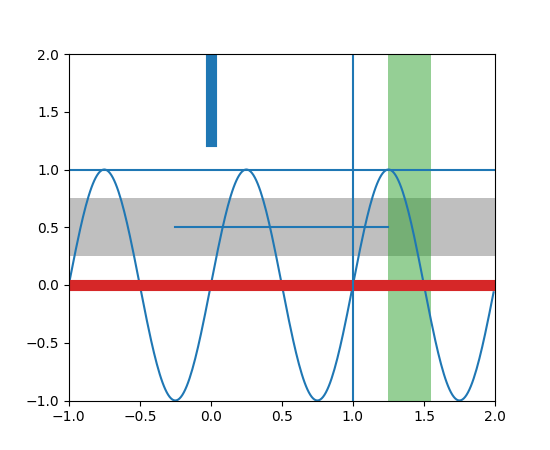`matplotlib.pyplot.``axis`(*v, **kwargs)

Convenience method to get or set axis properties.

Calling with no arguments:

```>>> axis()
```

returns the current axes limits `[xmin, xmax, ymin, ymax]`.:

```>>> axis(v)
```

sets the min and max of the x and y axes, with `v = [xmin, xmax, ymin, ymax]`.:

```>>> axis('off')
```

turns off the axis lines and labels.:

```>>> axis('equal')
```

changes limits of x or y axis so that equal increments of x and y have the same length; a circle is circular.:

```>>> axis('scaled')
```

achieves the same result by changing the dimensions of the plot box instead of the axis data limits.:

```>>> axis('tight')
```

changes x and y axis limits such that all data is shown. If all data is already shown, it will move it to the center of the figure without modifying (xmax - xmin) or (ymax - ymin). Note this is slightly different than in MATLAB.:

```>>> axis('image')
```

is ‘scaled’ with the axis limits equal to the data limits.:

```>>> axis('auto')
```

and:

```>>> axis('normal')
```

are deprecated. They restore default behavior; axis limits are automatically scaled to make the data fit comfortably within the plot box.

if `len(*v)==0`, you can pass in xmin, xmax, ymin, ymax as kwargs selectively to alter just those limits without changing the others.

```>>> axis('square')
```

changes the limit ranges (xmax-xmin) and (ymax-ymin) of the x and y axes to be the same, and have the same scaling, resulting in a square plot.

The xmin, xmax, ymin, ymax tuple is returned

`xlim()`, `ylim()`
For setting the x- and y-limits individually.
`matplotlib.pyplot.``axvline`(x=0, ymin=0, ymax=1, hold=None, **kwargs)

Add a vertical line across the axes.

Parameters: x : scalar, optional, default: 0 x position in data coordinates of the vertical line. ymin : scalar, optional, default: 0 Should be between 0 and 1, 0 being the bottom of the plot, 1 the top of the plot. ymax : scalar, optional, default: 1 Should be between 0 and 1, 0 being the bottom of the plot, 1 the top of the plot. `Line2D`

`axhspan`
for example plot and source code

Examples

• draw a thick red vline at x = 0 that spans the yrange:

```>>> axvline(linewidth=4, color='r')
```
• draw a default vline at x = 1 that spans the yrange:

```>>> axvline(x=1)
```
• draw a default vline at x = .5 that spans the middle half of the yrange:

```>>> axvline(x=.5, ymin=0.25, ymax=0.75)
```

Valid kwargs are `Line2D` properties, with the exception of ‘transform’:

Property Description
`agg_filter` unknown
`alpha` float (0.0 transparent through 1.0 opaque)
`animated` [True | False]
`antialiased` or aa [True | False]
`axes` an `Axes` instance
`clip_box` a `matplotlib.transforms.Bbox` instance
`clip_on` [True | False]
`clip_path` [ (`Path`, `Transform`) | `Patch` | None ]
`color` or c any matplotlib color
`contains` a callable function
`dash_capstyle` [‘butt’ | ‘round’ | ‘projecting’]
`dash_joinstyle` [‘miter’ | ‘round’ | ‘bevel’]
`dashes` sequence of on/off ink in points
`drawstyle` [‘default’ | ‘steps’ | ‘steps-pre’ | ‘steps-mid’ | ‘steps-post’]
`figure` a `matplotlib.figure.Figure` instance
`fillstyle` [‘full’ | ‘left’ | ‘right’ | ‘bottom’ | ‘top’ | ‘none’]
`gid` an id string
`label` string or anything printable with ‘%s’ conversion.
`linestyle` or ls [‘solid’ | ‘dashed’, ‘dashdot’, ‘dotted’ | (offset, on-off-dash-seq) | `'-'` | `'--'` | `'-.'` | `':'` | `'None'` | `' '` | `''`]
`linewidth` or lw float value in points
`marker` `A valid marker style`
`markeredgecolor` or mec any matplotlib color
`markeredgewidth` or mew float value in points
`markerfacecolor` or mfc any matplotlib color
`markerfacecoloralt` or mfcalt any matplotlib color
`markersize` or ms float
`markevery` [None | int | length-2 tuple of int | slice | list/array of int | float | length-2 tuple of float]
`path_effects` unknown
`picker` float distance in points or callable pick function `fn(artist, event)`
`pickradius` float distance in points
`rasterized` [True | False | None]
`sketch_params` unknown
`snap` unknown
`solid_capstyle` [‘butt’ | ‘round’ | ‘projecting’]
`solid_joinstyle` [‘miter’ | ‘round’ | ‘bevel’]
`transform` a `matplotlib.transforms.Transform` instance
`url` a url string
`visible` [True | False]
`xdata` 1D array
`ydata` 1D array
`zorder` any number
`matplotlib.pyplot.``axvspan`(xmin, xmax, ymin=0, ymax=1, hold=None, **kwargs)

Add a vertical span (rectangle) across the axes.

Draw a vertical span (rectangle) from `xmin` to `xmax`. With the default values of `ymin` = 0 and `ymax` = 1. This always spans the yrange, regardless of the ylim settings, even if you change them, e.g., with the `set_ylim()` command. That is, the vertical extent is in axes coords: 0=bottom, 0.5=middle, 1.0=top but the y location is in data coordinates.

Parameters: Returns: xmin : scalar Number indicating the first X-axis coordinate of the vertical span rectangle in data units. xmax : scalar Number indicating the second X-axis coordinate of the vertical span rectangle in data units. ymin : scalar, optional Number indicating the first Y-axis coordinate of the vertical span rectangle in relative Y-axis units (0-1). Default to 0. ymax : scalar, optional Number indicating the second Y-axis coordinate of the vertical span rectangle in relative Y-axis units (0-1). Default to 1. rectangle : matplotlib.patches.Polygon Vertical span (rectangle) from (xmin, ymin) to (xmax, ymax). **kwargs Optional parameters are properties of the class matplotlib.patches.Polygon.

Examples

Draw a vertical, green, translucent rectangle from x = 1.25 to x = 1.55 that spans the yrange of the axes.

```>>> axvspan(1.25, 1.55, facecolor='g', alpha=0.5)
```
`matplotlib.pyplot.``bar`(left, height, width=0.8, bottom=None, hold=None, data=None, **kwargs)

Make a bar plot.

Make a bar plot with rectangles bounded by:

`left`, `left` + `width`, `bottom`, `bottom` + `height`
(left, right, bottom and top edges)
Parameters: left : sequence of scalars the x coordinates of the left sides of the bars height : sequence of scalars the heights of the bars width : scalar or array-like, optional the width(s) of the bars default: 0.8 bottom : scalar or array-like, optional the y coordinate(s) of the bars default: None color : scalar or array-like, optional the colors of the bar faces edgecolor : scalar or array-like, optional the colors of the bar edges linewidth : scalar or array-like, optional width of bar edge(s). If None, use default linewidth; If 0, don’t draw edges. default: None tick_label : string or array-like, optional the tick labels of the bars default: None xerr : scalar or array-like, optional if not None, will be used to generate errorbar(s) on the bar chart default: None yerr : scalar or array-like, optional if not None, will be used to generate errorbar(s) on the bar chart default: None ecolor : scalar or array-like, optional specifies the color of errorbar(s) default: None capsize : scalar, optional determines the length in points of the error bar caps default: None, which will take the value from the `errorbar.capsize` `rcParam`. error_kw : dict, optional dictionary of kwargs to be passed to errorbar method. ecolor and capsize may be specified here rather than as independent kwargs. align : {‘center’, ‘edge’}, optional If ‘edge’, aligns bars by their left edges (for vertical bars) and by their bottom edges (for horizontal bars). If ‘center’, interpret the `left` argument as the coordinates of the centers of the bars. To align on the align bars on the right edge pass a negative `width`. orientation : {‘vertical’, ‘horizontal’}, optional The orientation of the bars. log : boolean, optional If true, sets the axis to be log scale. default: False bars : matplotlib.container.BarContainer Container with all of the bars + errorbars

`barh`
Plot a horizontal bar plot.

Notes

The optional arguments `color`, `edgecolor`, `linewidth`, `xerr`, and `yerr` can be either scalars or sequences of length equal to the number of bars. This enables you to use bar as the basis for stacked bar charts, or candlestick plots. Detail: `xerr` and `yerr` are passed directly to `errorbar()`, so they can also have shape 2xN for independent specification of lower and upper errors.

Other optional kwargs:

Property Description
`agg_filter` unknown
`alpha` float or None
`animated` [True | False]
`antialiased` or aa [True | False] or None for default
`axes` an `Axes` instance
`capstyle` [‘butt’ | ‘round’ | ‘projecting’]
`clip_box` a `matplotlib.transforms.Bbox` instance
`clip_on` [True | False]
`clip_path` [ (`Path`, `Transform`) | `Patch` | None ]
`color` matplotlib color spec
`contains` a callable function
`edgecolor` or ec mpl color spec, None, ‘none’, or ‘auto’
`facecolor` or fc mpl color spec, or None for default, or ‘none’ for no color
`figure` a `matplotlib.figure.Figure` instance
`fill` [True | False]
`gid` an id string
`hatch` [‘/’ | ‘\’ | ‘|’ | ‘-‘ | ‘+’ | ‘x’ | ‘o’ | ‘O’ | ‘.’ | ‘*’]
`joinstyle` [‘miter’ | ‘round’ | ‘bevel’]
`label` string or anything printable with ‘%s’ conversion.
`linestyle` or ls [‘solid’ | ‘dashed’, ‘dashdot’, ‘dotted’ | (offset, on-off-dash-seq) | `'-'` | `'--'` | `'-.'` | `':'` | `'None'` | `' '` | `''`]
`linewidth` or lw float or None for default
`path_effects` unknown
`picker` [None|float|boolean|callable]
`rasterized` [True | False | None]
`sketch_params` unknown
`snap` unknown
`transform` `Transform` instance
`url` a url string
`visible` [True | False]
`zorder` any number

Examples

Example: A stacked bar chart.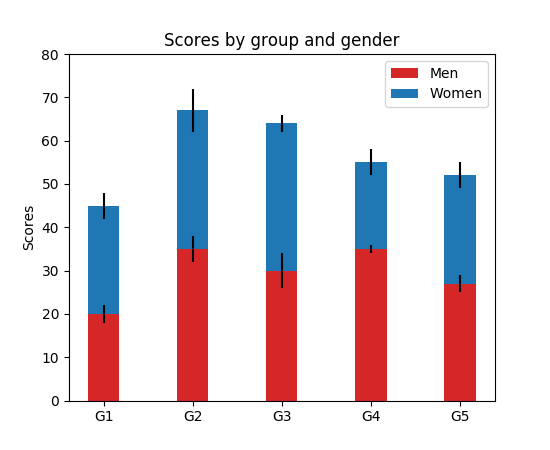Note

In addition to the above described arguments, this function can take a data keyword argument. If such a data argument is given, the following arguments are replaced by data[<arg>]:

• All arguments with the following names: ‘bottom’, ‘color’, ‘ecolor’, ‘edgecolor’, ‘height’, ‘left’, ‘linewidth’, ‘tick_label’, ‘width’, ‘xerr’, ‘yerr’.
`matplotlib.pyplot.``barbs`(*args, **kw)

Plot a 2-D field of barbs.

Call signatures:

```barb(U, V, **kw)
barb(U, V, C, **kw)
barb(X, Y, U, V, **kw)
barb(X, Y, U, V, C, **kw)
```

Arguments:

X, Y:
The x and y coordinates of the barb locations (default is head of barb; see pivot kwarg)
U, V:
Give the x and y components of the barb shaft
C:
An optional array used to map colors to the barbs

All arguments may be 1-D or 2-D arrays or sequences. If X and Y are absent, they will be generated as a uniform grid. If U and V are 2-D arrays but X and Y are 1-D, and if `len(X)` and `len(Y)` match the column and row dimensions of U, then X and Y will be expanded with `numpy.meshgrid()`.

U, V, C may be masked arrays, but masked X, Y are not supported at present.

Keyword arguments:

length:
Length of the barb in points; the other parts of the barb are scaled against this. Default is 9
pivot: [ ‘tip’ | ‘middle’ ]
The part of the arrow that is at the grid point; the arrow rotates about this point, hence the name pivot. Default is ‘tip’
barbcolor: [ color | color sequence ]
Specifies the color all parts of the barb except any flags. This parameter is analagous to the edgecolor parameter for polygons, which can be used instead. However this parameter will override facecolor.
flagcolor: [ color | color sequence ]
Specifies the color of any flags on the barb. This parameter is analagous to the facecolor parameter for polygons, which can be used instead. However this parameter will override facecolor. If this is not set (and C has not either) then flagcolor will be set to match barbcolor so that the barb has a uniform color. If C has been set, flagcolor has no effect.
sizes:

A dictionary of coefficients specifying the ratio of a given feature to the length of the barb. Only those values one wishes to override need to be included. These features include:

• ‘spacing’ - space between features (flags, full/half barbs)
• ‘height’ - height (distance from shaft to top) of a flag or full barb
• ‘width’ - width of a flag, twice the width of a full barb
• ‘emptybarb’ - radius of the circle used for low magnitudes
fill_empty:
A flag on whether the empty barbs (circles) that are drawn should be filled with the flag color. If they are not filled, they will be drawn such that no color is applied to the center. Default is False
rounding:
A flag to indicate whether the vector magnitude should be rounded when allocating barb components. If True, the magnitude is rounded to the nearest multiple of the half-barb increment. If False, the magnitude is simply truncated to the next lowest multiple. Default is True
barb_increments:

A dictionary of increments specifying values to associate with different parts of the barb. Only those values one wishes to override need to be included.

• ‘half’ - half barbs (Default is 5)
• ‘full’ - full barbs (Default is 10)
• ‘flag’ - flags (default is 50)
flip_barb:
Either a single boolean flag or an array of booleans. Single boolean indicates whether the lines and flags should point opposite to normal for all barbs. An array (which should be the same size as the other data arrays) indicates whether to flip for each individual barb. Normal behavior is for the barbs and lines to point right (comes from wind barbs having these features point towards low pressure in the Northern Hemisphere.) Default is False

Barbs are traditionally used in meteorology as a way to plot the speed and direction of wind observations, but can technically be used to plot any two dimensional vector quantity. As opposed to arrows, which give vector magnitude by the length of the arrow, the barbs give more quantitative information about the vector magnitude by putting slanted lines or a triangle for various increments in magnitude, as show schematically below:

```:     /\    \
:    /  \    \
:   /    \    \    \
:  /      \    \    \
: ------------------------------
```

The largest increment is given by a triangle (or “flag”). After those come full lines (barbs). The smallest increment is a half line. There is only, of course, ever at most 1 half line. If the magnitude is small and only needs a single half-line and no full lines or triangles, the half-line is offset from the end of the barb so that it can be easily distinguished from barbs with a single full line. The magnitude for the barb shown above would nominally be 65, using the standard increments of 50, 10, and 5.

linewidths and edgecolors can be used to customize the barb. Additional `PolyCollection` keyword arguments:

Property Description
`agg_filter` unknown
`alpha` float or None
`animated` [True | False]
`antialiased` or antialiaseds Boolean or sequence of booleans
`array` unknown
`axes` an `Axes` instance
`clim` a length 2 sequence of floats
`clip_box` a `matplotlib.transforms.Bbox` instance
`clip_on` [True | False]
`clip_path` [ (`Path`, `Transform`) | `Patch` | None ]
`cmap` a colormap or registered colormap name
`color` matplotlib color arg or sequence of rgba tuples
`contains` a callable function
`edgecolor` or edgecolors matplotlib color spec or sequence of specs
`facecolor` or facecolors matplotlib color spec or sequence of specs
`figure` a `matplotlib.figure.Figure` instance
`gid` an id string
`hatch` [ ‘/’ | ‘\’ | ‘|’ | ‘-‘ | ‘+’ | ‘x’ | ‘o’ | ‘O’ | ‘.’ | ‘*’ ]
`label` string or anything printable with ‘%s’ conversion.
`linestyle` or dashes or linestyles [‘solid’ | ‘dashed’, ‘dashdot’, ‘dotted’ | (offset, on-off-dash-seq) | `'-'` | `'--'` | `'-.'` | `':'` | `'None'` | `' '` | `''`]
`linewidth` or linewidths or lw float or sequence of floats
`norm` unknown
`offset_position` unknown
`offsets` float or sequence of floats
`path_effects` unknown
`picker` [None|float|boolean|callable]
`pickradius` unknown
`rasterized` [True | False | None]
`sketch_params` unknown
`snap` unknown
`transform` `Transform` instance
`url` a url string
`urls` unknown
`visible` [True | False]
`zorder` any number

Example: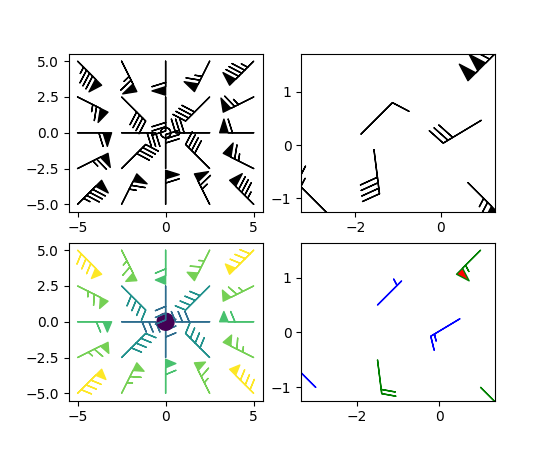(png, pdf)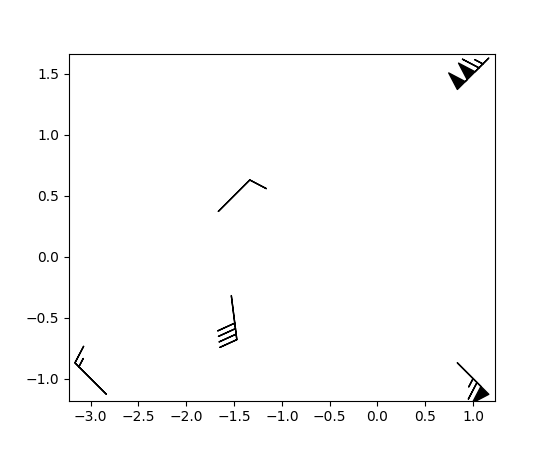(png, pdf)

Note

In addition to the above described arguments, this function can take a data keyword argument. If such a data argument is given, the following arguments are replaced by data[<arg>]:

• All positional and all keyword arguments.
`matplotlib.pyplot.``barh`(bottom, width, height=0.8, left=None, hold=None, **kwargs)

Make a horizontal bar plot.

Make a horizontal bar plot with rectangles bounded by:

`left`, `left` + `width`, `bottom`, `bottom` + `height`
(left, right, bottom and top edges)

`bottom`, `width`, `height`, and `left` can be either scalars or sequences

Parameters: Returns: bottom : scalar or array-like the y coordinate(s) of the bars width : scalar or array-like the width(s) of the bars height : sequence of scalars, optional, default: 0.8 the heights of the bars left : sequence of scalars the x coordinates of the left sides of the bars `matplotlib.patches.Rectangle` instances. color : scalar or array-like, optional the colors of the bars edgecolor : scalar or array-like, optional the colors of the bar edges linewidth : scalar or array-like, optional, default: None width of bar edge(s). If None, use default linewidth; If 0, don’t draw edges. tick_label : string or array-like, optional, default: None the tick labels of the bars xerr : scalar or array-like, optional, default: None if not None, will be used to generate errorbar(s) on the bar chart yerr : scalar or array-like, optional, default: None if not None, will be used to generate errorbar(s) on the bar chart ecolor : scalar or array-like, optional, default: None specifies the color of errorbar(s) capsize : scalar, optional determines the length in points of the error bar caps default: None, which will take the value from the `errorbar.capsize` `rcParam`. error_kw : dictionary of kwargs to be passed to errorbar method. `ecolor` and `capsize` may be specified here rather than as independent kwargs. align : {‘center’, ‘edge’}, optional If ‘edge’, aligns bars by their left edges (for vertical bars) and by their bottom edges (for horizontal bars). If ‘center’, interpret the `bottom` argument as the coordinates of the centers of the bars. To align on the align bars on the top edge pass a negative ‘height’. log : boolean, optional, default: False If true, sets the axis to be log scale

`bar`
Plot a vertical bar plot.

Notes

The optional arguments `color`, `edgecolor`, `linewidth`, `xerr`, and `yerr` can be either scalars or sequences of length equal to the number of bars. This enables you to use bar as the basis for stacked bar charts, or candlestick plots. Detail: `xerr` and `yerr` are passed directly to `errorbar()`, so they can also have shape 2xN for independent specification of lower and upper errors.

Other optional kwargs:

Property Description
`agg_filter` unknown
`alpha` float or None
`animated` [True | False]
`antialiased` or aa [True | False] or None for default
`axes` an `Axes` instance
`capstyle` [‘butt’ | ‘round’ | ‘projecting’]
`clip_box` a `matplotlib.transforms.Bbox` instance
`clip_on` [True | False]
`clip_path` [ (`Path`, `Transform`) | `Patch` | None ]
`color` matplotlib color spec
`contains` a callable function
`edgecolor` or ec mpl color spec, None, ‘none’, or ‘auto’
`facecolor` or fc mpl color spec, or None for default, or ‘none’ for no color
`figure` a `matplotlib.figure.Figure` instance
`fill` [True | False]
`gid` an id string
`hatch` [‘/’ | ‘\’ | ‘|’ | ‘-‘ | ‘+’ | ‘x’ | ‘o’ | ‘O’ | ‘.’ | ‘*’]
`joinstyle` [‘miter’ | ‘round’ | ‘bevel’]
`label` string or anything printable with ‘%s’ conversion.
`linestyle` or ls [‘solid’ | ‘dashed’, ‘dashdot’, ‘dotted’ | (offset, on-off-dash-seq) | `'-'` | `'--'` | `'-.'` | `':'` | `'None'` | `' '` | `''`]
`linewidth` or lw float or None for default
`path_effects` unknown
`picker` [None|float|boolean|callable]
`rasterized` [True | False | None]
`sketch_params` unknown
`snap` unknown
`transform` `Transform` instance
`url` a url string
`visible` [True | False]
`zorder` any number
`matplotlib.pyplot.``bone`()

set the default colormap to bone and apply to current image if any. See help(colormaps) for more information

`matplotlib.pyplot.``box`(on=None)

Turn the axes box on or off. on may be a boolean or a string, ‘on’ or ‘off’.

If on is None, toggle state.

`matplotlib.pyplot.``boxplot`(x, notch=None, sym=None, vert=None, whis=None, positions=None, widths=None, patch_artist=None, bootstrap=None, usermedians=None, conf_intervals=None, meanline=None, showmeans=None, showcaps=None, showbox=None, showfliers=None, boxprops=None, labels=None, flierprops=None, medianprops=None, meanprops=None, capprops=None, whiskerprops=None, manage_xticks=True, autorange=False, zorder=None, hold=None, data=None)

Make a box and whisker plot.

Make a box and whisker plot for each column of `x` or each vector in sequence `x`. The box extends from the lower to upper quartile values of the data, with a line at the median. The whiskers extend from the box to show the range of the data. Flier points are those past the end of the whiskers.

Parameters: Returns: x : Array or a sequence of vectors. The input data. notch : bool, optional (False) If `True`, will produce a notched box plot. Otherwise, a rectangular boxplot is produced. The notches represent the confidence interval (CI) around the median. See the entry for the `bootstrap` parameter for information regarding how the locations of the notches are computed. Note In cases where the values of the CI are less than the lower quartile or greater than the upper quartile, the notches will extend beyond the box, giving it a distinctive “flipped” appearance. This is expected behavior and consistent with other statistical visualization packages. sym : str, optional The default symbol for flier points. Enter an empty string (‘’) if you don’t want to show fliers. If `None`, then the fliers default to ‘b+’ If you want more control use the flierprops kwarg. vert : bool, optional (True) If `True` (default), makes the boxes vertical. If `False`, everything is drawn horizontally. whis : float, sequence, or string (default = 1.5) As a float, determines the reach of the whiskers to the beyond the first and third quartiles. In other words, where IQR is the interquartile range (`Q3-Q1`), the upper whisker will extend to last datum less than `Q3 + whis*IQR`). Similarly, the lower whisker will extend to the first datum greater than `Q1 - whis*IQR`. Beyond the whiskers, data are considered outliers and are plotted as individual points. Set this to an unreasonably high value to force the whiskers to show the min and max values. Alternatively, set this to an ascending sequence of percentile (e.g., [5, 95]) to set the whiskers at specific percentiles of the data. Finally, `whis` can be the string `'range'` to force the whiskers to the min and max of the data. bootstrap : int, optional Specifies whether to bootstrap the confidence intervals around the median for notched boxplots. If `bootstrap` is None, no bootstrapping is performed, and notches are calculated using a Gaussian-based asymptotic approximation (see McGill, R., Tukey, J.W., and Larsen, W.A., 1978, and Kendall and Stuart, 1967). Otherwise, bootstrap specifies the number of times to bootstrap the median to determine its 95% confidence intervals. Values between 1000 and 10000 are recommended. usermedians : array-like, optional An array or sequence whose first dimension (or length) is compatible with `x`. This overrides the medians computed by matplotlib for each element of `usermedians` that is not `None`. When an element of `usermedians` is None, the median will be computed by matplotlib as normal. conf_intervals : array-like, optional Array or sequence whose first dimension (or length) is compatible with `x` and whose second dimension is 2. When the an element of `conf_intervals` is not None, the notch locations computed by matplotlib are overridden (provided `notch` is `True`). When an element of `conf_intervals` is `None`, the notches are computed by the method specified by the other kwargs (e.g., `bootstrap`). positions : array-like, optional Sets the positions of the boxes. The ticks and limits are automatically set to match the positions. Defaults to `range(1, N+1)` where N is the number of boxes to be drawn. widths : scalar or array-like Sets the width of each box either with a scalar or a sequence. The default is 0.5, or ```0.15*(distance between extreme positions)```, if that is smaller. patch_artist : bool, optional (False) If `False` produces boxes with the Line2D artist. Otherwise, boxes and drawn with Patch artists. labels : sequence, optional Labels for each dataset. Length must be compatible with dimensions of `x`. manage_xticks : bool, optional (True) If the function should adjust the xlim and xtick locations. autorange : bool, optional (False) When `True` and the data are distributed such that the 25th and 75th percentiles are equal, `whis` is set to `'range'` such that the whisker ends are at the minimum and maximum of the data. meanline : bool, optional (False) If `True` (and `showmeans` is `True`), will try to render the mean as a line spanning the full width of the box according to `meanprops` (see below). Not recommended if `shownotches` is also True. Otherwise, means will be shown as points. zorder : scalar, optional (None) Sets the zorder of the boxplot. result : dict A dictionary mapping each component of the boxplot to a list of the `matplotlib.lines.Line2D` instances created. That dictionary has the following keys (assuming vertical boxplots): `boxes`: the main body of the boxplot showing the quartiles and the median’s confidence intervals if enabled. `medians`: horizontal lines at the median of each box. `whiskers`: the vertical lines extending to the most extreme, non-outlier data points. `caps`: the horizontal lines at the ends of the whiskers. `fliers`: points representing data that extend beyond the whiskers (fliers). `means`: points or lines representing the means. showcaps : bool, optional (True) Show the caps on the ends of whiskers. showbox : bool, optional (True) Show the central box. showfliers : bool, optional (True) Show the outliers beyond the caps. showmeans : bool, optional (False) Show the arithmetic means. capprops : dict, optional (None) Specifies the style of the caps. boxprops : dict, optional (None) Specifies the style of the box. whiskerprops : dict, optional (None) Specifies the style of the whiskers. flierprops : dict, optional (None) Specifies the style of the fliers. medianprops : dict, optional (None) Specifies the style of the median. meanprops : dict, optional (None) Specifies the style of the mean.

Examples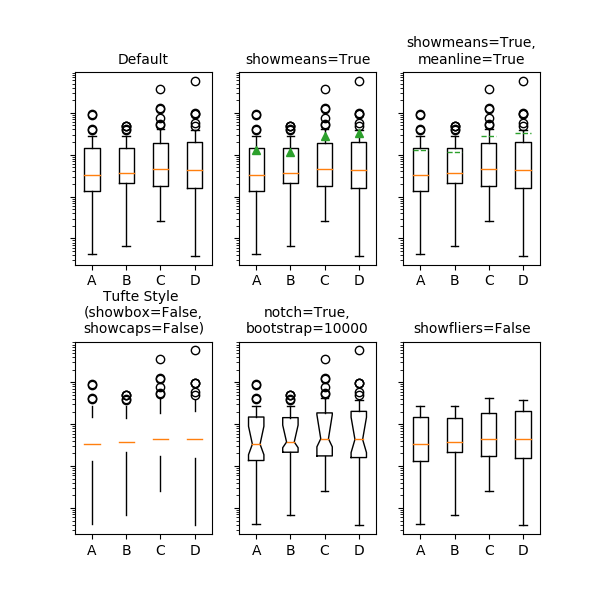(png, pdf)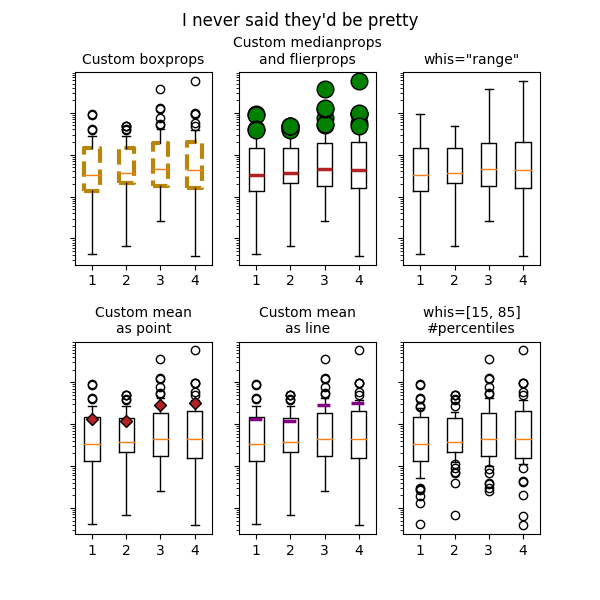Note

In addition to the above described arguments, this function can take a data keyword argument. If such a data argument is given, the following arguments are replaced by data[<arg>]:

• All positional and all keyword arguments.
`matplotlib.pyplot.``broken_barh`(xranges, yrange, hold=None, data=None, **kwargs)

Plot horizontal bars.

A collection of horizontal bars spanning yrange with a sequence of xranges.

Required arguments:

Argument Description
xranges sequence of (xmin, xwidth)
yrange sequence of (ymin, ywidth)

kwargs are `matplotlib.collections.BrokenBarHCollection` properties:

Property Description
`agg_filter` unknown
`alpha` float or None
`animated` [True | False]
`antialiased` or antialiaseds Boolean or sequence of booleans
`array` unknown
`axes` an `Axes` instance
`clim` a length 2 sequence of floats
`clip_box` a `matplotlib.transforms.Bbox` instance
`clip_on` [True | False]
`clip_path` [ (`Path`, `Transform`) | `Patch` | None ]
`cmap` a colormap or registered colormap name
`color` matplotlib color arg or sequence of rgba tuples
`contains` a callable function
`edgecolor` or edgecolors matplotlib color spec or sequence of specs
`facecolor` or facecolors matplotlib color spec or sequence of specs
`figure` a `matplotlib.figure.Figure` instance
`gid` an id string
`hatch` [ ‘/’ | ‘\’ | ‘|’ | ‘-‘ | ‘+’ | ‘x’ | ‘o’ | ‘O’ | ‘.’ | ‘*’ ]
`label` string or anything printable with ‘%s’ conversion.
`linestyle` or dashes or linestyles [‘solid’ | ‘dashed’, ‘dashdot’, ‘dotted’ | (offset, on-off-dash-seq) | `'-'` | `'--'` | `'-.'` | `':'` | `'None'` | `' '` | `''`]
`linewidth` or linewidths or lw float or sequence of floats
`norm` unknown
`offset_position` unknown
`offsets` float or sequence of floats
`path_effects` unknown
`picker` [None|float|boolean|callable]
`pickradius` unknown
`rasterized` [True | False | None]
`sketch_params` unknown
`snap` unknown
`transform` `Transform` instance
`url` a url string
`urls` unknown
`visible` [True | False]
`zorder` any number

these can either be a single argument, i.e.,:

```facecolors = 'black'
```

or a sequence of arguments for the various bars, i.e.,:

```facecolors = ('black', 'red', 'green')
```

Example: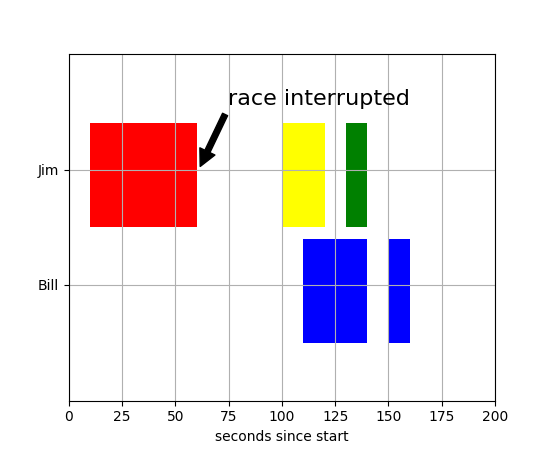Note

In addition to the above described arguments, this function can take a data keyword argument. If such a data argument is given, the following arguments are replaced by data[<arg>]:

• All positional and all keyword arguments.
`matplotlib.pyplot.``cla`()

Clear the current axes.

`matplotlib.pyplot.``clabel`(CS, *args, **kwargs)

Label a contour plot.

Call signature:

```clabel(cs, **kwargs)
```

Adds labels to line contours in cs, where cs is a `ContourSet` object returned by contour.

```clabel(cs, v, **kwargs)
```

only labels contours listed in v.

Optional keyword arguments:

fontsize:
size in points or relative size e.g., ‘smaller’, ‘x-large’
colors:
• if None, the color of each label matches the color of the corresponding contour
• if one string color, e.g., colors = ‘r’ or colors = ‘red’, all labels will be plotted in this color
• if a tuple of matplotlib color args (string, float, rgb, etc), different labels will be plotted in different colors in the order specified
inline:
controls whether the underlying contour is removed or not. Default is True.
inline_spacing:
space in pixels to leave on each side of label when placing inline. Defaults to 5. This spacing will be exact for labels at locations where the contour is straight, less so for labels on curved contours.
fmt:
a format string for the label. Default is ‘%1.3f’ Alternatively, this can be a dictionary matching contour levels with arbitrary strings to use for each contour level (i.e., fmt[level]=string), or it can be any callable, such as a `Formatter` instance, that returns a string when called with a numeric contour level.
manual:

if True, contour labels will be placed manually using mouse clicks. Click the first button near a contour to add a label, click the second button (or potentially both mouse buttons at once) to finish adding labels. The third button can be used to remove the last label added, but only if labels are not inline. Alternatively, the keyboard can be used to select label locations (enter to end label placement, delete or backspace act like the third mouse button, and any other key will select a label location).

manual can be an iterable object of x,y tuples. Contour labels will be created as if mouse is clicked at each x,y positions.

rightside_up:
if True (default), label rotations will always be plus or minus 90 degrees from level.
use_clabeltext:
if True (default is False), ClabelText class (instead of matplotlib.Text) is used to create labels. ClabelText recalculates rotation angles of texts during the drawing time, therefore this can be used if aspect of the axes changes.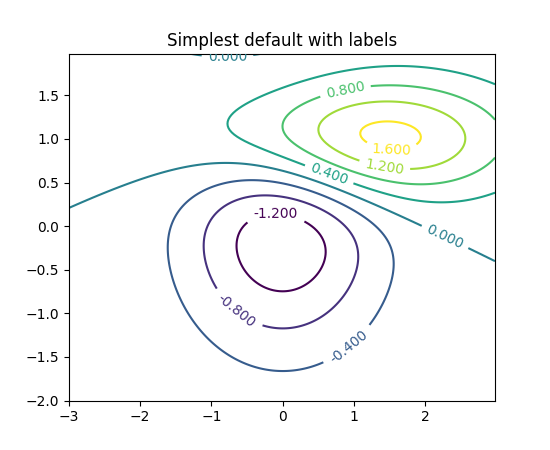(png, pdf)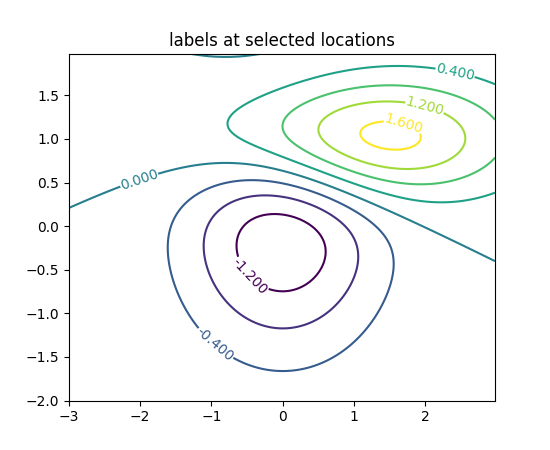(png, pdf)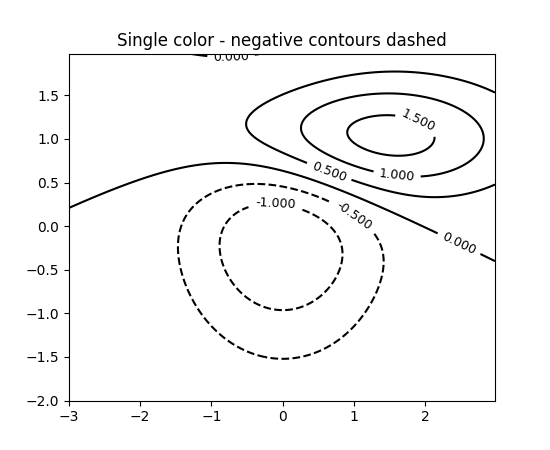(png, pdf)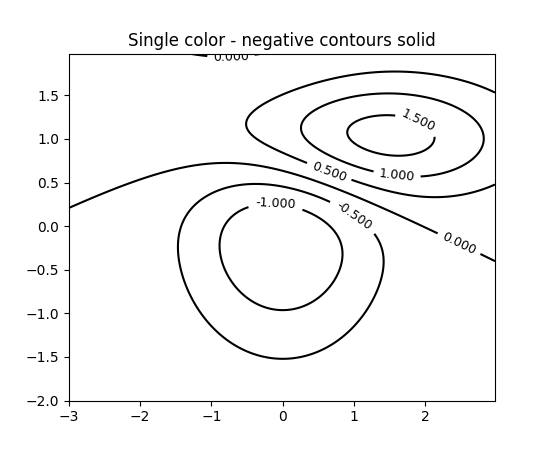(png, pdf)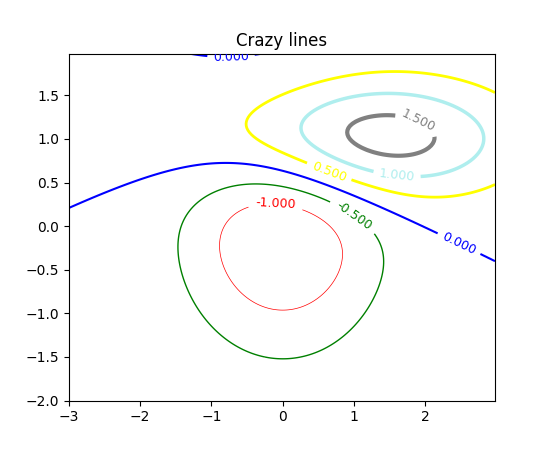(png, pdf)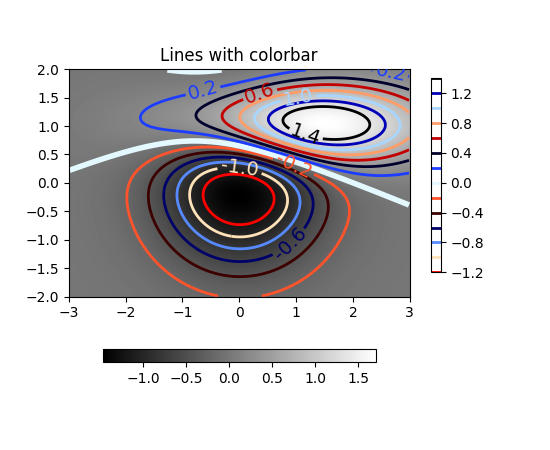(png, pdf)

`matplotlib.pyplot.``clf`()

Clear the current figure.

`matplotlib.pyplot.``clim`(vmin=None, vmax=None)

Set the color limits of the current image.

To apply clim to all axes images do:

```clim(0, 0.5)
```

If either vmin or vmax is None, the image min/max respectively will be used for color scaling.

If you want to set the clim of multiple images, use, for example:

```for im in gca().get_images():
im.set_clim(0, 0.05)
```
`matplotlib.pyplot.``close`(*args)

Close a figure window.

`close()` by itself closes the current figure

`close(h)` where h is a `Figure` instance, closes that figure

`close(num)` closes figure number num

`close(name)` where name is a string, closes figure with that label

`close('all')` closes all the figure windows

`matplotlib.pyplot.``cohere`(x, y, NFFT=256, Fs=2, Fc=0, detrend=<function detrend_none>, window=<function window_hanning>, noverlap=0, pad_to=None, sides='default', scale_by_freq=None, hold=None, data=None, **kwargs)

Plot the coherence between x and y.

Plot the coherence between x and y. Coherence is the normalized cross spectral density: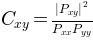Parameters:

Fs : scalar

The sampling frequency (samples per time unit). It is used to calculate the Fourier frequencies, freqs, in cycles per time unit. The default value is 2.

window : callable or ndarray

A function or a vector of length NFFT. To create window vectors see `window_hanning()`, `window_none()`, `numpy.blackman()`, `numpy.hamming()`, `numpy.bartlett()`, `scipy.signal()`, `scipy.signal.get_window()`, etc. The default is `window_hanning()`. If a function is passed as the argument, it must take a data segment as an argument and return the windowed version of the segment.

sides : [ ‘default’ | ‘onesided’ | ‘twosided’ ]

Specifies which sides of the spectrum to return. Default gives the default behavior, which returns one-sided for real data and both for complex data. ‘onesided’ forces the return of a one-sided spectrum, while ‘twosided’ forces two-sided.

The number of points to which the data segment is padded when performing the FFT. This can be different from NFFT, which specifies the number of data points used. While not increasing the actual resolution of the spectrum (the minimum distance between resolvable peaks), this can give more points in the plot, allowing for more detail. This corresponds to the n parameter in the call to fft(). The default is None, which sets pad_to equal to NFFT

NFFT : integer

The number of data points used in each block for the FFT. A power 2 is most efficient. The default value is 256. This should NOT be used to get zero padding, or the scaling of the result will be incorrect. Use pad_to for this instead.

detrend : {‘default’, ‘constant’, ‘mean’, ‘linear’, ‘none’} or callable

The function applied to each segment before fft-ing, designed to remove the mean or linear trend. Unlike in MATLAB, where the detrend parameter is a vector, in matplotlib is it a function. The `pylab` module defines `detrend_none()`, `detrend_mean()`, and `detrend_linear()`, but you can use a custom function as well. You can also use a string to choose one of the functions. ‘default’, ‘constant’, and ‘mean’ call `detrend_mean()`. ‘linear’ calls `detrend_linear()`. ‘none’ calls `detrend_none()`.

scale_by_freq : boolean, optional

Specifies whether the resulting density values should be scaled by the scaling frequency, which gives density in units of Hz^-1. This allows for integration over the returned frequency values. The default is True for MATLAB compatibility.

noverlap : integer

The number of points of overlap between blocks. The default value is 0 (no overlap).

Fc : integer

The center frequency of x (defaults to 0), which offsets the x extents of the plot to reflect the frequency range used when a signal is acquired and then filtered and downsampled to baseband.

**kwargs :

Keyword arguments control the `Line2D` properties of the coherence plot:

Property Description
`agg_filter` unknown
`alpha` float (0.0 transparent through 1.0 opaque)
`animated` [True | False]
`antialiased` or aa [True | False]
`axes` an `Axes` instance
`clip_box` a `matplotlib.transforms.Bbox` instance
`clip_on` [True | False]
`clip_path` [ (`Path`, `Transform`) | `Patch` | None ]
`color` or c any matplotlib color
`contains` a callable function
`dash_capstyle` [‘butt’ | ‘round’ | ‘projecting’]
`dash_joinstyle` [‘miter’ | ‘round’ | ‘bevel’]
`dashes` sequence of on/off ink in points
`drawstyle` [‘default’ | ‘steps’ | ‘steps-pre’ | ‘steps-mid’ | ‘steps-post’]
`figure` a `matplotlib.figure.Figure` instance
`fillstyle` [‘full’ | ‘left’ | ‘right’ | ‘bottom’ | ‘top’ | ‘none’]
`gid` an id string
`label` string or anything printable with ‘%s’ conversion.
`linestyle` or ls [‘solid’ | ‘dashed’, ‘dashdot’, ‘dotted’ | (offset, on-off-dash-seq) | `'-'` | `'--'` | `'-.'` | `':'` | `'None'` | `' '` | `''`]
`linewidth` or lw float value in points
`marker` `A valid marker style`
`markeredgecolor` or mec any matplotlib color
`markeredgewidth` or mew float value in points
`markerfacecolor` or mfc any matplotlib color
`markerfacecoloralt` or mfcalt any matplotlib color
`markersize` or ms float
`markevery` [None | int | length-2 tuple of int | slice | list/array of int | float | length-2 tuple of float]
`path_effects` unknown
`picker` float distance in points or callable pick function `fn(artist, event)`
`pickradius` float distance in points
`rasterized` [True | False | None]
`sketch_params` unknown
`snap` unknown
`solid_capstyle` [‘butt’ | ‘round’ | ‘projecting’]
`solid_joinstyle` [‘miter’ | ‘round’ | ‘bevel’]
`transform` a `matplotlib.transforms.Transform` instance
`url` a url string
`visible` [True | False]
`xdata` 1D array
`ydata` 1D array
`zorder` any number
Returns:

The return value is a tuple (Cxy, f), where f are the

frequencies of the coherence vector.

kwargs are applied to the lines.

References

Bendat & Piersol – Random Data: Analysis and Measurement Procedures, John Wiley & Sons (1986)

Examples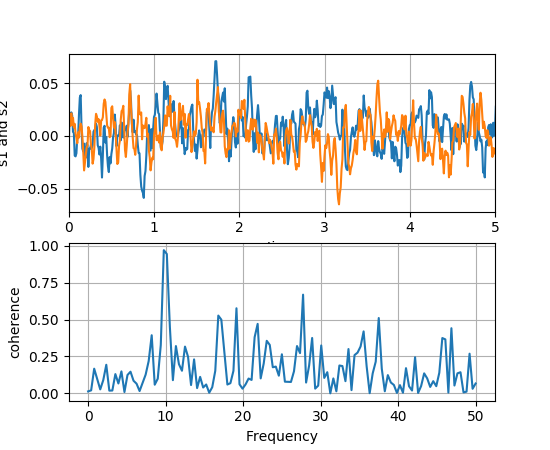Note

In addition to the above described arguments, this function can take a data keyword argument. If such a data argument is given, the following arguments are replaced by data[<arg>]:

• All arguments with the following names: ‘x’, ‘y’.
`matplotlib.pyplot.``colorbar`(mappable=None, cax=None, ax=None, **kw)

Add a colorbar to a plot.

Function signatures for the `pyplot` interface; all but the first are also method signatures for the `colorbar()` method:

```colorbar(**kwargs)
colorbar(mappable, **kwargs)
colorbar(mappable, cax=cax, **kwargs)
colorbar(mappable, ax=ax, **kwargs)
```

arguments:

mappable
the `Image`, `ContourSet`, etc. to which the colorbar applies; this argument is mandatory for the `colorbar()` method but optional for the `colorbar()` function, which sets the default to the current image.

keyword arguments:

cax
None | axes object into which the colorbar will be drawn
ax
None | parent axes object(s) from which space for a new colorbar axes will be stolen. If a list of axes is given they will all be resized to make room for the colorbar axes.
use_gridspec
False | If cax is None, a new cax is created as an instance of Axes. If ax is an instance of Subplot and use_gridspec is True, cax is created as an instance of Subplot using the grid_spec module.

Additional keyword arguments are of two kinds:

axes properties:

Property Description
orientation vertical or horizontal
fraction 0.15; fraction of original axes to use for colorbar
pad 0.05 if vertical, 0.15 if horizontal; fraction of original axes between colorbar and new image axes
shrink 1.0; fraction by which to shrink the colorbar
aspect 20; ratio of long to short dimensions
anchor (0.0, 0.5) if vertical; (0.5, 1.0) if horizontal; the anchor point of the colorbar axes
panchor (1.0, 0.5) if vertical; (0.5, 0.0) if horizontal; the anchor point of the colorbar parent axes. If False, the parent axes’ anchor will be unchanged

colorbar properties:

Property Description
extend [ ‘neither’ | ‘both’ | ‘min’ | ‘max’ ] If not ‘neither’, make pointed end(s) for out-of- range values. These are set for a given colormap using the colormap set_under and set_over methods.
extendfrac [ None | ‘auto’ | length | lengths ] If set to None, both the minimum and maximum triangular colorbar extensions with have a length of 5% of the interior colorbar length (this is the default setting). If set to ‘auto’, makes the triangular colorbar extensions the same lengths as the interior boxes (when spacing is set to ‘uniform’) or the same lengths as the respective adjacent interior boxes (when spacing is set to ‘proportional’). If a scalar, indicates the length of both the minimum and maximum triangular colorbar extensions as a fraction of the interior colorbar length. A two-element sequence of fractions may also be given, indicating the lengths of the minimum and maximum colorbar extensions respectively as a fraction of the interior colorbar length.
extendrect [ False | True ] If False the minimum and maximum colorbar extensions will be triangular (the default). If True the extensions will be rectangular.
spacing [ ‘uniform’ | ‘proportional’ ] Uniform spacing gives each discrete color the same space; proportional makes the space proportional to the data interval.
ticks [ None | list of ticks | Locator object ] If None, ticks are determined automatically from the input.
format [ None | format string | Formatter object ] If None, the `ScalarFormatter` is used. If a format string is given, e.g., ‘%.3f’, that is used. An alternative `Formatter` object may be given instead.
drawedges [ False | True ] If true, draw lines at color boundaries.

The following will probably be useful only in the context of indexed colors (that is, when the mappable has norm=NoNorm()), or other unusual circumstances.

Property Description
boundaries None or a sequence
values None or a sequence which must be of length 1 less than the sequence of boundaries. For each region delimited by adjacent entries in boundaries, the color mapped to the corresponding value in values will be used.

If mappable is a `ContourSet`, its extend kwarg is included automatically.

Note that the shrink kwarg provides a simple way to keep a vertical colorbar, for example, from being taller than the axes of the mappable to which the colorbar is attached; but it is a manual method requiring some trial and error. If the colorbar is too tall (or a horizontal colorbar is too wide) use a smaller value of shrink.

For more precise control, you can manually specify the positions of the axes objects in which the mappable and the colorbar are drawn. In this case, do not use any of the axes properties kwargs.

It is known that some vector graphics viewer (svg and pdf) renders white gaps between segments of the colorbar. This is due to bugs in the viewers not matplotlib. As a workaround the colorbar can be rendered with overlapping segments:

```cbar = colorbar()
cbar.solids.set_edgecolor("face")
draw()
```

However this has negative consequences in other circumstances. Particularly with semi transparent images (alpha < 1) and colorbar extensions and is not enabled by default see (issue #1188).

returns:
`Colorbar` instance; see also its base class, `ColorbarBase`. Call the `set_label()` method to label the colorbar.
`matplotlib.pyplot.``colors`()

This is a do-nothing function to provide you with help on how matplotlib handles colors.

Commands which take color arguments can use several formats to specify the colors. For the basic built-in colors, you can use a single letter

Alias Color
‘b’ blue
‘g’ green
‘r’ red
‘c’ cyan
‘m’ magenta
‘y’ yellow
‘k’ black
‘w’ white

For a greater range of colors, you have two options. You can specify the color using an html hex string, as in:

```color = '#eeefff'
```

or you can pass an R,G,B tuple, where each of R,G,B are in the range [0,1].

You can also use any legal html name for a color, for example:

```color = 'red'
color = 'burlywood'
color = 'chartreuse'
```

The example below creates a subplot with a dark slate gray background:

```subplot(111, facecolor=(0.1843, 0.3098, 0.3098))
```

Here is an example that creates a pale turquoise title:

```title('Is this the best color?', color='#afeeee')
```
`matplotlib.pyplot.``connect`(s, func)

Connect event with string s to func. The signature of func is:

```def func(event)
```

where event is a `matplotlib.backend_bases.Event`. The following events are recognized

• ‘button_press_event’
• ‘button_release_event’
• ‘draw_event’
• ‘key_press_event’
• ‘key_release_event’
• ‘motion_notify_event’
• ‘pick_event’
• ‘resize_event’
• ‘scroll_event’
• ‘figure_enter_event’,
• ‘figure_leave_event’,
• ‘axes_enter_event’,
• ‘axes_leave_event’
• ‘close_event’

For the location events (button and key press/release), if the mouse is over the axes, the variable `event.inaxes` will be set to the `Axes` the event occurs is over, and additionally, the variables `event.xdata` and `event.ydata` will be defined. This is the mouse location in data coords. See `KeyEvent` and `MouseEvent` for more info.

Return value is a connection id that can be used with `mpl_disconnect()`.

Example usage:

```def on_press(event):
print('you pressed', event.button, event.xdata, event.ydata)

cid = canvas.mpl_connect('button_press_event', on_press)
```
`matplotlib.pyplot.``contour`(*args, **kwargs)

Plot contours.

`contour()` and `contourf()` draw contour lines and filled contours, respectively. Except as noted, function signatures and return values are the same for both versions.

`contourf()` differs from the MATLAB version in that it does not draw the polygon edges. To draw edges, add line contours with calls to `contour()`.

Call signatures:

```contour(Z)
```

make a contour plot of an array Z. The level values are chosen automatically.

```contour(X,Y,Z)
```

X, Y specify the (x, y) coordinates of the surface

```contour(Z,N)
contour(X,Y,Z,N)
```

contour up to N automatically-chosen levels.

```contour(Z,V)
contour(X,Y,Z,V)
```

draw contour lines at the values specified in sequence V, which must be in increasing order.

```contourf(..., V)
```

fill the `len(V)-1` regions between the values in V, which must be in increasing order.

```contour(Z, **kwargs)
```

Use keyword args to control colors, linewidth, origin, cmap ... see below for more details.

X and Y must both be 2-D with the same shape as Z, or they must both be 1-D such that `len(X)` is the number of columns in Z and `len(Y)` is the number of rows in Z.

`C = contour(...)` returns a `QuadContourSet` object.

Optional keyword arguments:

corner_mask: [ True | False | ‘legacy’ ]

Enable/disable corner masking, which only has an effect if Z is a masked array. If False, any quad touching a masked point is masked out. If True, only the triangular corners of quads nearest those points are always masked out, other triangular corners comprising three unmasked points are contoured as usual. If ‘legacy’, the old contouring algorithm is used, which is equivalent to False and is deprecated, only remaining whilst the new algorithm is tested fully.

If not specified, the default is taken from rcParams[‘contour.corner_mask’], which is True unless it has been modified.

colors: [ None | string | (mpl_colors) ]

If None, the colormap specified by cmap will be used.

If a string, like ‘r’ or ‘red’, all levels will be plotted in this color.

If a tuple of matplotlib color args (string, float, rgb, etc), different levels will be plotted in different colors in the order specified.

alpha: float
The alpha blending value
cmap: [ None | Colormap ]
A cm `Colormap` instance or None. If cmap is None and colors is None, a default Colormap is used.
norm: [ None | Normalize ]
A `matplotlib.colors.Normalize` instance for scaling data values to colors. If norm is None and colors is None, the default linear scaling is used.
vmin, vmax: [ None | scalar ]
If not None, either or both of these values will be supplied to the `matplotlib.colors.Normalize` instance, overriding the default color scaling based on levels.
levels: [level0, level1, ..., leveln]
A list of floating point numbers indicating the level curves to draw, in increasing order; e.g., to draw just the zero contour pass `levels=`
origin: [ None | ‘upper’ | ‘lower’ | ‘image’ ]

If None, the first value of Z will correspond to the lower left corner, location (0,0). If ‘image’, the rc value for `image.origin` will be used.

This keyword is not active if X and Y are specified in the call to contour.

extent: [ None | (x0,x1,y0,y1) ]

If origin is not None, then extent is interpreted as in `matplotlib.pyplot.imshow()`: it gives the outer pixel boundaries. In this case, the position of Z[0,0] is the center of the pixel, not a corner. If origin is None, then (x0, y0) is the position of Z[0,0], and (x1, y1) is the position of Z[-1,-1].

This keyword is not active if X and Y are specified in the call to contour.

locator: [ None | ticker.Locator subclass ]
If locator is None, the default `MaxNLocator` is used. The locator is used to determine the contour levels if they are not given explicitly via the V argument.
extend: [ ‘neither’ | ‘both’ | ‘min’ | ‘max’ ]
Unless this is ‘neither’, contour levels are automatically added to one or both ends of the range so that all data are included. These added ranges are then mapped to the special colormap values which default to the ends of the colormap range, but can be set via `matplotlib.colors.Colormap.set_under()` and `matplotlib.colors.Colormap.set_over()` methods.
xunits, yunits: [ None | registered units ]
Override axis units by specifying an instance of a `matplotlib.units.ConversionInterface`.
antialiased: [ True | False ]
enable antialiasing, overriding the defaults. For filled contours, the default is True. For line contours, it is taken from rcParams[‘lines.antialiased’].
nchunk: [ 0 | integer ]
If 0, no subdivision of the domain. Specify a positive integer to divide the domain into subdomains of nchunk by nchunk quads. Chunking reduces the maximum length of polygons generated by the contouring algorithm which reduces the rendering workload passed on to the backend and also requires slightly less RAM. It can however introduce rendering artifacts at chunk boundaries depending on the backend, the antialiased flag and value of alpha.

contour-only keyword arguments:

linewidths: [ None | number | tuple of numbers ]

If linewidths is None, the default width in `lines.linewidth` in `matplotlibrc` is used.

If a number, all levels will be plotted with this linewidth.

If a tuple, different levels will be plotted with different linewidths in the order specified.

linestyles: [ None | ‘solid’ | ‘dashed’ | ‘dashdot’ | ‘dotted’ ]

If linestyles is None, the default is ‘solid’ unless the lines are monochrome. In that case, negative contours will take their linestyle from the `matplotlibrc` `contour.negative_linestyle` setting.

linestyles can also be an iterable of the above strings specifying a set of linestyles to be used. If this iterable is shorter than the number of contour levels it will be repeated as necessary.

contourf-only keyword arguments:

hatches:
A list of cross hatch patterns to use on the filled areas. If None, no hatching will be added to the contour. Hatching is supported in the PostScript, PDF, SVG and Agg backends only.

Note: contourf fills intervals that are closed at the top; that is, for boundaries z1 and z2, the filled region is:

```z1 < z <= z2
```

There is one exception: if the lowest boundary coincides with the minimum value of the z array, then that minimum value will be included in the lowest interval.

Examples:(png, pdf)(png, pdf)(png, pdf)(png, pdf)(png, pdf)(png, pdf)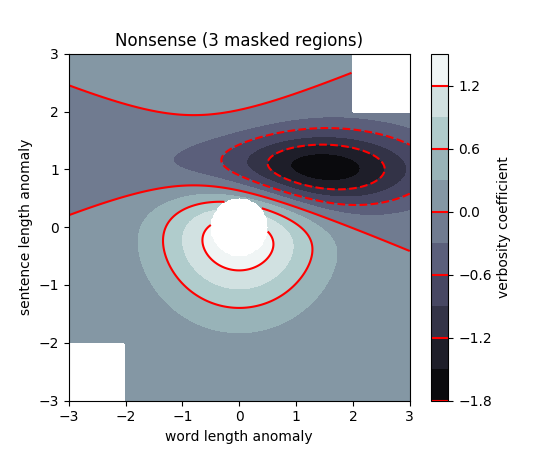(png, pdf)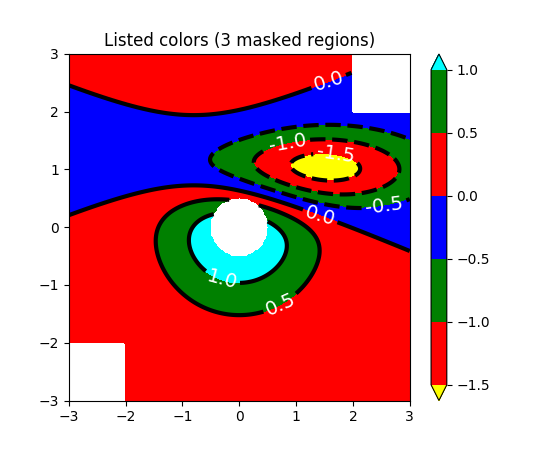(png, pdf)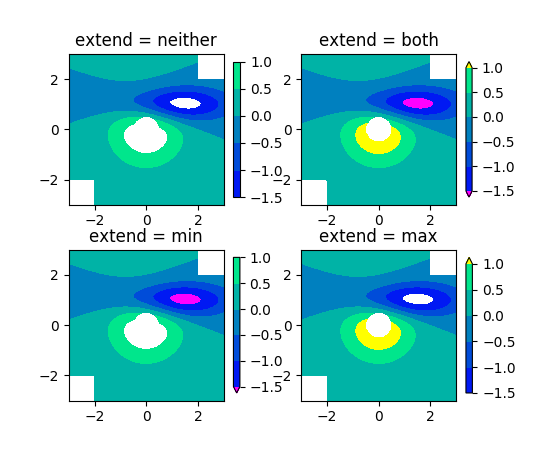(png, pdf)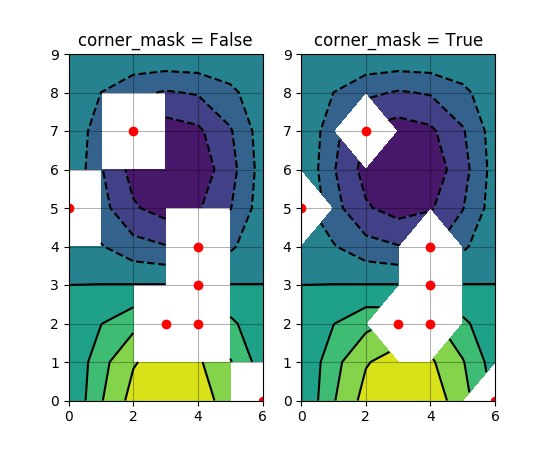`matplotlib.pyplot.``contourf`(*args, **kwargs)

Plot contours.

`contour()` and `contourf()` draw contour lines and filled contours, respectively. Except as noted, function signatures and return values are the same for both versions.

`contourf()` differs from the MATLAB version in that it does not draw the polygon edges. To draw edges, add line contours with calls to `contour()`.

Call signatures:

```contour(Z)
```

make a contour plot of an array Z. The level values are chosen automatically.

```contour(X,Y,Z)
```

X, Y specify the (x, y) coordinates of the surface

```contour(Z,N)
contour(X,Y,Z,N)
```

contour up to N automatically-chosen levels.

```contour(Z,V)
contour(X,Y,Z,V)
```

draw contour lines at the values specified in sequence V, which must be in increasing order.

```contourf(..., V)
```

fill the `len(V)-1` regions between the values in V, which must be in increasing order.

```contour(Z, **kwargs)
```

Use keyword args to control colors, linewidth, origin, cmap ... see below for more details.

X and Y must both be 2-D with the same shape as Z, or they must both be 1-D such that `len(X)` is the number of columns in Z and `len(Y)` is the number of rows in Z.

`C = contour(...)` returns a `QuadContourSet` object.

Optional keyword arguments:

corner_mask: [ True | False | ‘legacy’ ]

Enable/disable corner masking, which only has an effect if Z is a masked array. If False, any quad touching a masked point is masked out. If True, only the triangular corners of quads nearest those points are always masked out, other triangular corners comprising three unmasked points are contoured as usual. If ‘legacy’, the old contouring algorithm is used, which is equivalent to False and is deprecated, only remaining whilst the new algorithm is tested fully.

If not specified, the default is taken from rcParams[‘contour.corner_mask’], which is True unless it has been modified.

colors: [ None | string | (mpl_colors) ]

If None, the colormap specified by cmap will be used.

If a string, like ‘r’ or ‘red’, all levels will be plotted in this color.

If a tuple of matplotlib color args (string, float, rgb, etc), different levels will be plotted in different colors in the order specified.

alpha: float
The alpha blending value
cmap: [ None | Colormap ]
A cm `Colormap` instance or None. If cmap is None and colors is None, a default Colormap is used.
norm: [ None | Normalize ]
A `matplotlib.colors.Normalize` instance for scaling data values to colors. If norm is None and colors is None, the default linear scaling is used.
vmin, vmax: [ None | scalar ]
If not None, either or both of these values will be supplied to the `matplotlib.colors.Normalize` instance, overriding the default color scaling based on levels.
levels: [level0, level1, ..., leveln]
A list of floating point numbers indicating the level curves to draw, in increasing order; e.g., to draw just the zero contour pass `levels=`
origin: [ None | ‘upper’ | ‘lower’ | ‘image’ ]

If None, the first value of Z will correspond to the lower left corner, location (0,0). If ‘image’, the rc value for `image.origin` will be used.

This keyword is not active if X and Y are specified in the call to contour.

extent: [ None | (x0,x1,y0,y1) ]

If origin is not None, then extent is interpreted as in `matplotlib.pyplot.imshow()`: it gives the outer pixel boundaries. In this case, the position of Z[0,0] is the center of the pixel, not a corner. If origin is None, then (x0, y0) is the position of Z[0,0], and (x1, y1) is the position of Z[-1,-1].

This keyword is not active if X and Y are specified in the call to contour.

locator: [ None | ticker.Locator subclass ]
If locator is None, the default `MaxNLocator` is used. The locator is used to determine the contour levels if they are not given explicitly via the V argument.
extend: [ ‘neither’ | ‘both’ | ‘min’ | ‘max’ ]
Unless this is ‘neither’, contour levels are automatically added to one or both ends of the range so that all data are included. These added ranges are then mapped to the special colormap values which default to the ends of the colormap range, but can be set via `matplotlib.colors.Colormap.set_under()` and `matplotlib.colors.Colormap.set_over()` methods.
xunits, yunits: [ None | registered units ]
Override axis units by specifying an instance of a `matplotlib.units.ConversionInterface`.
antialiased: [ True | False ]
enable antialiasing, overriding the defaults. For filled contours, the default is True. For line contours, it is taken from rcParams[‘lines.antialiased’].
nchunk: [ 0 | integer ]
If 0, no subdivision of the domain. Specify a positive integer to divide the domain into subdomains of nchunk by nchunk quads. Chunking reduces the maximum length of polygons generated by the contouring algorithm which reduces the rendering workload passed on to the backend and also requires slightly less RAM. It can however introduce rendering artifacts at chunk boundaries depending on the backend, the antialiased flag and value of alpha.

contour-only keyword arguments:

linewidths: [ None | number | tuple of numbers ]

If linewidths is None, the default width in `lines.linewidth` in `matplotlibrc` is used.

If a number, all levels will be plotted with this linewidth.

If a tuple, different levels will be plotted with different linewidths in the order specified.

linestyles: [ None | ‘solid’ | ‘dashed’ | ‘dashdot’ | ‘dotted’ ]

If linestyles is None, the default is ‘solid’ unless the lines are monochrome. In that case, negative contours will take their linestyle from the `matplotlibrc` `contour.negative_linestyle` setting.

linestyles can also be an iterable of the above strings specifying a set of linestyles to be used. If this iterable is shorter than the number of contour levels it will be repeated as necessary.

contourf-only keyword arguments:

hatches:
A list of cross hatch patterns to use on the filled areas. If None, no hatching will be added to the contour. Hatching is supported in the PostScript, PDF, SVG and Agg backends only.

Note: contourf fills intervals that are closed at the top; that is, for boundaries z1 and z2, the filled region is:

```z1 < z <= z2
```

There is one exception: if the lowest boundary coincides with the minimum value of the z array, then that minimum value will be included in the lowest interval.

Examples:(png, pdf)(png, pdf)(png, pdf)(png, pdf)(png, pdf)(png, pdf)(png, pdf)(png, pdf)(png, pdf)`matplotlib.pyplot.``cool`()

set the default colormap to cool and apply to current image if any. See help(colormaps) for more information

`matplotlib.pyplot.``copper`()

set the default colormap to copper and apply to current image if any. See help(colormaps) for more information

`matplotlib.pyplot.``csd`(x, y, NFFT=None, Fs=None, Fc=None, detrend=None, window=None, noverlap=None, pad_to=None, sides=None, scale_by_freq=None, return_line=None, hold=None, data=None, **kwargs)

Plot the cross-spectral density.

Call signature:

```csd(x, y, NFFT=256, Fs=2, Fc=0, detrend=mlab.detrend_none,
sides='default', scale_by_freq=None, return_line=None, **kwargs)
```

The cross spectral density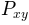by Welch’s average periodogram method. The vectors x and y are divided into NFFT length segments. Each segment is detrended by function detrend and windowed by function window. noverlap gives the length of the overlap between segments. The product of the direct FFTs of x and y are averaged over each segment to compute, with a scaling to correct for power loss due to windowing.

If len(x) < NFFT or len(y) < NFFT, they will be zero padded to NFFT.

Parameters:

x, y : 1-D arrays or sequences

Arrays or sequences containing the data

Fs : scalar

The sampling frequency (samples per time unit). It is used to calculate the Fourier frequencies, freqs, in cycles per time unit. The default value is 2.

window : callable or ndarray

A function or a vector of length NFFT. To create window vectors see `window_hanning()`, `window_none()`, `numpy.blackman()`, `numpy.hamming()`, `numpy.bartlett()`, `scipy.signal()`, `scipy.signal.get_window()`, etc. The default is `window_hanning()`. If a function is passed as the argument, it must take a data segment as an argument and return the windowed version of the segment.

sides : [ ‘default’ | ‘onesided’ | ‘twosided’ ]

Specifies which sides of the spectrum to return. Default gives the default behavior, which returns one-sided for real data and both for complex data. ‘onesided’ forces the return of a one-sided spectrum, while ‘twosided’ forces two-sided.

The number of points to which the data segment is padded when performing the FFT. This can be different from NFFT, which specifies the number of data points used. While not increasing the actual resolution of the spectrum (the minimum distance between resolvable peaks), this can give more points in the plot, allowing for more detail. This corresponds to the n parameter in the call to fft(). The default is None, which sets pad_to equal to NFFT

NFFT : integer

The number of data points used in each block for the FFT. A power 2 is most efficient. The default value is 256. This should NOT be used to get zero padding, or the scaling of the result will be incorrect. Use pad_to for this instead.

detrend : {‘default’, ‘constant’, ‘mean’, ‘linear’, ‘none’} or callable

The function applied to each segment before fft-ing, designed to remove the mean or linear trend. Unlike in MATLAB, where the detrend parameter is a vector, in matplotlib is it a function. The `pylab` module defines `detrend_none()`, `detrend_mean()`, and `detrend_linear()`, but you can use a custom function as well. You can also use a string to choose one of the functions. ‘default’, ‘constant’, and ‘mean’ call `detrend_mean()`. ‘linear’ calls `detrend_linear()`. ‘none’ calls `detrend_none()`.

scale_by_freq : boolean, optional

Specifies whether the resulting density values should be scaled by the scaling frequency, which gives density in units of Hz^-1. This allows for integration over the returned frequency values. The default is True for MATLAB compatibility.

noverlap : integer

The number of points of overlap between segments. The default value is 0 (no overlap).

Fc : integer

The center frequency of x (defaults to 0), which offsets the x extents of the plot to reflect the frequency range used when a signal is acquired and then filtered and downsampled to baseband.

return_line : bool

Whether to include the line object plotted in the returned values. Default is False.

**kwargs :

Keyword arguments control the `Line2D` properties:

Property Description
`agg_filter` unknown
`alpha` float (0.0 transparent through 1.0 opaque)
`animated` [True | False]
`antialiased` or aa [True | False]
`axes` an `Axes` instance
`clip_box` a `matplotlib.transforms.Bbox` instance
`clip_on` [True | False]
`clip_path` [ (`Path`, `Transform`) | `Patch` | None ]
`color` or c any matplotlib color
`contains` a callable function
`dash_capstyle` [‘butt’ | ‘round’ | ‘projecting’]
`dash_joinstyle` [‘miter’ | ‘round’ | ‘bevel’]
`dashes` sequence of on/off ink in points
`drawstyle` [‘default’ | ‘steps’ | ‘steps-pre’ | ‘steps-mid’ | ‘steps-post’]
`figure` a `matplotlib.figure.Figure` instance
`fillstyle` [‘full’ | ‘left’ | ‘right’ | ‘bottom’ | ‘top’ | ‘none’]
`gid` an id string
`label` string or anything printable with ‘%s’ conversion.
`linestyle` or ls [‘solid’ | ‘dashed’, ‘dashdot’, ‘dotted’ | (offset, on-off-dash-seq) | `'-'` | `'--'` | `'-.'` | `':'` | `'None'` | `' '` | `''`]
`linewidth` or lw float value in points
`marker` `A valid marker style`
`markeredgecolor` or mec any matplotlib color
`markeredgewidth` or mew float value in points
`markerfacecolor` or mfc any matplotlib color
`markerfacecoloralt` or mfcalt any matplotlib color
`markersize` or ms float
`markevery` [None | int | length-2 tuple of int | slice | list/array of int | float | length-2 tuple of float]
`path_effects` unknown
`picker` float distance in points or callable pick function `fn(artist, event)`
`pickradius` float distance in points
`rasterized` [True | False | None]
`sketch_params` unknown
`snap` unknown
`solid_capstyle` [‘butt’ | ‘round’ | ‘projecting’]
`solid_joinstyle` [‘miter’ | ‘round’ | ‘bevel’]
`transform` a `matplotlib.transforms.Transform` instance
`url` a url string
`visible` [True | False]
`xdata` 1D array
`ydata` 1D array
`zorder` any number
Returns:

Pxy : 1-D array

The values for the cross spectrum `P_{xy}` before scaling (complex valued)

freqs : 1-D array

The frequencies corresponding to the elements in Pxy

line : a `Line2D` instance

The line created by this function. Only returned if return_line is True.

`psd()`
`psd()` is the equivalent to setting y=x.
In addition to the above described arguments, this function can take a data keyword argument. If such a data argument is given, the following arguments are replaced by data[<arg>]: * All arguments with the following names: ‘x’, ‘y’.

Notes

For plotting, the power is plotted as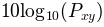for decibels, though `P_{xy}` itself is returned.

References

Bendat & Piersol – Random Data: Analysis and Measurement Procedures, John Wiley & Sons (1986)

Examples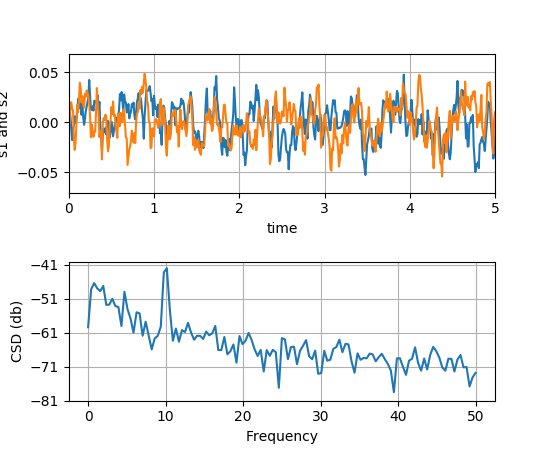`matplotlib.pyplot.``delaxes`(*args)

Remove an axes from the current figure. If ax doesn’t exist, an error will be raised.

`delaxes()`: delete the current axes

`matplotlib.pyplot.``disconnect`(cid)

Disconnect callback id cid

Example usage:

```cid = canvas.mpl_connect('button_press_event', on_press)
#...later
canvas.mpl_disconnect(cid)
```
`matplotlib.pyplot.``draw`()

Redraw the current figure.

This is used to update a figure that has been altered, but not automatically re-drawn. If interactive mode is on (`ion()`), this should be only rarely needed, but there may be ways to modify the state of a figure without marking it as `stale`. Please report these cases as bugs.

A more object-oriented alternative, given any `Figure` instance, `fig`, that was created using a `pyplot` function, is:

```fig.canvas.draw_idle()
```
`matplotlib.pyplot.``errorbar`(x, y, yerr=None, xerr=None, fmt='', ecolor=None, elinewidth=None, capsize=None, barsabove=False, lolims=False, uplims=False, xlolims=False, xuplims=False, errorevery=1, capthick=None, hold=None, data=None, **kwargs)

Plot an errorbar graph.

Plot x versus y with error deltas in yerr and xerr. Vertical errorbars are plotted if yerr is not None. Horizontal errorbars are plotted if xerr is not None.

x, y, xerr, and yerr can all be scalars, which plots a single error bar at x, y.

Parameters:

x : scalar or array-like

y : scalar or array-like

xerr/yerr : scalar or array-like, shape(N,) or shape(2,N), optional

If a scalar number, len(N) array-like object, or a N-element array-like object, errorbars are drawn at +/-value relative to the data. Default is None.

If a sequence of shape 2xN, errorbars are drawn at -row1 and +row2 relative to the data.

fmt : plot format string, optional, default: None

The plot format symbol. If fmt is ‘none’ (case-insensitive), only the errorbars are plotted. This is used for adding errorbars to a bar plot, for example. Default is ‘’, an empty plot format string; properties are then identical to the defaults for `plot()`.

ecolor : mpl color, optional, default: None

A matplotlib color arg which gives the color the errorbar lines; if None, use the color of the line connecting the markers.

elinewidth : scalar, optional, default: None

The linewidth of the errorbar lines. If None, use the linewidth.

capsize : scalar, optional, default: None

The length of the error bar caps in points; if None, it will take the value from `errorbar.capsize` `rcParam`.

capthick : scalar, optional, default: None

An alias kwarg to markeredgewidth (a.k.a. - mew). This setting is a more sensible name for the property that controls the thickness of the error bar cap in points. For backwards compatibility, if mew or markeredgewidth are given, then they will over-ride capthick. This may change in future releases.

barsabove : bool, optional, default: False

if True , will plot the errorbars above the plot symbols. Default is below.

lolims / uplims / xlolims / xuplims : bool, optional, default:None

These arguments can be used to indicate that a value gives only upper/lower limits. In that case a caret symbol is used to indicate this. lims-arguments may be of the same type as xerr and yerr. To use limits with inverted axes, `set_xlim()` or `set_ylim()` must be called before `errorbar()`.

errorevery : positive integer, optional, default:1

subsamples the errorbars. e.g., if errorevery=5, errorbars for every 5-th datapoint will be plotted. The data plot itself still shows all data points.

Returns:

plotline : `Line2D` instance

x, y plot markers and/or line

caplines : list of `Line2D` instances

error bar cap

barlinecols : list of `LineCollection`

horizontal and vertical error ranges.

Other Parameters:

kwargs : All other keyword arguments are passed on to the plot

command for the markers. For example, this code makes big red squares with thick green edges:

```x,y,yerr = rand(3,10)
errorbar(x, y, yerr, marker='s', mfc='red',
mec='green', ms=20, mew=4)
```

where mfc, mec, ms and mew are aliases for the longer property names, markerfacecolor, markeredgecolor, markersize and markeredgewidth.

valid kwargs for the marker properties are

Property Description
`agg_filter` unknown
`alpha` float (0.0 transparent through 1.0 opaque)
`animated` [True | False]
`antialiased` or aa [True | False]
`axes` an `Axes` instance
`clip_box` a `matplotlib.transforms.Bbox` instance
`clip_on` [True | False]
`clip_path` [ (`Path`, `Transform`) | `Patch` | None ]
`color` or c any matplotlib color
`contains` a callable function
`dash_capstyle` [‘butt’ | ‘round’ | ‘projecting’]
`dash_joinstyle` [‘miter’ | ‘round’ | ‘bevel’]
`dashes` sequence of on/off ink in points
`drawstyle` [‘default’ | ‘steps’ | ‘steps-pre’ | ‘steps-mid’ | ‘steps-post’]
`figure` a `matplotlib.figure.Figure` instance
`fillstyle` [‘full’ | ‘left’ | ‘right’ | ‘bottom’ | ‘top’ | ‘none’]
`gid` an id string
`label` string or anything printable with ‘%s’ conversion.
`linestyle` or ls [‘solid’ | ‘dashed’, ‘dashdot’, ‘dotted’ | (offset, on-off-dash-seq) | `'-'` | `'--'` | `'-.'` | `':'` | `'None'` | `' '` | `''`]
`linewidth` or lw float value in points
`marker` `A valid marker style`
`markeredgecolor` or mec any matplotlib color
`markeredgewidth` or mew float value in points
`markerfacecolor` or mfc any matplotlib color
`markerfacecoloralt` or mfcalt any matplotlib color
`markersize` or ms float
`markevery` [None | int | length-2 tuple of int | slice | list/array of int | float | length-2 tuple of float]
`path_effects` unknown
`picker` float distance in points or callable pick function `fn(artist, event)`
`pickradius` float distance in points
`rasterized` [True | False | None]
`sketch_params` unknown
`snap` unknown
`solid_capstyle` [‘butt’ | ‘round’ | ‘projecting’]
`solid_joinstyle` [‘miter’ | ‘round’ | ‘bevel’]
`transform` a `matplotlib.transforms.Transform` instance
`url` a url string
`visible` [True | False]
`xdata` 1D array
`ydata` 1D array
`zorder` any number

Examples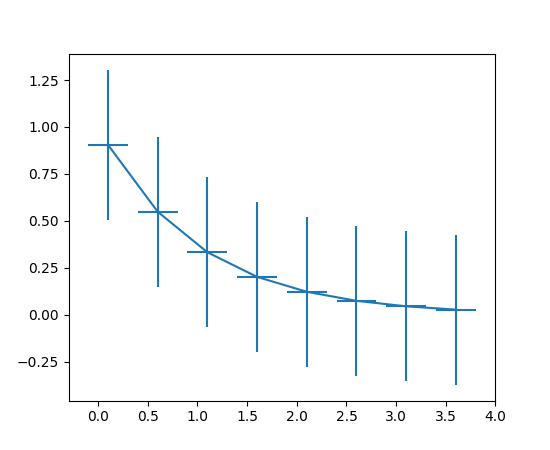Note

In addition to the above described arguments, this function can take a data keyword argument. If such a data argument is given, the following arguments are replaced by data[<arg>]:

• All arguments with the following names: ‘x’, ‘xerr’, ‘y’, ‘yerr’.
`matplotlib.pyplot.``eventplot`(positions, orientation='horizontal', lineoffsets=1, linelengths=1, linewidths=None, colors=None, linestyles='solid', hold=None, data=None, **kwargs)

Plot identical parallel lines at specific positions.

Plot parallel lines at the given positions. positions should be a 1D or 2D array-like object, with each row corresponding to a row or column of lines.

This type of plot is commonly used in neuroscience for representing neural events, where it is commonly called a spike raster, dot raster, or raster plot.

However, it is useful in any situation where you wish to show the timing or position of multiple sets of discrete events, such as the arrival times of people to a business on each day of the month or the date of hurricanes each year of the last century.

orientation : [ ‘horizontal’ | ‘vertical’ ]
‘horizontal’ : the lines will be vertical and arranged in rows ‘vertical’ : lines will be horizontal and arranged in columns
lineoffsets :
A float or array-like containing floats.
linelengths :
A float or array-like containing floats.
linewidths :
A float or array-like containing floats.
colors
must be a sequence of RGBA tuples (e.g., arbitrary color strings, etc, not allowed) or a list of such sequences
linestyles :
[ ‘solid’ | ‘dashed’ | ‘dashdot’ | ‘dotted’ ] or an array of these values

For linelengths, linewidths, colors, and linestyles, if only a single value is given, that value is applied to all lines. If an array-like is given, it must have the same length as positions, and each value will be applied to the corresponding row or column in positions.

Returns a list of `matplotlib.collections.EventCollection` objects that were added.

kwargs are `LineCollection` properties:

Property Description
`agg_filter` unknown
`alpha` float or None
`animated` [True | False]
`antialiased` or antialiaseds Boolean or sequence of booleans
`array` unknown
`axes` an `Axes` instance
`clim` a length 2 sequence of floats
`clip_box` a `matplotlib.transforms.Bbox` instance
`clip_on` [True | False]
`clip_path` [ (`Path`, `Transform`) | `Patch` | None ]
`cmap` a colormap or registered colormap name
`color` matplotlib color arg or sequence of rgba tuples
`contains` a callable function
`edgecolor` or edgecolors matplotlib color spec or sequence of specs
`facecolor` or facecolors matplotlib color spec or sequence of specs
`figure` a `matplotlib.figure.Figure` instance
`gid` an id string
`hatch` [ ‘/’ | ‘\’ | ‘|’ | ‘-‘ | ‘+’ | ‘x’ | ‘o’ | ‘O’ | ‘.’ | ‘*’ ]
`label` string or anything printable with ‘%s’ conversion.
`linestyle` or dashes or linestyles [‘solid’ | ‘dashed’, ‘dashdot’, ‘dotted’ | (offset, on-off-dash-seq) | `'-'` | `'--'` | `'-.'` | `':'` | `'None'` | `' '` | `''`]
`linewidth` or linewidths or lw float or sequence of floats
`norm` unknown
`offset_position` unknown
`offsets` float or sequence of floats
`path_effects` unknown
`paths` unknown
`picker` [None|float|boolean|callable]
`pickradius` unknown
`rasterized` [True | False | None]
`segments` unknown
`sketch_params` unknown
`snap` unknown
`transform` `Transform` instance
`url` a url string
`urls` unknown
`verts` unknown
`visible` [True | False]
`zorder` any number

Example: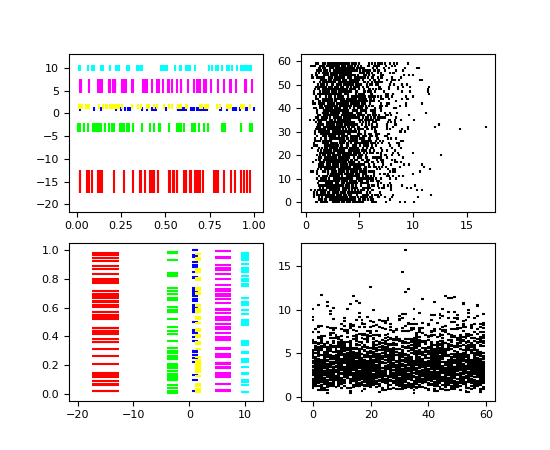Note

In addition to the above described arguments, this function can take a data keyword argument. If such a data argument is given, the following arguments are replaced by data[<arg>]:

• All arguments with the following names: ‘colors’, ‘linelengths’, ‘lineoffsets’, ‘linestyles’, ‘linewidths’, ‘positions’.
`matplotlib.pyplot.``figimage`(*args, **kwargs)

Adds a non-resampled image to the figure.

call signatures:

```figimage(X, **kwargs)
```

adds a non-resampled array X to the figure.

```figimage(X, xo, yo)
```

with pixel offsets xo, yo,

X must be a float array:

• If X is MxN, assume luminance (grayscale)
• If X is MxNx3, assume RGB
• If X is MxNx4, assume RGBA

Optional keyword arguments:

Keyword Description
resize a boolean, True or False. If “True”, then re-size the Figure to match the given image size.
xo or yo An integer, the x and y image offset in pixels
cmap a `matplotlib.colors.Colormap` instance, e.g., cm.jet. If None, default to the rc `image.cmap` value
norm a `matplotlib.colors.Normalize` instance. The default is normalization(). This scales luminance -> 0-1
vmin|vmax are used to scale a luminance image to 0-1. If either is None, the min and max of the luminance values will be used. Note if you pass a norm instance, the settings for vmin and vmax will be ignored.
alpha the alpha blending value, default is None
origin [ ‘upper’ | ‘lower’ ] Indicates where the [0,0] index of the array is in the upper left or lower left corner of the axes. Defaults to the rc image.origin value

figimage complements the axes image (`imshow()`) which will be resampled to fit the current axes. If you want a resampled image to fill the entire figure, you can define an `Axes` with extent [0,0,1,1].

An `matplotlib.image.FigureImage` instance is returned.Additional kwargs are Artist kwargs passed on to `FigureImage`

`matplotlib.pyplot.``figlegend`(handles, labels, loc, **kwargs)

Place a legend in the figure.

labels
a sequence of strings
handles
a sequence of `Line2D` or `Patch` instances
loc
can be a string or an integer specifying the legend location

A `matplotlib.legend.Legend` instance is returned.

Example:

```figlegend( (line1, line2, line3),
('label1', 'label2', 'label3'),
'upper right' )
```
`matplotlib.pyplot.``fignum_exists`(num)
`matplotlib.pyplot.``figtext`(*args, **kwargs)

Add text to figure.

Call signature:

```text(x, y, s, fontdict=None, **kwargs)
```

Add text to figure at location x, y (relative 0-1 coords). See `text()` for the meaning of the other arguments.

kwargs control the `Text` properties:

Property Description
`agg_filter` unknown
`alpha` float (0.0 transparent through 1.0 opaque)
`animated` [True | False]
`axes` an `Axes` instance
`backgroundcolor` any matplotlib color
`bbox` FancyBboxPatch prop dict
`clip_box` a `matplotlib.transforms.Bbox` instance
`clip_on` [True | False]
`clip_path` [ (`Path`, `Transform`) | `Patch` | None ]
`color` any matplotlib color
`contains` a callable function
`family` or fontfamily or fontname or name [FONTNAME | ‘serif’ | ‘sans-serif’ | ‘cursive’ | ‘fantasy’ | ‘monospace’ ]
`figure` a `matplotlib.figure.Figure` instance
`fontproperties` or font_properties a `matplotlib.font_manager.FontProperties` instance
`gid` an id string
`horizontalalignment` or ha [ ‘center’ | ‘right’ | ‘left’ ]
`label` string or anything printable with ‘%s’ conversion.
`linespacing` float (multiple of font size)
`multialignment` [‘left’ | ‘right’ | ‘center’ ]
`path_effects` unknown
`picker` [None|float|boolean|callable]
`position` (x,y)
`rasterized` [True | False | None]
`rotation` [ angle in degrees | ‘vertical’ | ‘horizontal’ ]
`rotation_mode` unknown
`size` or fontsize [size in points | ‘xx-small’ | ‘x-small’ | ‘small’ | ‘medium’ | ‘large’ | ‘x-large’ | ‘xx-large’ ]
`sketch_params` unknown
`snap` unknown
`stretch` or fontstretch [a numeric value in range 0-1000 | ‘ultra-condensed’ | ‘extra-condensed’ | ‘condensed’ | ‘semi-condensed’ | ‘normal’ | ‘semi-expanded’ | ‘expanded’ | ‘extra-expanded’ | ‘ultra-expanded’ ]
`style` or fontstyle [ ‘normal’ | ‘italic’ | ‘oblique’]
`text` string or anything printable with ‘%s’ conversion.
`transform` `Transform` instance
`url` a url string
`usetex` unknown
`variant` or fontvariant [ ‘normal’ | ‘small-caps’ ]
`verticalalignment` or ma or va [ ‘center’ | ‘top’ | ‘bottom’ | ‘baseline’ ]
`visible` [True | False]
`weight` or fontweight [a numeric value in range 0-1000 | ‘ultralight’ | ‘light’ | ‘normal’ | ‘regular’ | ‘book’ | ‘medium’ | ‘roman’ | ‘semibold’ | ‘demibold’ | ‘demi’ | ‘bold’ | ‘heavy’ | ‘extra bold’ | ‘black’ ]
`wrap` unknown
`x` float
`y` float
`zorder` any number
`matplotlib.pyplot.``figure`(num=None, figsize=None, dpi=None, facecolor=None, edgecolor=None, frameon=True, FigureClass=<class 'matplotlib.figure.Figure'>, **kwargs)

Creates a new figure.

Parameters: num : integer or string, optional, default: none If not provided, a new figure will be created, and the figure number will be incremented. The figure objects holds this number in a `number` attribute. If num is provided, and a figure with this id already exists, make it active, and returns a reference to it. If this figure does not exists, create it and returns it. If num is a string, the window title will be set to this figure’s `num`. figsize : tuple of integers, optional, default: None width, height in inches. If not provided, defaults to rc figure.figsize. dpi : integer, optional, default: None resolution of the figure. If not provided, defaults to rc figure.dpi. facecolor : the background color. If not provided, defaults to rc figure.facecolor edgecolor : the border color. If not provided, defaults to rc figure.edgecolor figure : Figure The Figure instance returned will also be passed to new_figure_manager in the backends, which allows to hook custom Figure classes into the pylab interface. Additional kwargs will be passed to the figure init function.

Notes

If you are creating many figures, make sure you explicitly call “close” on the figures you are not using, because this will enable pylab to properly clean up the memory.

rcParams defines the default values, which can be modified in the matplotlibrc file

`matplotlib.pyplot.``fill`(*args, **kwargs)

Plot filled polygons.

Parameters: Returns: args : a variable length argument It allowing for multiple x, y pairs with an optional color format string; see `plot()` for details on the argument parsing. For example, each of the following is legal: ```ax.fill(x, y) ax.fill(x, y, "b") ax.fill(x, y, "b", x, y, "r") ``` An arbitrary number of x, y, color groups can be specified:: ax.fill(x1, y1, ‘g’, x2, y2, ‘r’) a list of `Patch` kwargs : `Polygon` properties

Notes

The same color strings that `plot()` supports are supported by the fill format string.

If you would like to fill below a curve, e.g., shade a region between 0 and y along x, use `fill_between()`

Examples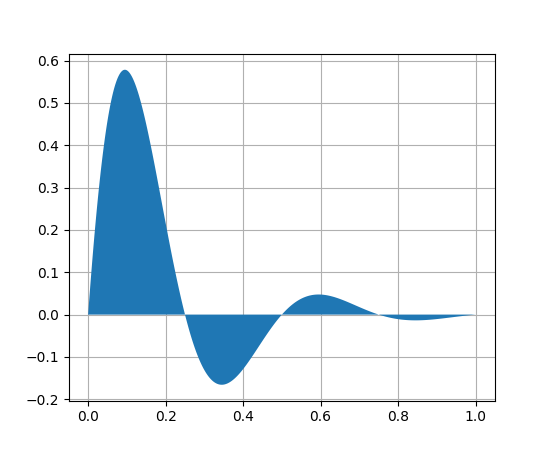Note

In addition to the above described arguments, this function can take a data keyword argument. If such a data argument is given, the following arguments are replaced by data[<arg>]:

• All arguments with the following names: ‘x’, ‘y’.
`matplotlib.pyplot.``fill_between`(x, y1, y2=0, where=None, interpolate=False, step=None, hold=None, data=None, **kwargs)

Make filled polygons between two curves.

Create a `PolyCollection` filling the regions between y1 and y2 where `where==True`

Parameters: x : array An N-length array of the x data y1 : array An N-length array (or scalar) of the y data y2 : array An N-length array (or scalar) of the y data where : array, optional If `None`, default to fill between everywhere. If not `None`, it is an N-length numpy boolean array and the fill will only happen over the regions where `where==True`. interpolate : bool, optional If `True`, interpolate between the two lines to find the precise point of intersection. Otherwise, the start and end points of the filled region will only occur on explicit values in the x array. step : {‘pre’, ‘post’, ‘mid’}, optional If not None, fill with step logic.

In addition to the above described arguments, this function can take a data keyword argument. If such a data argument is given, the following arguments are replaced by data[<arg>]: * All arguments with the following names: ‘where’, ‘x’, ‘y1’, ‘y2’.

Notes

Additional Keyword args passed on to the `PolyCollection`.

kwargs control the `Polygon` properties:

Property Description
`agg_filter` unknown
`alpha` float or None
`animated` [True | False]
`antialiased` or antialiaseds Boolean or sequence of booleans
`array` unknown
`axes` an `Axes` instance
`clim` a length 2 sequence of floats
`clip_box` a `matplotlib.transforms.Bbox` instance
`clip_on` [True | False]
`clip_path` [ (`Path`, `Transform`) | `Patch` | None ]
`cmap` a colormap or registered colormap name
`color` matplotlib color arg or sequence of rgba tuples
`contains` a callable function
`edgecolor` or edgecolors matplotlib color spec or sequence of specs
`facecolor` or facecolors matplotlib color spec or sequence of specs
`figure` a `matplotlib.figure.Figure` instance
`gid` an id string
`hatch` [ ‘/’ | ‘\’ | ‘|’ | ‘-‘ | ‘+’ | ‘x’ | ‘o’ | ‘O’ | ‘.’ | ‘*’ ]
`label` string or anything printable with ‘%s’ conversion.
`linestyle` or dashes or linestyles [‘solid’ | ‘dashed’, ‘dashdot’, ‘dotted’ | (offset, on-off-dash-seq) | `'-'` | `'--'` | `'-.'` | `':'` | `'None'` | `' '` | `''`]
`linewidth` or linewidths or lw float or sequence of floats
`norm` unknown
`offset_position` unknown
`offsets` float or sequence of floats
`path_effects` unknown
`picker` [None|float|boolean|callable]
`pickradius` unknown
`rasterized` [True | False | None]
`sketch_params` unknown
`snap` unknown
`transform` `Transform` instance
`url` a url string
`urls` unknown
`visible` [True | False]
`zorder` any number

Examples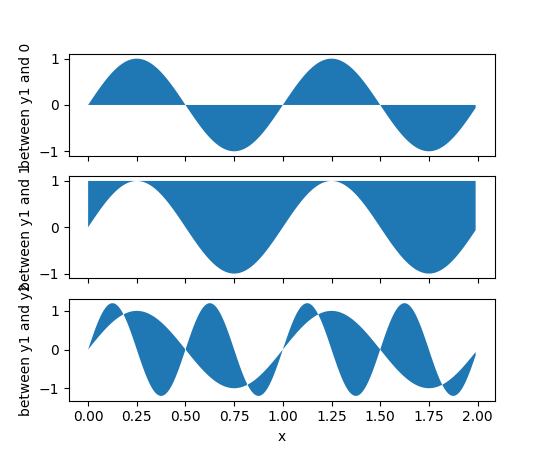(png, pdf)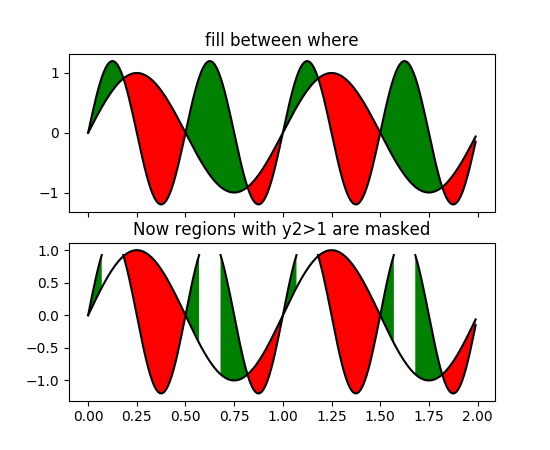(png, pdf)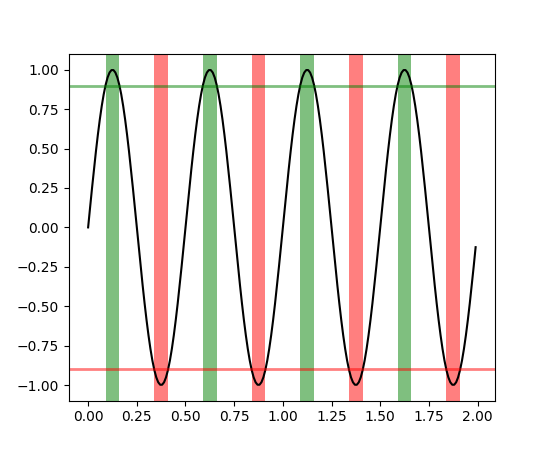(png, pdf)

`matplotlib.pyplot.``fill_betweenx`(y, x1, x2=0, where=None, step=None, hold=None, data=None, **kwargs)

Make filled polygons between two horizontal curves.

Create a `PolyCollection` filling the regions between x1 and x2 where `where==True`

Parameters: y : array An N-length array of the y data x1 : array An N-length array (or scalar) of the x data x2 : array, optional An N-length array (or scalar) of the x data where : array, optional If None, default to fill between everywhere. If not None, it is a N length numpy boolean array and the fill will only happen over the regions where `where==True` step : {‘pre’, ‘post’, ‘mid’}, optional If not None, fill with step logic.

In addition to the above described arguments, this function can take a data keyword argument. If such a data argument is given, the following arguments are replaced by data[<arg>]: * All arguments with the following names: ‘where’, ‘x1’, ‘x2’, ‘y’.

Notes

keyword args passed on to the
`PolyCollection`

kwargs control the `Polygon` properties:

Property Description
`agg_filter` unknown
`alpha` float or None
`animated` [True | False]
`antialiased` or antialiaseds Boolean or sequence of booleans
`array` unknown
`axes` an `Axes` instance
`clim` a length 2 sequence of floats
`clip_box` a `matplotlib.transforms.Bbox` instance
`clip_on` [True | False]
`clip_path` [ (`Path`, `Transform`) | `Patch` | None ]
`cmap` a colormap or registered colormap name
`color` matplotlib color arg or sequence of rgba tuples
`contains` a callable function
`edgecolor` or edgecolors matplotlib color spec or sequence of specs
`facecolor` or facecolors matplotlib color spec or sequence of specs
`figure` a `matplotlib.figure.Figure` instance
`gid` an id string
`hatch` [ ‘/’ | ‘\’ | ‘|’ | ‘-‘ | ‘+’ | ‘x’ | ‘o’ | ‘O’ | ‘.’ | ‘*’ ]
`label` string or anything printable with ‘%s’ conversion.
`linestyle` or dashes or linestyles [‘solid’ | ‘dashed’, ‘dashdot’, ‘dotted’ | (offset, on-off-dash-seq) | `'-'` | `'--'` | `'-.'` | `':'` | `'None'` | `' '` | `''`]
`linewidth` or linewidths or lw float or sequence of floats
`norm` unknown
`offset_position` unknown
`offsets` float or sequence of floats
`path_effects` unknown
`picker` [None|float|boolean|callable]
`pickradius` unknown
`rasterized` [True | False | None]
`sketch_params` unknown
`snap` unknown
`transform` `Transform` instance
`url` a url string
`urls` unknown
`visible` [True | False]
`zorder` any number

Examples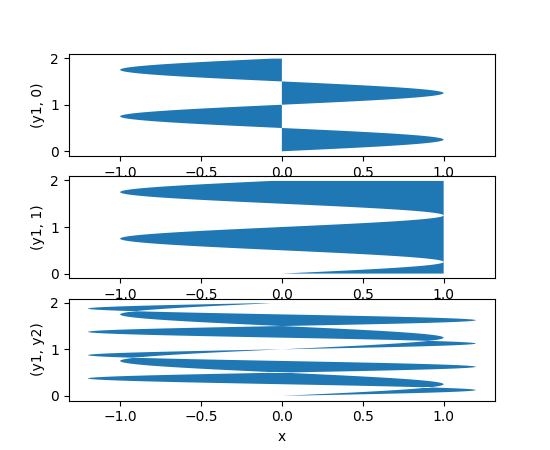(png, pdf)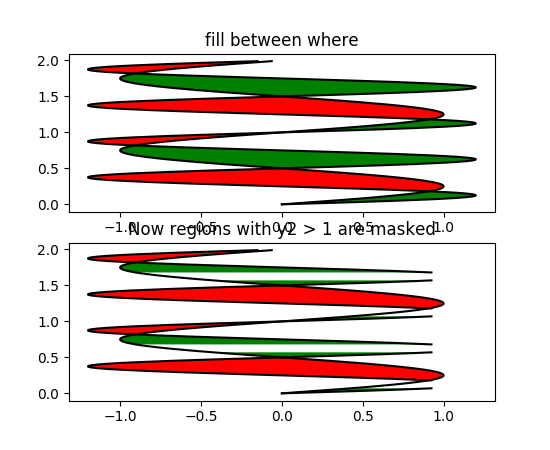(png, pdf)

`matplotlib.pyplot.``findobj`(o=None, match=None, include_self=True)

Find artist objects.

Recursively find all `Artist` instances contained in self.

match can be

• None: return all objects contained in artist.
• function with signature `boolean = match(artist)` used to filter matches
• class instance: e.g., Line2D. Only return artists of class type.

If include_self is True (default), include self in the list to be checked for a match.

`matplotlib.pyplot.``flag`()

set the default colormap to flag and apply to current image if any. See help(colormaps) for more information

`matplotlib.pyplot.``gca`(**kwargs)

Get the current `Axes` instance on the current figure matching the given keyword args, or create one.

`matplotlib.figure.Figure.gca`
The figure’s gca method.

Examples

To get the current polar axes on the current figure:

```plt.gca(projection='polar')
```

If the current axes doesn’t exist, or isn’t a polar one, the appropriate axes will be created and then returned.

`matplotlib.pyplot.``gcf`()

Get a reference to the current figure.

`matplotlib.pyplot.``gci`()

Get the current colorable artist. Specifically, returns the current `ScalarMappable` instance (image or patch collection), or None if no images or patch collections have been defined. The commands `imshow()` and `figimage()` create `Image` instances, and the commands `pcolor()` and `scatter()` create `Collection` instances. The current image is an attribute of the current axes, or the nearest earlier axes in the current figure that contains an image.

`matplotlib.pyplot.``get_current_fig_manager`()
`matplotlib.pyplot.``get_figlabels`()

Return a list of existing figure labels.

`matplotlib.pyplot.``get_fignums`()

Return a list of existing figure numbers.

`matplotlib.pyplot.``get_plot_commands`()

Get a sorted list of all of the plotting commands.

`matplotlib.pyplot.``ginput`(*args, **kwargs)

Blocking call to interact with the figure.

This will wait for n clicks from the user and return a list of the coordinates of each click.

If timeout is zero or negative, does not timeout.

If n is zero or negative, accumulate clicks until a middle click (or potentially both mouse buttons at once) terminates the input.

Right clicking cancels last input.

The buttons used for the various actions (adding points, removing points, terminating the inputs) can be overriden via the arguments mouse_add, mouse_pop and mouse_stop, that give the associated mouse button: 1 for left, 2 for middle, 3 for right.

The keyboard can also be used to select points in case your mouse does not have one or more of the buttons. The delete and backspace keys act like right clicking (i.e., remove last point), the enter key terminates input and any other key (not already used by the window manager) selects a point.

`matplotlib.pyplot.``gray`()

set the default colormap to gray and apply to current image if any. See help(colormaps) for more information

`matplotlib.pyplot.``grid`(b=None, which='major', axis='both', **kwargs)

Turn the axes grids on or off.

Set the axes grids on or off; b is a boolean. (For MATLAB compatibility, b may also be a string, ‘on’ or ‘off’.)

If b is None and `len(kwargs)==0`, toggle the grid state. If kwargs are supplied, it is assumed that you want a grid and b is thus set to True.

which can be ‘major’ (default), ‘minor’, or ‘both’ to control whether major tick grids, minor tick grids, or both are affected.

axis can be ‘both’ (default), ‘x’, or ‘y’ to control which set of gridlines are drawn.

kwargs are used to set the grid line properties, e.g.,:

```ax.grid(color='r', linestyle='-', linewidth=2)
```

Valid `Line2D` kwargs are

Property Description
`agg_filter` unknown
`alpha` float (0.0 transparent through 1.0 opaque)
`animated` [True | False]
`antialiased` or aa [True | False]
`axes` an `Axes` instance
`clip_box` a `matplotlib.transforms.Bbox` instance
`clip_on` [True | False]
`clip_path` [ (`Path`, `Transform`) | `Patch` | None ]
`color` or c any matplotlib color
`contains` a callable function
`dash_capstyle` [‘butt’ | ‘round’ | ‘projecting’]
`dash_joinstyle` [‘miter’ | ‘round’ | ‘bevel’]
`dashes` sequence of on/off ink in points
`drawstyle` [‘default’ | ‘steps’ | ‘steps-pre’ | ‘steps-mid’ | ‘steps-post’]
`figure` a `matplotlib.figure.Figure` instance
`fillstyle` [‘full’ | ‘left’ | ‘right’ | ‘bottom’ | ‘top’ | ‘none’]
`gid` an id string
`label` string or anything printable with ‘%s’ conversion.
`linestyle` or ls [‘solid’ | ‘dashed’, ‘dashdot’, ‘dotted’ | (offset, on-off-dash-seq) | `'-'` | `'--'` | `'-.'` | `':'` | `'None'` | `' '` | `''`]
`linewidth` or lw float value in points
`marker` `A valid marker style`
`markeredgecolor` or mec any matplotlib color
`markeredgewidth` or mew float value in points
`markerfacecolor` or mfc any matplotlib color
`markerfacecoloralt` or mfcalt any matplotlib color
`markersize` or ms float
`markevery` [None | int | length-2 tuple of int | slice | list/array of int | float | length-2 tuple of float]
`path_effects` unknown
`picker` float distance in points or callable pick function `fn(artist, event)`
`pickradius` float distance in points
`rasterized` [True | False | None]
`sketch_params` unknown
`snap` unknown
`solid_capstyle` [‘butt’ | ‘round’ | ‘projecting’]
`solid_joinstyle` [‘miter’ | ‘round’ | ‘bevel’]
`transform` a `matplotlib.transforms.Transform` instance
`url` a url string
`visible` [True | False]
`xdata` 1D array
`ydata` 1D array
`zorder` any number
`matplotlib.pyplot.``hexbin`(x, y, C=None, gridsize=100, bins=None, xscale='linear', yscale='linear', extent=None, cmap=None, norm=None, vmin=None, vmax=None, alpha=None, linewidths=None, edgecolors='none', reduce_C_function=<function mean>, mincnt=None, marginals=False, hold=None, data=None, **kwargs)

Make a hexagonal binning plot.

Make a hexagonal binning plot of x versus y, where x, y are 1-D sequences of the same length, N. If C is None (the default), this is a histogram of the number of occurences of the observations at (x[i],y[i]).

If C is specified, it specifies values at the coordinate (x[i],y[i]). These values are accumulated for each hexagonal bin and then reduced according to reduce_C_function, which defaults to numpy’s mean function (np.mean). (If C is specified, it must also be a 1-D sequence of the same length as x and y.)

Parameters: Returns: x, y : array or masked array C : array or masked array, optional, default is None gridsize : int or (int, int), optional, default is 100 The number of hexagons in the x-direction, default is 100. The corresponding number of hexagons in the y-direction is chosen such that the hexagons are approximately regular. Alternatively, gridsize can be a tuple with two elements specifying the number of hexagons in the x-direction and the y-direction. bins : {‘log’} or int or sequence, optional, default is None If None, no binning is applied; the color of each hexagon directly corresponds to its count value. If ‘log’, use a logarithmic scale for the color map. Internally,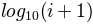is used to determine the hexagon color. If an integer, divide the counts in the specified number of bins, and color the hexagons accordingly. If a sequence of values, the values of the lower bound of the bins to be used. xscale : {‘linear’, ‘log’}, optional, default is ‘linear’ Use a linear or log10 scale on the horizontal axis. yscale : {‘linear’, ‘log’}, optional, default is ‘linear’ Use a linear or log10 scale on the vertical axis. mincnt : int > 0, optional, default is None If not None, only display cells with more than mincnt number of points in the cell marginals : bool, optional, default is False if marginals is True, plot the marginal density as colormapped rectagles along the bottom of the x-axis and left of the y-axis extent : scalar, optional, default is None The limits of the bins. The default assigns the limits based on gridsize, x, y, xscale and yscale. If xscale or yscale is set to ‘log’, the limits are expected to be the exponent for a power of 10. E.g. for x-limits of 1 and 50 in ‘linear’ scale and y-limits of 10 and 1000 in ‘log’ scale, enter (1, 50, 1, 3). Order of scalars is (left, right, bottom, top). object a `PolyCollection` instance; use `get_array()` on this `PolyCollection` to get the counts in each hexagon. If marginals is True, horizontal bar and vertical bar (both PolyCollections) will be attached to the return collection as attributes hbar and vbar. cmap : object, optional, default is None a `matplotlib.colors.Colormap` instance. If None, defaults to rc `image.cmap`. norm : object, optional, default is None `matplotlib.colors.Normalize` instance is used to scale luminance data to 0,1. vmin, vmax : scalar, optional, default is None vmin and vmax are used in conjunction with norm to normalize luminance data. If None, the min and max of the color array C are used. Note if you pass a norm instance your settings for vmin and vmax will be ignored. alpha : scalar between 0 and 1, optional, default is None the alpha value for the patches linewidths : scalar, optional, default is None If None, defaults to 1.0. edgecolors : {‘none’} or mpl color, optional, default is ‘none’ If ‘none’, draws the edges in the same color as the fill color. This is the default, as it avoids unsightly unpainted pixels between the hexagons. If None, draws outlines in the default color. If a matplotlib color arg, draws outlines in the specified color.

Notes

The standard descriptions of all the `Collection` parameters:

Property Description
`agg_filter` unknown
`alpha` float or None
`animated` [True | False]
`antialiased` or antialiaseds Boolean or sequence of booleans
`array` unknown
`axes` an `Axes` instance
`clim` a length 2 sequence of floats
`clip_box` a `matplotlib.transforms.Bbox` instance
`clip_on` [True | False]
`clip_path` [ (`Path`, `Transform`) | `Patch` | None ]
`cmap` a colormap or registered colormap name
`color` matplotlib color arg or sequence of rgba tuples
`contains` a callable function
`edgecolor` or edgecolors matplotlib color spec or sequence of specs
`facecolor` or facecolors matplotlib color spec or sequence of specs
`figure` a `matplotlib.figure.Figure` instance
`gid` an id string
`hatch` [ ‘/’ | ‘\’ | ‘|’ | ‘-‘ | ‘+’ | ‘x’ | ‘o’ | ‘O’ | ‘.’ | ‘*’ ]
`label` string or anything printable with ‘%s’ conversion.
`linestyle` or dashes or linestyles [‘solid’ | ‘dashed’, ‘dashdot’, ‘dotted’ | (offset, on-off-dash-seq) | `'-'` | `'--'` | `'-.'` | `':'` | `'None'` | `' '` | `''`]
`linewidth` or linewidths or lw float or sequence of floats
`norm` unknown
`offset_position` unknown
`offsets` float or sequence of floats
`path_effects` unknown
`picker` [None|float|boolean|callable]
`pickradius` unknown
`rasterized` [True | False | None]
`sketch_params` unknown
`snap` unknown
`transform` `Transform` instance
`url` a url string
`urls` unknown
`visible` [True | False]
`zorder` any number

Note

In addition to the above described arguments, this function can take a data keyword argument. If such a data argument is given, the following arguments are replaced by data[<arg>]:

• All arguments with the following names: ‘x’, ‘y’.

Examples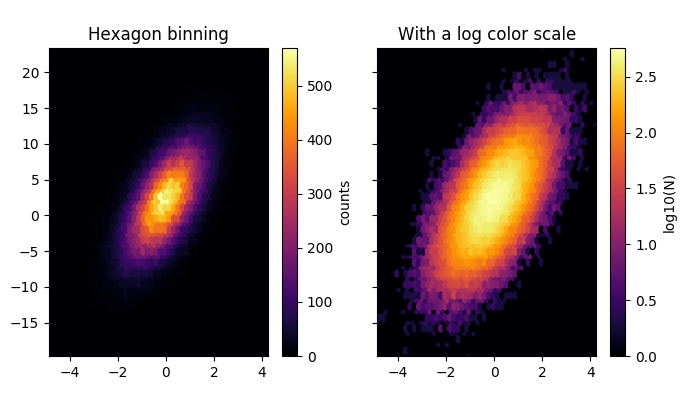`matplotlib.pyplot.``hist`(x, bins=None, range=None, normed=False, weights=None, cumulative=False, bottom=None, histtype='bar', align='mid', orientation='vertical', rwidth=None, log=False, color=None, label=None, stacked=False, hold=None, data=None, **kwargs)

Plot a histogram.

Compute and draw the histogram of x. The return value is a tuple (n, bins, patches) or ([n0, n1, ...], bins, [patches0, patches1,...]) if the input contains multiple data.

Multiple data can be provided via x as a list of datasets of potentially different length ([x0, x1, ...]), or as a 2-D ndarray in which each column is a dataset. Note that the ndarray form is transposed relative to the list form.

Masked arrays are not supported at present.

Parameters: Returns: x : (n,) array or sequence of (n,) arrays Input values, this takes either a single array or a sequency of arrays which are not required to be of the same length bins : integer or array_like or ‘auto’, optional If an integer is given, `bins + 1` bin edges are returned, consistently with `numpy.histogram()` for numpy version >= 1.3. Unequally spaced bins are supported if `bins` is a sequence. If Numpy 1.11 is installed, may also be `'auto'`. Default is taken from the rcParam `hist.bins`. range : tuple or None, optional The lower and upper range of the bins. Lower and upper outliers are ignored. If not provided, `range` is (x.min(), x.max()). Range has no effect if `bins` is a sequence. If `bins` is a sequence or `range` is specified, autoscaling is based on the specified bin range instead of the range of x. Default is `None` normed : boolean, optional If `True`, the first element of the return tuple will be the counts normalized to form a probability density, i.e., `n/(len(x)`dbin)`, i.e., the integral of the histogram will sum to 1. If stacked is also True, the sum of the histograms is normalized to 1. Default is `False` weights : (n, ) array_like or None, optional An array of weights, of the same shape as `x`. Each value in `x` only contributes its associated weight towards the bin count (instead of 1). If `normed` is True, the weights are normalized, so that the integral of the density over the range remains 1. Default is `None` cumulative : boolean, optional If `True`, then a histogram is computed where each bin gives the counts in that bin plus all bins for smaller values. The last bin gives the total number of datapoints. If `normed` is also `True` then the histogram is normalized such that the last bin equals 1. If `cumulative` evaluates to less than 0 (e.g., -1), the direction of accumulation is reversed. In this case, if `normed` is also `True`, then the histogram is normalized such that the first bin equals 1. Default is `False` bottom : array_like, scalar, or None Location of the bottom baseline of each bin. If a scalar, the base line for each bin is shifted by the same amount. If an array, each bin is shifted independently and the length of bottom must match the number of bins. If None, defaults to 0. Default is `None` histtype : {‘bar’, ‘barstacked’, ‘step’, ‘stepfilled’}, optional The type of histogram to draw. ‘bar’ is a traditional bar-type histogram. If multiple data are given the bars are aranged side by side. ‘barstacked’ is a bar-type histogram where multiple data are stacked on top of each other. ‘step’ generates a lineplot that is by default unfilled. ‘stepfilled’ generates a lineplot that is by default filled. Default is ‘bar’ align : {‘left’, ‘mid’, ‘right’}, optional Controls how the histogram is plotted. ‘left’: bars are centered on the left bin edges. ‘mid’: bars are centered between the bin edges. ‘right’: bars are centered on the right bin edges. Default is ‘mid’ orientation : {‘horizontal’, ‘vertical’}, optional If ‘horizontal’, `barh` will be used for bar-type histograms and the bottom kwarg will be the left edges. rwidth : scalar or None, optional The relative width of the bars as a fraction of the bin width. If `None`, automatically compute the width. Ignored if `histtype` is ‘step’ or ‘stepfilled’. Default is `None` log : boolean, optional If `True`, the histogram axis will be set to a log scale. If `log` is `True` and `x` is a 1D array, empty bins will be filtered out and only the non-empty (`n`, `bins`, `patches`) will be returned. Default is `False` color : color or array_like of colors or None, optional Color spec or sequence of color specs, one per dataset. Default (`None`) uses the standard line color sequence. Default is `None` label : string or None, optional String, or sequence of strings to match multiple datasets. Bar charts yield multiple patches per dataset, but only the first gets the label, so that the legend command will work as expected. default is `None` stacked : boolean, optional If `True`, multiple data are stacked on top of each other If `False` multiple data are aranged side by side if histtype is ‘bar’ or on top of each other if histtype is ‘step’ Default is `False` n : array or list of arrays The values of the histogram bins. See normed and weights for a description of the possible semantics. If input x is an array, then this is an array of length nbins. If input is a sequence arrays `[data1, data2,..]`, then this is a list of arrays with the values of the histograms for each of the arrays in the same order. bins : array The edges of the bins. Length nbins + 1 (nbins left edges and right edge of last bin). Always a single array even when multiple data sets are passed in. patches : list or list of lists Silent list of individual patches used to create the histogram or list of such list if multiple input datasets. kwargs : `Patch` properties

`hist2d`
2D histograms

Notes

Until numpy release 1.5, the underlying numpy histogram function was incorrect with `normed`=`True` if bin sizes were unequal. MPL inherited that error. It is now corrected within MPL when using earlier numpy versions.

Examples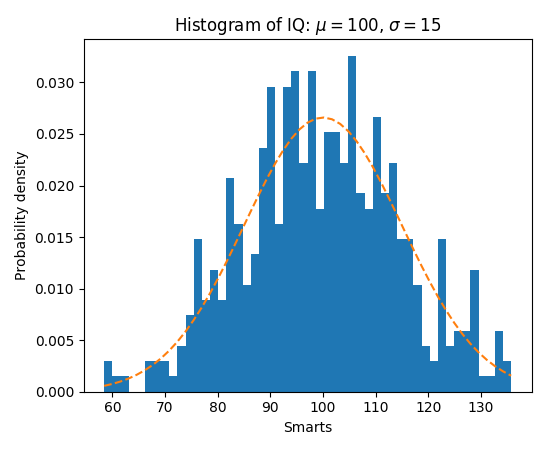Note

In addition to the above described arguments, this function can take a data keyword argument. If such a data argument is given, the following arguments are replaced by data[<arg>]:

• All arguments with the following names: ‘weights’, ‘x’.
`matplotlib.pyplot.``hist2d`(x, y, bins=10, range=None, normed=False, weights=None, cmin=None, cmax=None, hold=None, data=None, **kwargs)

Make a 2D histogram plot.

Parameters: Returns: x, y: array_like, shape (n, ) Input values bins: [None | int | [int, int] | array_like | [array, array]] The bin specification: If int, the number of bins for the two dimensions (nx=ny=bins). If [int, int], the number of bins in each dimension (nx, ny = bins). If array_like, the bin edges for the two dimensions (x_edges=y_edges=bins). If [array, array], the bin edges in each dimension (x_edges, y_edges = bins). The default value is 10. range : array_like shape(2, 2), optional, default: None The leftmost and rightmost edges of the bins along each dimension (if not specified explicitly in the bins parameters): [[xmin, xmax], [ymin, ymax]]. All values outside of this range will be considered outliers and not tallied in the histogram. normed : boolean, optional, default: False Normalize histogram. weights : array_like, shape (n, ), optional, default: None An array of values w_i weighing each sample (x_i, y_i). cmin : scalar, optional, default: None All bins that has count less than cmin will not be displayed and these count values in the return value count histogram will also be set to nan upon return cmax : scalar, optional, default: None All bins that has count more than cmax will not be displayed (set to none before passing to imshow) and these count values in the return value count histogram will also be set to nan upon return The return value is `(counts, xedges, yedges, Image)`. cmap : {Colormap, string}, optional A `matplotlib.colors.Colormap` instance. If not set, use rc settings. norm : Normalize, optional A `matplotlib.colors.Normalize` instance is used to scale luminance data to `[0, 1]`. If not set, defaults to `Normalize()`. vmin/vmax : {None, scalar}, optional Arguments passed to the `Normalize` instance. alpha : `0 <= scalar <= 1` or `None`, optional The alpha blending value.

`hist`
1D histogram

Notes

Rendering the histogram with a logarithmic color scale is accomplished by passing a `colors.LogNorm` instance to the norm keyword argument. Likewise, power-law normalization (similar in effect to gamma correction) can be accomplished with `colors.PowerNorm`.

Examples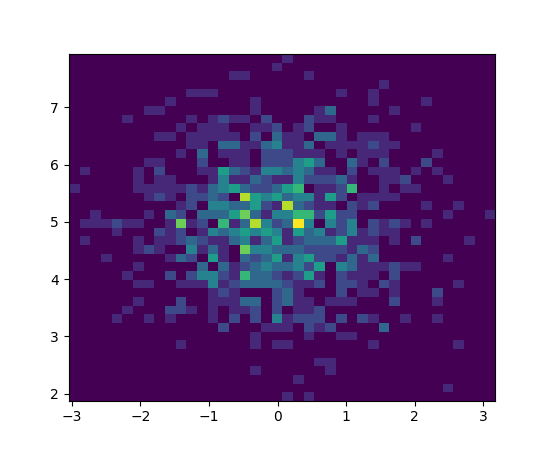Note

In addition to the above described arguments, this function can take a data keyword argument. If such a data argument is given, the following arguments are replaced by data[<arg>]:

• All arguments with the following names: ‘weights’, ‘x’, ‘y’.
`matplotlib.pyplot.``hlines`(y, xmin, xmax, colors='k', linestyles='solid', label='', hold=None, data=None, **kwargs)

Plot horizontal lines at each `y` from `xmin` to `xmax`.

Parameters: Returns: y : scalar or sequence of scalar y-indexes where to plot the lines. xmin, xmax : scalar or 1D array_like Respective beginning and end of each line. If scalars are provided, all lines will have same length. colors : array_like of colors, optional, default: ‘k’ linestyles : [‘solid’ | ‘dashed’ | ‘dashdot’ | ‘dotted’], optional label : string, optional, default: ‘’ lines : `LineCollection` kwargs : `LineCollection` properties.

`vlines`
vertical lines

Examples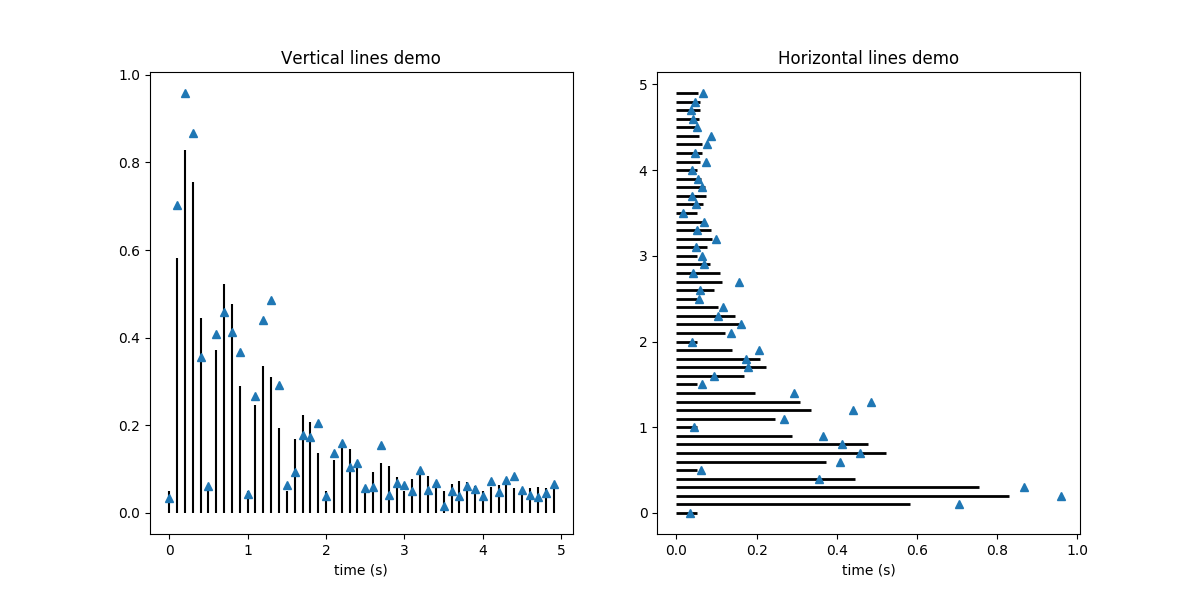Note

In addition to the above described arguments, this function can take a data keyword argument. If such a data argument is given, the following arguments are replaced by data[<arg>]:

• All arguments with the following names: ‘xmax’, ‘xmin’, ‘y’.
`matplotlib.pyplot.``hold`(b=None)

Deprecated since version 2.0: pyplot.hold is deprecated. Future behavior will be consistent with the long-time default: plot commands add elements without first clearing the Axes and/or Figure.

Set the hold state. If b is None (default), toggle the hold state, else set the hold state to boolean value b:

```hold()      # toggle hold
hold(True)  # hold is on
hold(False) # hold is off
```

When hold is True, subsequent plot commands will add elements to the current axes. When hold is False, the current axes and figure will be cleared on the next plot command.

`matplotlib.pyplot.``hot`()

set the default colormap to hot and apply to current image if any. See help(colormaps) for more information

`matplotlib.pyplot.``hsv`()

set the default colormap to hsv and apply to current image if any. See help(colormaps) for more information

`matplotlib.pyplot.``imread`(*args, **kwargs)

Read an image from a file into an array.

fname may be a string path, a valid URL, or a Python file-like object. If using a file object, it must be opened in binary mode.

If format is provided, will try to read file of that type, otherwise the format is deduced from the filename. If nothing can be deduced, PNG is tried.

Return value is a `numpy.array`. For grayscale images, the return array is MxN. For RGB images, the return value is MxNx3. For RGBA images the return value is MxNx4.

matplotlib can only read PNGs natively, but if PIL is installed, it will use it to load the image and return an array (if possible) which can be used with `imshow()`. Note, URL strings may not be compatible with PIL. Check the PIL documentation for more information.

`matplotlib.pyplot.``imsave`(*args, **kwargs)

Save an array as in image file.

The output formats available depend on the backend being used.

Arguments:
fname:
A string containing a path to a filename, or a Python file-like object. If format is None and fname is a string, the output format is deduced from the extension of the filename.
arr:
An MxN (luminance), MxNx3 (RGB) or MxNx4 (RGBA) array.
Keyword arguments:
vmin/vmax: [ None | scalar ]
vmin and vmax set the color scaling for the image by fixing the values that map to the colormap color limits. If either vmin or vmax is None, that limit is determined from the arr min/max value.
cmap:
cmap is a colors.Colormap instance, e.g., cm.jet. If None, default to the rc image.cmap value.
format:
One of the file extensions supported by the active backend. Most backends support png, pdf, ps, eps and svg.
origin
[ ‘upper’ | ‘lower’ ] Indicates where the [0,0] index of the array is in the upper left or lower left corner of the axes. Defaults to the rc image.origin value.
dpi
The DPI to store in the metadata of the file. This does not affect the resolution of the output image.
`matplotlib.pyplot.``imshow`(X, cmap=None, norm=None, aspect=None, interpolation=None, alpha=None, vmin=None, vmax=None, origin=None, extent=None, shape=None, filternorm=1, filterrad=4.0, imlim=None, resample=None, url=None, hold=None, data=None, **kwargs)

Display an image on the axes.

Parameters: Returns: X : array_like, shape (n, m) or (n, m, 3) or (n, m, 4) Display the image in `X` to current axes. `X` may be an array or a PIL image. If `X` is an array, it can have the following shapes and types: MxN – values to be mapped (float or int) MxNx3 – RGB (float or uint8) MxNx4 – RGBA (float or uint8) The value for each component of MxNx3 and MxNx4 float arrays should be in the range 0.0 to 1.0. MxN arrays are mapped to colors based on the `norm` (mapping scalar to scalar) and the `cmap` (mapping the normed scalar to a color). cmap : `Colormap`, optional, default: None If None, default to rc `image.cmap` value. `cmap` is ignored if `X` is 3-D, directly specifying RGB(A) values. aspect : [‘auto’ | ‘equal’ | scalar], optional, default: None If ‘auto’, changes the image aspect ratio to match that of the axes. If ‘equal’, and `extent` is None, changes the axes aspect ratio to match that of the image. If `extent` is not `None`, the axes aspect ratio is changed to match that of the extent. If None, default to rc `image.aspect` value. interpolation : string, optional, default: None Acceptable values are ‘none’, ‘nearest’, ‘bilinear’, ‘bicubic’, ‘spline16’, ‘spline36’, ‘hanning’, ‘hamming’, ‘hermite’, ‘kaiser’, ‘quadric’, ‘catrom’, ‘gaussian’, ‘bessel’, ‘mitchell’, ‘sinc’, ‘lanczos’ If `interpolation` is None, default to rc `image.interpolation`. See also the `filternorm` and `filterrad` parameters. If `interpolation` is ‘none’, then no interpolation is performed on the Agg, ps and pdf backends. Other backends will fall back to ‘nearest’. norm : `Normalize`, optional, default: None A `Normalize` instance is used to scale a 2-D float `X` input to the (0, 1) range for input to the `cmap`. If `norm` is None, use the default func:`normalize`. If `norm` is an instance of `NoNorm`, `X` must be an array of integers that index directly into the lookup table of the `cmap`. vmin, vmax : scalar, optional, default: None `vmin` and `vmax` are used in conjunction with norm to normalize luminance data. Note if you pass a `norm` instance, your settings for `vmin` and `vmax` will be ignored. alpha : scalar, optional, default: None The alpha blending value, between 0 (transparent) and 1 (opaque) origin : [‘upper’ | ‘lower’], optional, default: None Place the [0,0] index of the array in the upper left or lower left corner of the axes. If None, default to rc `image.origin`. extent : scalars (left, right, bottom, top), optional, default: None The location, in data-coordinates, of the lower-left and upper-right corners. If `None`, the image is positioned such that the pixel centers fall on zero-based (row, column) indices. shape : scalars (columns, rows), optional, default: None For raw buffer images filternorm : scalar, optional, default: 1 A parameter for the antigrain image resize filter. From the antigrain documentation, if `filternorm` = 1, the filter normalizes integer values and corrects the rounding errors. It doesn’t do anything with the source floating point values, it corrects only integers according to the rule of 1.0 which means that any sum of pixel weights must be equal to 1.0. So, the filter function must produce a graph of the proper shape. filterrad : scalar, optional, default: 4.0 The filter radius for filters that have a radius parameter, i.e. when interpolation is one of: ‘sinc’, ‘lanczos’ or ‘blackman’ image : `AxesImage` kwargs : `Artist` properties.

`matshow`
Plot a matrix or an array as an image.

Notes

Unless extent is used, pixel centers will be located at integer coordinates. In other words: the origin will coincide with the center of pixel (0, 0).

Examples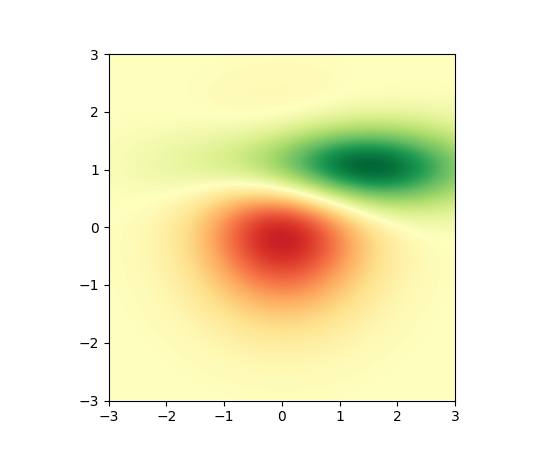Note

In addition to the above described arguments, this function can take a data keyword argument. If such a data argument is given, the following arguments are replaced by data[<arg>]:

• All positional and all keyword arguments.
`matplotlib.pyplot.``inferno`()

set the default colormap to inferno and apply to current image if any. See help(colormaps) for more information

`matplotlib.pyplot.``install_repl_displayhook`()

Install a repl display hook so that any stale figure are automatically redrawn when control is returned to the repl.

This works with IPython terminals and kernels, as well as vanilla python shells.

`matplotlib.pyplot.``ioff`()

Turn interactive mode off.

`matplotlib.pyplot.``ion`()

Turn interactive mode on.

`matplotlib.pyplot.``ishold`()

Deprecated since version 2.0: pyplot.hold is deprecated. Future behavior will be consistent with the long-time default: plot commands add elements without first clearing the Axes and/or Figure.

Return the hold status of the current axes.

`matplotlib.pyplot.``isinteractive`()

Return status of interactive mode.

`matplotlib.pyplot.``jet`()

set the default colormap to jet and apply to current image if any. See help(colormaps) for more information

`matplotlib.pyplot.``legend`(*args, **kwargs)

Places a legend on the axes.

To make a legend for lines which already exist on the axes (via plot for instance), simply call this function with an iterable of strings, one for each legend item. For example:

```ax.plot([1, 2, 3])
ax.legend(['A simple line'])
```

However, in order to keep the “label” and the legend element instance together, it is preferable to specify the label either at artist creation, or by calling the `set_label()` method on the artist:

```line, = ax.plot([1, 2, 3], label='Inline label')
# Overwrite the label by calling the method.
line.set_label('Label via method')
ax.legend()
```

Specific lines can be excluded from the automatic legend element selection by defining a label starting with an underscore. This is default for all artists, so calling `legend()` without any arguments and without setting the labels manually will result in no legend being drawn.

For full control of which artists have a legend entry, it is possible to pass an iterable of legend artists followed by an iterable of legend labels respectively:

```legend((line1, line2, line3), ('label1', 'label2', 'label3'))
```
Parameters:

loc : int or string or pair of floats, default: ‘upper right’

The location of the legend. Possible codes are:

Location String Location Code
‘best’ 0
‘upper right’ 1
‘upper left’ 2
‘lower left’ 3
‘lower right’ 4
‘right’ 5
‘center left’ 6
‘center right’ 7
‘lower center’ 8
‘upper center’ 9
‘center’ 10

Alternatively can be a 2-tuple giving `x, y` of the lower-left corner of the legend in axes coordinates (in which case `bbox_to_anchor` will be ignored).

bbox_to_anchor : `matplotlib.transforms.BboxBase` instance or tuple of floats

Specify any arbitrary location for the legend in `bbox_transform` coordinates (default Axes coordinates).

For example, to put the legend’s upper right hand corner in the center of the axes the following keywords can be used:

```loc='upper right', bbox_to_anchor=(0.5, 0.5)
```

ncol : integer

The number of columns that the legend has. Default is 1.

prop : None or `matplotlib.font_manager.FontProperties` or dict

The font properties of the legend. If None (default), the current `matplotlib.rcParams` will be used.

fontsize : int or float or {‘xx-small’, ‘x-small’, ‘small’, ‘medium’, ‘large’, ‘x-large’, ‘xx-large’}

Controls the font size of the legend. If the value is numeric the size will be the absolute font size in points. String values are relative to the current default font size. This argument is only used if `prop` is not specified.

numpoints : None or int

The number of marker points in the legend when creating a legend entry for a line/`matplotlib.lines.Line2D`. Default is `None` which will take the value from the `legend.numpoints` `rcParam`.

scatterpoints : None or int

The number of marker points in the legend when creating a legend entry for a scatter plot/ `matplotlib.collections.PathCollection`. Default is `None` which will take the value from the `legend.scatterpoints` `rcParam`.

scatteryoffsets : iterable of floats

The vertical offset (relative to the font size) for the markers created for a scatter plot legend entry. 0.0 is at the base the legend text, and 1.0 is at the top. To draw all markers at the same height, set to `[0.5]`. Default `[0.375, 0.5, 0.3125]`.

markerscale : None or int or float

The relative size of legend markers compared with the originally drawn ones. Default is `None` which will take the value from the `legend.markerscale` `rcParam`.

markerfirst : bool

if True, legend marker is placed to the left of the legend label if False, legend marker is placed to the right of the legend label

frameon : None or bool

Control whether the legend should be drawn on a patch (frame). Default is `None` which will take the value from the `legend.frameon` `rcParam`.

fancybox : None or bool

Control whether round edges should be enabled around the `FancyBboxPatch` which makes up the legend’s background. Default is `None` which will take the value from the `legend.fancybox` `rcParam`.

shadow : None or bool

Control whether to draw a shadow behind the legend. Default is `None` which will take the value from the `legend.shadow` `rcParam`.

framealpha : None or float

Control the alpha transparency of the legend’s background. Default is `None` which will take the value from the `legend.framealpha` `rcParam`.

facecolor : None or “inherit” or a color spec

Control the legend’s background color. Default is `None` which will take the value from the `legend.facecolor` `rcParam`. If `"inherit"`, it will take the `axes.facecolor` `rcParam`.

edgecolor : None or “inherit” or a color spec

Control the legend’s background patch edge color. Default is `None` which will take the value from the `legend.edgecolor` `rcParam`. If `"inherit"`, it will take the `axes.edgecolor` `rcParam`.

mode : {“expand”, None}

If `mode` is set to `"expand"` the legend will be horizontally expanded to fill the axes area (or `bbox_to_anchor` if defines the legend’s size).

bbox_transform : None or `matplotlib.transforms.Transform`

The transform for the bounding box (`bbox_to_anchor`). For a value of `None` (default) the Axes’ `transAxes` transform will be used.

title : str or None

The legend’s title. Default is no title (`None`).

borderpad : float or None

The fractional whitespace inside the legend border. Measured in font-size units. Default is `None` which will take the value from the `legend.borderpad` `rcParam`.

labelspacing : float or None

The vertical space between the legend entries. Measured in font-size units. Default is `None` which will take the value from the `legend.labelspacing` `rcParam`.

handlelength : float or None

The length of the legend handles. Measured in font-size units. Default is `None` which will take the value from the `legend.handlelength` `rcParam`.

handletextpad : float or None

The pad between the legend handle and text. Measured in font-size units. Default is `None` which will take the value from the `legend.handletextpad` `rcParam`.

borderaxespad : float or None

The pad between the axes and legend border. Measured in font-size units. Default is `None` which will take the value from the `legend.borderaxespad` `rcParam`.

columnspacing : float or None

The spacing between columns. Measured in font-size units. Default is `None` which will take the value from the `legend.columnspacing` `rcParam`.

handler_map : dict or None

The custom dictionary mapping instances or types to a legend handler. This `handler_map` updates the default handler map found at `matplotlib.legend.Legend.get_legend_handler_map()`.

Notes

Not all kinds of artist are supported by the legend command. See Legend guide for details.

Examples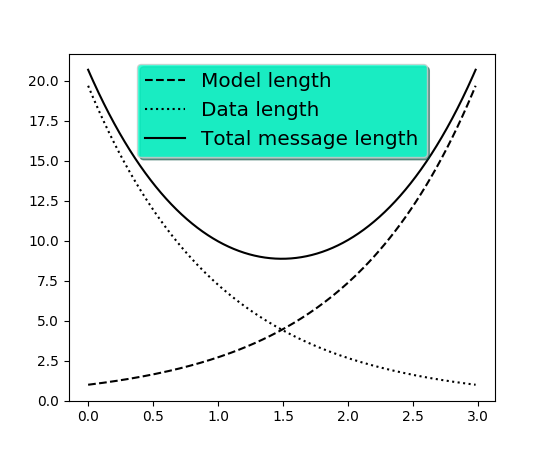`matplotlib.pyplot.``locator_params`(axis='both', tight=None, **kwargs)

Control behavior of tick locators.

Keyword arguments:

axis
[‘x’ | ‘y’ | ‘both’] Axis on which to operate; default is ‘both’.
tight
[True | False | None] Parameter passed to `autoscale_view()`. Default is None, for no change.

Remaining keyword arguments are passed to directly to the `set_params()` method.

Typically one might want to reduce the maximum number of ticks and use tight bounds when plotting small subplots, for example:

```ax.locator_params(tight=True, nbins=4)
```

Because the locator is involved in autoscaling, `autoscale_view()` is called automatically after the parameters are changed.

This presently works only for the `MaxNLocator` used by default on linear axes, but it may be generalized.

`matplotlib.pyplot.``loglog`(*args, **kwargs)

Make a plot with log scaling on both the x and y axis.

`loglog()` supports all the keyword arguments of `plot()` and `matplotlib.axes.Axes.set_xscale()` / `matplotlib.axes.Axes.set_yscale()`.

Notable keyword arguments:

basex/basey: scalar > 1
Base of the x/y logarithm
subsx/subsy: [ None | sequence ]
The location of the minor x/y ticks; None defaults to autosubs, which depend on the number of decades in the plot; see `matplotlib.axes.Axes.set_xscale()` / `matplotlib.axes.Axes.set_yscale()` for details
nonposx/nonposy: [‘mask’ | ‘clip’ ]
Non-positive values in x or y can be masked as invalid, or clipped to a very small positive number

The remaining valid kwargs are `Line2D` properties:

Property Description
`agg_filter` unknown
`alpha` float (0.0 transparent through 1.0 opaque)
`animated` [True | False]
`antialiased` or aa [True | False]
`axes` an `Axes` instance
`clip_box` a `matplotlib.transforms.Bbox` instance
`clip_on` [True | False]
`clip_path` [ (`Path`, `Transform`) | `Patch` | None ]
`color` or c any matplotlib color
`contains` a callable function
`dash_capstyle` [‘butt’ | ‘round’ | ‘projecting’]
`dash_joinstyle` [‘miter’ | ‘round’ | ‘bevel’]
`dashes` sequence of on/off ink in points
`drawstyle` [‘default’ | ‘steps’ | ‘steps-pre’ | ‘steps-mid’ | ‘steps-post’]
`figure` a `matplotlib.figure.Figure` instance
`fillstyle` [‘full’ | ‘left’ | ‘right’ | ‘bottom’ | ‘top’ | ‘none’]
`gid` an id string
`label` string or anything printable with ‘%s’ conversion.
`linestyle` or ls [‘solid’ | ‘dashed’, ‘dashdot’, ‘dotted’ | (offset, on-off-dash-seq) | `'-'` | `'--'` | `'-.'` | `':'` | `'None'` | `' '` | `''`]
`linewidth` or lw float value in points
`marker` `A valid marker style`
`markeredgecolor` or mec any matplotlib color
`markeredgewidth` or mew float value in points
`markerfacecolor` or mfc any matplotlib color
`markerfacecoloralt` or mfcalt any matplotlib color
`markersize` or ms float
`markevery` [None | int | length-2 tuple of int | slice | list/array of int | float | length-2 tuple of float]
`path_effects` unknown
`picker` float distance in points or callable pick function `fn(artist, event)`
`pickradius` float distance in points
`rasterized` [True | False | None]
`sketch_params` unknown
`snap` unknown
`solid_capstyle` [‘butt’ | ‘round’ | ‘projecting’]
`solid_joinstyle` [‘miter’ | ‘round’ | ‘bevel’]
`transform` a `matplotlib.transforms.Transform` instance
`url` a url string
`visible` [True | False]
`xdata` 1D array
`ydata` 1D array
`zorder` any number

Example: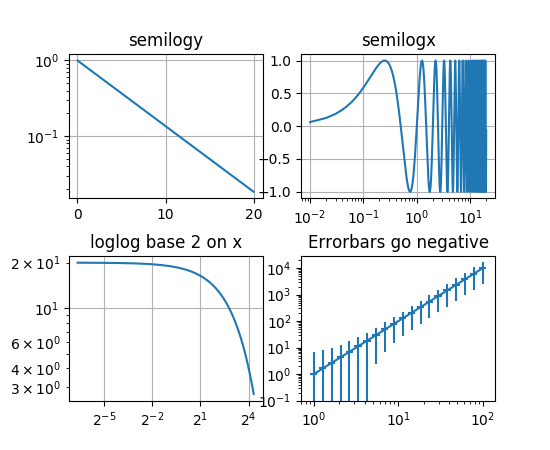`matplotlib.pyplot.``magma`()

set the default colormap to magma and apply to current image if any. See help(colormaps) for more information

`matplotlib.pyplot.``magnitude_spectrum`(x, Fs=None, Fc=None, window=None, pad_to=None, sides=None, scale=None, hold=None, data=None, **kwargs)

Plot the magnitude spectrum.

Call signature:

```magnitude_spectrum(x, Fs=2, Fc=0,  window=mlab.window_hanning,
```

Compute the magnitude spectrum of x. Data is padded to a length of pad_to and the windowing function window is applied to the signal.

Parameters:

x : 1-D array or sequence

Array or sequence containing the data

Fs : scalar

The sampling frequency (samples per time unit). It is used to calculate the Fourier frequencies, freqs, in cycles per time unit. The default value is 2.

window : callable or ndarray

A function or a vector of length NFFT. To create window vectors see `window_hanning()`, `window_none()`, `numpy.blackman()`, `numpy.hamming()`, `numpy.bartlett()`, `scipy.signal()`, `scipy.signal.get_window()`, etc. The default is `window_hanning()`. If a function is passed as the argument, it must take a data segment as an argument and return the windowed version of the segment.

sides : [ ‘default’ | ‘onesided’ | ‘twosided’ ]

Specifies which sides of the spectrum to return. Default gives the default behavior, which returns one-sided for real data and both for complex data. ‘onesided’ forces the return of a one-sided spectrum, while ‘twosided’ forces two-sided.

The number of points to which the data segment is padded when performing the FFT. While not increasing the actual resolution of the spectrum (the minimum distance between resolvable peaks), this can give more points in the plot, allowing for more detail. This corresponds to the n parameter in the call to fft(). The default is None, which sets pad_to equal to the length of the input signal (i.e. no padding).

scale : [ ‘default’ | ‘linear’ | ‘dB’ ]

The scaling of the values in the spec. ‘linear’ is no scaling. ‘dB’ returns the values in dB scale. When mode is ‘density’, this is dB power (10 * log10). Otherwise this is dB amplitude (20 * log10). ‘default’ is ‘linear’.

Fc : integer

The center frequency of x (defaults to 0), which offsets the x extents of the plot to reflect the frequency range used when a signal is acquired and then filtered and downsampled to baseband.

**kwargs :

Keyword arguments control the `Line2D` properties:

Property Description
`agg_filter` unknown
`alpha` float (0.0 transparent through 1.0 opaque)
`animated` [True | False]
`antialiased` or aa [True | False]
`axes` an `Axes` instance
`clip_box` a `matplotlib.transforms.Bbox` instance
`clip_on` [True | False]
`clip_path` [ (`Path`, `Transform`) | `Patch` | None ]
`color` or c any matplotlib color
`contains` a callable function
`dash_capstyle` [‘butt’ | ‘round’ | ‘projecting’]
`dash_joinstyle` [‘miter’ | ‘round’ | ‘bevel’]
`dashes` sequence of on/off ink in points
`drawstyle` [‘default’ | ‘steps’ | ‘steps-pre’ | ‘steps-mid’ | ‘steps-post’]
`figure` a `matplotlib.figure.Figure` instance
`fillstyle` [‘full’ | ‘left’ | ‘right’ | ‘bottom’ | ‘top’ | ‘none’]
`gid` an id string
`label` string or anything printable with ‘%s’ conversion.
`linestyle` or ls [‘solid’ | ‘dashed’, ‘dashdot’, ‘dotted’ | (offset, on-off-dash-seq) | `'-'` | `'--'` | `'-.'` | `':'` | `'None'` | `' '` | `''`]
`linewidth` or lw float value in points
`marker` `A valid marker style`
`markeredgecolor` or mec any matplotlib color
`markeredgewidth` or mew float value in points
`markerfacecolor` or mfc any matplotlib color
`markerfacecoloralt` or mfcalt any matplotlib color
`markersize` or ms float
`markevery` [None | int | length-2 tuple of int | slice | list/array of int | float | length-2 tuple of float]
`path_effects` unknown
`picker` float distance in points or callable pick function `fn(artist, event)`
`pickradius` float distance in points
`rasterized` [True | False | None]
`sketch_params` unknown
`snap` unknown
`solid_capstyle` [‘butt’ | ‘round’ | ‘projecting’]
`solid_joinstyle` [‘miter’ | ‘round’ | ‘bevel’]
`transform` a `matplotlib.transforms.Transform` instance
`url` a url string
`visible` [True | False]
`xdata` 1D array
`ydata` 1D array
`zorder` any number
Returns:

spectrum : 1-D array

The values for the magnitude spectrum before scaling (real valued)

freqs : 1-D array

The frequencies corresponding to the elements in spectrum

line : a `Line2D` instance

The line created by this function

`psd()`
`psd()` plots the power spectral density.`.
`angle_spectrum()`
`angle_spectrum()` plots the angles of the corresponding frequencies.
`phase_spectrum()`
`phase_spectrum()` plots the phase (unwrapped angle) of the corresponding frequencies.
`specgram()`
`specgram()` can plot the magnitude spectrum of segments within the signal in a colormap.
In addition to the above described arguments, this function can take a data keyword argument. If such a data argument is given, the following arguments are replaced by data[<arg>]: * All arguments with the following names: ‘x’.

Examples`matplotlib.pyplot.``margins`(*args, **kw)

Set or retrieve autoscaling margins.

signatures:

```margins()
```

returns xmargin, ymargin

```margins(margin)

margins(xmargin, ymargin)

margins(x=xmargin, y=ymargin)

margins(..., tight=False)
```

All three forms above set the xmargin and ymargin parameters. All keyword parameters are optional. A single argument specifies both xmargin and ymargin. The tight parameter is passed to `autoscale_view()`, which is executed after a margin is changed; the default here is True, on the assumption that when margins are specified, no additional padding to match tick marks is usually desired. Setting tight to None will preserve the previous setting.

Specifying any margin changes only the autoscaling; for example, if xmargin is not None, then xmargin times the X data interval will be added to each end of that interval before it is used in autoscaling.

`matplotlib.pyplot.``matshow`(A, fignum=None, **kw)

Display an array as a matrix in a new figure window.

The origin is set at the upper left hand corner and rows (first dimension of the array) are displayed horizontally. The aspect ratio of the figure window is that of the array, unless this would make an excessively short or narrow figure.

Tick labels for the xaxis are placed on top.

With the exception of fignum, keyword arguments are passed to `imshow()`. You may set the origin kwarg to “lower” if you want the first row in the array to be at the bottom instead of the top.

fignum: [ None | integer | False ]

By default, `matshow()` creates a new figure window with automatic numbering. If fignum is given as an integer, the created figure will use this figure number. Because of how `matshow()` tries to set the figure aspect ratio to be the one of the array, if you provide the number of an already existing figure, strange things may happen.

If fignum is False or 0, a new figure window will NOT be created.

`matplotlib.pyplot.``minorticks_off`()

Remove minor ticks from the current plot.

`matplotlib.pyplot.``minorticks_on`()

Display minor ticks on the current plot.

Displaying minor ticks reduces performance; turn them off using minorticks_off() if drawing speed is a problem.

`matplotlib.pyplot.``nipy_spectral`()

set the default colormap to nipy_spectral and apply to current image if any. See help(colormaps) for more information

`matplotlib.pyplot.``over`(func, *args, **kwargs)

Deprecated since version 2.0: pyplot.hold is deprecated. Future behavior will be consistent with the long-time default: plot commands add elements without first clearing the Axes and/or Figure.

Call a function with hold(True).

Calls:

```func(*args, **kwargs)
```

with `hold(True)` and then restores the hold state.

`matplotlib.pyplot.``pause`(interval)

Pause for interval seconds.

If there is an active figure it will be updated and displayed, and the GUI event loop will run during the pause.

If there is no active figure, or if a non-interactive backend is in use, this executes time.sleep(interval).

This can be used for crude animation. For more complex animation, see `matplotlib.animation`.

This function is experimental; its behavior may be changed or extended in a future release.

`matplotlib.pyplot.``pcolor`(*args, **kwargs)

Create a pseudocolor plot of a 2-D array.

Note

pcolor can be very slow for large arrays; consider using the similar but much faster `pcolormesh()` instead.

Call signatures:

```pcolor(C, **kwargs)
pcolor(X, Y, C, **kwargs)
```

C is the array of color values.

X and Y, if given, specify the (x, y) coordinates of the colored quadrilaterals; the quadrilateral for C[i,j] has corners at:

```(X[i,   j],   Y[i,   j]),
(X[i,   j+1], Y[i,   j+1]),
(X[i+1, j],   Y[i+1, j]),
(X[i+1, j+1], Y[i+1, j+1]).
```

Ideally the dimensions of X and Y should be one greater than those of C; if the dimensions are the same, then the last row and column of C will be ignored.

Note that the column index corresponds to the x-coordinate, and the row index corresponds to y; for details, see the Grid Orientation section below.

If either or both of X and Y are 1-D arrays or column vectors, they will be expanded as needed into the appropriate 2-D arrays, making a rectangular grid.

X, Y and C may be masked arrays. If either C[i, j], or one of the vertices surrounding C[i,j] (X or Y at [i, j], [i+1, j], [i, j+1],[i+1, j+1]) is masked, nothing is plotted.

Keyword arguments:

cmap: [ None | Colormap ]
A `matplotlib.colors.Colormap` instance. If None, use rc settings.
norm: [ None | Normalize ]
An `matplotlib.colors.Normalize` instance is used to scale luminance data to 0,1. If None, defaults to `normalize()`.
vmin/vmax: [ None | scalar ]
vmin and vmax are used in conjunction with norm to normalize luminance data. If either is None, it is autoscaled to the respective min or max of the color array C. If not None, vmin or vmax passed in here override any pre-existing values supplied in the norm instance.
shading: [ ‘flat’ | ‘faceted’ ]

If ‘faceted’, a black grid is drawn around each rectangle; if ‘flat’, edges are not drawn. Default is ‘flat’, contrary to MATLAB.

This kwarg is deprecated; please use ‘edgecolors’ instead:
• shading=’flat’ – edgecolors=’none’
• shading=’faceted – edgecolors=’k’
edgecolors: [ None | `'none'` | color | color sequence]

If None, the rc setting is used by default.

If `'none'`, edges will not be visible.

An mpl color or sequence of colors will set the edge color

alpha: `0 <= scalar <= 1` or None
the alpha blending value
snap: bool
Whether to snap the mesh to pixel boundaries.

Return value is a `matplotlib.collections.Collection` instance.

The grid orientation follows the MATLAB convention: an array C with shape (nrows, ncolumns) is plotted with the column number as X and the row number as Y, increasing up; hence it is plotted the way the array would be printed, except that the Y axis is reversed. That is, C is taken as C*(*y, x).

Similarly for `meshgrid()`:

```x = np.arange(5)
y = np.arange(3)
X, Y = np.meshgrid(x, y)
```

is equivalent to:

```X = array([[0, 1, 2, 3, 4],
[0, 1, 2, 3, 4],
[0, 1, 2, 3, 4]])

Y = array([[0, 0, 0, 0, 0],
[1, 1, 1, 1, 1],
[2, 2, 2, 2, 2]])
```

so if you have:

```C = rand(len(x), len(y))
```

then you need to transpose C:

```pcolor(X, Y, C.T)
```

or:

```pcolor(C.T)
```

MATLAB `pcolor()` always discards the last row and column of C, but matplotlib displays the last row and column if X and Y are not specified, or if X and Y have one more row and column than C.

kwargs can be used to control the `PolyCollection` properties:

Property Description
`agg_filter` unknown
`alpha` float or None
`animated` [True | False]
`antialiased` or antialiaseds Boolean or sequence of booleans
`array` unknown
`axes` an `Axes` instance
`clim` a length 2 sequence of floats
`clip_box` a `matplotlib.transforms.Bbox` instance
`clip_on` [True | False]
`clip_path` [ (`Path`, `Transform`) | `Patch` | None ]
`cmap` a colormap or registered colormap name
`color` matplotlib color arg or sequence of rgba tuples
`contains` a callable function
`edgecolor` or edgecolors matplotlib color spec or sequence of specs
`facecolor` or facecolors matplotlib color spec or sequence of specs
`figure` a `matplotlib.figure.Figure` instance
`gid` an id string
`hatch` [ ‘/’ | ‘\’ | ‘|’ | ‘-‘ | ‘+’ | ‘x’ | ‘o’ | ‘O’ | ‘.’ | ‘*’ ]
`label` string or anything printable with ‘%s’ conversion.
`linestyle` or dashes or linestyles [‘solid’ | ‘dashed’, ‘dashdot’, ‘dotted’ | (offset, on-off-dash-seq) | `'-'` | `'--'` | `'-.'` | `':'` | `'None'` | `' '` | `''`]
`linewidth` or linewidths or lw float or sequence of floats
`norm` unknown
`offset_position` unknown
`offsets` float or sequence of floats
`path_effects` unknown
`picker` [None|float|boolean|callable]
`pickradius` unknown
`rasterized` [True | False | None]
`sketch_params` unknown
`snap` unknown
`transform` `Transform` instance
`url` a url string
`urls` unknown
`visible` [True | False]
`zorder` any number

Note

The default antialiaseds is False if the default edgecolors*=”none” is used. This eliminates artificial lines at patch boundaries, and works regardless of the value of alpha. If *edgecolors is not “none”, then the default antialiaseds is taken from rcParams[‘patch.antialiased’], which defaults to True. Stroking the edges may be preferred if alpha is 1, but will cause artifacts otherwise.

`pcolormesh()`
For an explanation of the differences between pcolor and pcolormesh.

Note

In addition to the above described arguments, this function can take a data keyword argument. If such a data argument is given, the following arguments are replaced by data[<arg>]:

• All positional and all keyword arguments.
`matplotlib.pyplot.``pcolormesh`(*args, **kwargs)

Plot a quadrilateral mesh.

Call signatures:

```pcolormesh(C)
pcolormesh(X, Y, C)
pcolormesh(C, **kwargs)
```

Create a pseudocolor plot of a 2-D array.

pcolormesh is similar to `pcolor()`, but uses a different mechanism and returns a different object; pcolor returns a `PolyCollection` but pcolormesh returns a `QuadMesh`. It is much faster, so it is almost always preferred for large arrays.

C may be a masked array, but X and Y may not. Masked array support is implemented via cmap and norm; in contrast, `pcolor()` simply does not draw quadrilaterals with masked colors or vertices.

Keyword arguments:

cmap: [ None | Colormap ]
A `matplotlib.colors.Colormap` instance. If None, use rc settings.
norm: [ None | Normalize ]
A `matplotlib.colors.Normalize` instance is used to scale luminance data to 0,1. If None, defaults to `normalize()`.
vmin/vmax: [ None | scalar ]
vmin and vmax are used in conjunction with norm to normalize luminance data. If either is None, it is autoscaled to the respective min or max of the color array C. If not None, vmin or vmax passed in here override any pre-existing values supplied in the norm instance.
shading: [ ‘flat’ | ‘gouraud’ ]
‘flat’ indicates a solid color for each quad. When ‘gouraud’, each quad will be Gouraud shaded. When gouraud shading, edgecolors is ignored.
edgecolors: [None | `'None'` | `'face'` | color |
color sequence]

If None, the rc setting is used by default.

If `'None'`, edges will not be visible.

If `'face'`, edges will have the same color as the faces.

An mpl color or sequence of colors will set the edge color

alpha: `0 <= scalar <= 1` or None
the alpha blending value

Return value is a `matplotlib.collections.QuadMesh` object.

kwargs can be used to control the `matplotlib.collections.QuadMesh` properties:

Property Description
`agg_filter` unknown
`alpha` float or None
`animated` [True | False]
`antialiased` or antialiaseds Boolean or sequence of booleans
`array` unknown
`axes` an `Axes` instance
`clim` a length 2 sequence of floats
`clip_box` a `matplotlib.transforms.Bbox` instance
`clip_on` [True | False]
`clip_path` [ (`Path`, `Transform`) | `Patch` | None ]
`cmap` a colormap or registered colormap name
`color` matplotlib color arg or sequence of rgba tuples
`contains` a callable function
`edgecolor` or edgecolors matplotlib color spec or sequence of specs
`facecolor` or facecolors matplotlib color spec or sequence of specs
`figure` a `matplotlib.figure.Figure` instance
`gid` an id string
`hatch` [ ‘/’ | ‘\’ | ‘|’ | ‘-‘ | ‘+’ | ‘x’ | ‘o’ | ‘O’ | ‘.’ | ‘*’ ]
`label` string or anything printable with ‘%s’ conversion.
`linestyle` or dashes or linestyles [‘solid’ | ‘dashed’, ‘dashdot’, ‘dotted’ | (offset, on-off-dash-seq) | `'-'` | `'--'` | `'-.'` | `':'` | `'None'` | `' '` | `''`]
`linewidth` or linewidths or lw float or sequence of floats
`norm` unknown
`offset_position` unknown
`offsets` float or sequence of floats
`path_effects` unknown
`picker` [None|float|boolean|callable]
`pickradius` unknown
`rasterized` [True | False | None]
`sketch_params` unknown
`snap` unknown
`transform` `Transform` instance
`url` a url string
`urls` unknown
`visible` [True | False]
`zorder` any number

`pcolor()`
For an explanation of the grid orientation (Grid Orientation) and the expansion of 1-D X and/or Y to 2-D arrays.

Note

In addition to the above described arguments, this function can take a data keyword argument. If such a data argument is given, the following arguments are replaced by data[<arg>]:

• All positional and all keyword arguments.
`matplotlib.pyplot.``phase_spectrum`(x, Fs=None, Fc=None, window=None, pad_to=None, sides=None, hold=None, data=None, **kwargs)

Plot the phase spectrum.

Call signature:

```phase_spectrum(x, Fs=2, Fc=0,  window=mlab.window_hanning,
```

Compute the phase spectrum (unwrapped angle spectrum) of x. Data is padded to a length of pad_to and the windowing function window is applied to the signal.

Parameters:

x : 1-D array or sequence

Array or sequence containing the data

Fs : scalar

The sampling frequency (samples per time unit). It is used to calculate the Fourier frequencies, freqs, in cycles per time unit. The default value is 2.

window : callable or ndarray

A function or a vector of length NFFT. To create window vectors see `window_hanning()`, `window_none()`, `numpy.blackman()`, `numpy.hamming()`, `numpy.bartlett()`, `scipy.signal()`, `scipy.signal.get_window()`, etc. The default is `window_hanning()`. If a function is passed as the argument, it must take a data segment as an argument and return the windowed version of the segment.

sides : [ ‘default’ | ‘onesided’ | ‘twosided’ ]

Specifies which sides of the spectrum to return. Default gives the default behavior, which returns one-sided for real data and both for complex data. ‘onesided’ forces the return of a one-sided spectrum, while ‘twosided’ forces two-sided.

The number of points to which the data segment is padded when performing the FFT. While not increasing the actual resolution of the spectrum (the minimum distance between resolvable peaks), this can give more points in the plot, allowing for more detail. This corresponds to the n parameter in the call to fft(). The default is None, which sets pad_to equal to the length of the input signal (i.e. no padding).

Fc : integer

The center frequency of x (defaults to 0), which offsets the x extents of the plot to reflect the frequency range used when a signal is acquired and then filtered and downsampled to baseband.

**kwargs :

Keyword arguments control the `Line2D` properties:

Property Description
`agg_filter` unknown
`alpha` float (0.0 transparent through 1.0 opaque)
`animated` [True | False]
`antialiased` or aa [True | False]
`axes` an `Axes` instance
`clip_box` a `matplotlib.transforms.Bbox` instance
`clip_on` [True | False]
`clip_path` [ (`Path`, `Transform`) | `Patch` | None ]
`color` or c any matplotlib color
`contains` a callable function
`dash_capstyle` [‘butt’ | ‘round’ | ‘projecting’]
`dash_joinstyle` [‘miter’ | ‘round’ | ‘bevel’]
`dashes` sequence of on/off ink in points
`drawstyle` [‘default’ | ‘steps’ | ‘steps-pre’ | ‘steps-mid’ | ‘steps-post’]
`figure` a `matplotlib.figure.Figure` instance
`fillstyle` [‘full’ | ‘left’ | ‘right’ | ‘bottom’ | ‘top’ | ‘none’]
`gid` an id string
`label` string or anything printable with ‘%s’ conversion.
`linestyle` or ls [‘solid’ | ‘dashed’, ‘dashdot’, ‘dotted’ | (offset, on-off-dash-seq) | `'-'` | `'--'` | `'-.'` | `':'` | `'None'` | `' '` | `''`]
`linewidth` or lw float value in points
`marker` `A valid marker style`
`markeredgecolor` or mec any matplotlib color
`markeredgewidth` or mew float value in points
`markerfacecolor` or mfc any matplotlib color
`markerfacecoloralt` or mfcalt any matplotlib color
`markersize` or ms float
`markevery` [None | int | length-2 tuple of int | slice | list/array of int | float | length-2 tuple of float]
`path_effects` unknown
`picker` float distance in points or callable pick function `fn(artist, event)`
`pickradius` float distance in points
`rasterized` [True | False | None]
`sketch_params` unknown
`snap` unknown
`solid_capstyle` [‘butt’ | ‘round’ | ‘projecting’]
`solid_joinstyle` [‘miter’ | ‘round’ | ‘bevel’]
`transform` a `matplotlib.transforms.Transform` instance
`url` a url string
`visible` [True | False]
`xdata` 1D array
`ydata` 1D array
`zorder` any number
Returns:

spectrum : 1-D array

The values for the phase spectrum in radians (real valued)

freqs : 1-D array

The frequencies corresponding to the elements in spectrum

line : a `Line2D` instance

The line created by this function

`magnitude_spectrum()`
`magnitude_spectrum()` plots the magnitudes of the corresponding frequencies.
`angle_spectrum()`
`angle_spectrum()` plots the wrapped version of this function.
`specgram()`
`specgram()` can plot the phase spectrum of segments within the signal in a colormap.
In addition to the above described arguments, this function can take a data keyword argument. If such a data argument is given, the following arguments are replaced by data[<arg>]: * All arguments with the following names: ‘x’.

Examples`matplotlib.pyplot.``pie`(x, explode=None, labels=None, colors=None, autopct=None, pctdistance=0.6, shadow=False, labeldistance=1.1, startangle=None, radius=None, counterclock=True, wedgeprops=None, textprops=None, center=(0, 0), frame=False, hold=None, data=None)

Plot a pie chart.

Make a pie chart of array x. The fractional area of each wedge is given by `x/sum(x)`. If `sum(x) <= 1`, then the values of x give the fractional area directly and the array will not be normalized. The wedges are plotted counterclockwise, by default starting from the x-axis.

Parameters: x : array-like The input array used to make the pie chart. explode : array-like, optional, default: None If not None, is a `len(x)` array which specifies the fraction of the radius with which to offset each wedge. labels : list, optional, default: None A sequence of strings providing the labels for each wedge colors : array-like, optional, default: None A sequence of matplotlib color args through which the pie chart will cycle. If `None`, will use the colors in the currently active cycle. autopct : None (default), string, or function, optional If not None, is a string or function used to label the wedges with their numeric value. The label will be placed inside the wedge. If it is a format string, the label will be `fmt%pct`. If it is a function, it will be called. pctdistance : float, optional, default: 0.6 The ratio between the center of each pie slice and the start of the text generated by autopct. Ignored if autopct is None. shadow : bool, optional, default: False Draw a shadow beneath the pie. labeldistance : float, optional, default: 1.1 The radial distance at which the pie labels are drawn startangle : float, optional, default: None If not None, rotates the start of the pie chart by angle degrees counterclockwise from the x-axis. radius : float, optional, default: None The radius of the pie, if radius is None it will be set to 1. counterclock : bool, optional, default: True Specify fractions direction, clockwise or counterclockwise. wedgeprops : dict, optional, default: None Dict of arguments passed to the wedge objects making the pie. For example, you can pass in``wedgeprops = {‘linewidth’: 3}`` to set the width of the wedge border lines equal to 3. For more details, look at the doc/arguments of the wedge object. By default `clip_on=False`. textprops : dict, optional, default: None Dict of arguments to pass to the text objects. center : list of float, optional, default: (0, 0) Center position of the chart. Takes value (0, 0) or is a sequence of 2 scalars. frame : bool, optional, default: False Plot axes frame with the chart if true. patches : list A sequence of `matplotlib.patches.Wedge` instances texts : list A is a list of the label `matplotlib.text.Text` instances. autotexts : list A is a list of `Text` instances for the numeric labels. Is returned only if parameter autopct is not None.

Notes

The pie chart will probably look best if the figure and axes are square, or the Axes aspect is equal.

Examples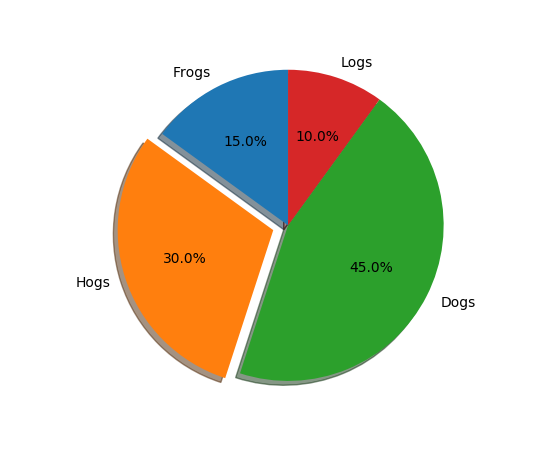Note

In addition to the above described arguments, this function can take a data keyword argument. If such a data argument is given, the following arguments are replaced by data[<arg>]:

• All arguments with the following names: ‘colors’, ‘explode’, ‘labels’, ‘x’.
`matplotlib.pyplot.``pink`()

set the default colormap to pink and apply to current image if any. See help(colormaps) for more information

`matplotlib.pyplot.``plasma`()

set the default colormap to plasma and apply to current image if any. See help(colormaps) for more information

`matplotlib.pyplot.``plot`(*args, **kwargs)

Plot lines and/or markers to the `Axes`. args is a variable length argument, allowing for multiple x, y pairs with an optional format string. For example, each of the following is legal:

```plot(x, y)        # plot x and y using default line style and color
plot(x, y, 'bo')  # plot x and y using blue circle markers
plot(y)           # plot y using x as index array 0..N-1
plot(y, 'r+')     # ditto, but with red plusses
```

If x and/or y is 2-dimensional, then the corresponding columns will be plotted.

If used with labeled data, make sure that the color spec is not included as an element in data, as otherwise the last case `plot("v","r", data={"v":..., "r":...)` can be interpreted as the first case which would do `plot(v, r)` using the default line style and color.

If not used with labeled data (i.e., without a data argument), an arbitrary number of x, y, fmt groups can be specified, as in:

```a.plot(x1, y1, 'g^', x2, y2, 'g-')
```

Return value is a list of lines that were added.

By default, each line is assigned a different style specified by a ‘style cycle’. To change this behavior, you can edit the axes.prop_cycle rcParam.

The following format string characters are accepted to control the line style or marker:

character description
`'-'` solid line style
`'--'` dashed line style
`'-.'` dash-dot line style
`':'` dotted line style
`'.'` point marker
`','` pixel marker
`'o'` circle marker
`'v'` triangle_down marker
`'^'` triangle_up marker
`'<'` triangle_left marker
`'>'` triangle_right marker
`'1'` tri_down marker
`'2'` tri_up marker
`'3'` tri_left marker
`'4'` tri_right marker
`'s'` square marker
`'p'` pentagon marker
`'*'` star marker
`'h'` hexagon1 marker
`'H'` hexagon2 marker
`'+'` plus marker
`'x'` x marker
`'D'` diamond marker
`'d'` thin_diamond marker
`'|'` vline marker
`'_'` hline marker

The following color abbreviations are supported:

character color
‘b’ blue
‘g’ green
‘r’ red
‘c’ cyan
‘m’ magenta
‘y’ yellow
‘k’ black
‘w’ white

In addition, you can specify colors in many weird and wonderful ways, including full names (`'green'`), hex strings (`'#008000'`), RGB or RGBA tuples (`(0,1,0,1)`) or grayscale intensities as a string (`'0.8'`). Of these, the string specifications can be used in place of a `fmt` group, but the tuple forms can be used only as `kwargs`.

Line styles and colors are combined in a single format string, as in `'bo'` for blue circles.

The kwargs can be used to set line properties (any property that has a `set_*` method). You can use this to set a line label (for auto legends), linewidth, anitialising, marker face color, etc. Here is an example:

```plot([1,2,3], [1,2,3], 'go-', label='line 1', linewidth=2)
plot([1,2,3], [1,4,9], 'rs',  label='line 2')
axis([0, 4, 0, 10])
legend()
```

If you make multiple lines with one plot command, the kwargs apply to all those lines, e.g.:

```plot(x1, y1, x2, y2, antialiased=False)
```

Neither line will be antialiased.

You do not need to use format strings, which are just abbreviations. All of the line properties can be controlled by keyword arguments. For example, you can set the color, marker, linestyle, and markercolor with:

```plot(x, y, color='green', linestyle='dashed', marker='o',
markerfacecolor='blue', markersize=12).
```

See `Line2D` for details.

The kwargs are `Line2D` properties:

Property Description
`agg_filter` unknown
`alpha` float (0.0 transparent through 1.0 opaque)
`animated` [True | False]
`antialiased` or aa [True | False]
`axes` an `Axes` instance
`clip_box` a `matplotlib.transforms.Bbox` instance
`clip_on` [True | False]
`clip_path` [ (`Path`, `Transform`) | `Patch` | None ]
`color` or c any matplotlib color
`contains` a callable function
`dash_capstyle` [‘butt’ | ‘round’ | ‘projecting’]
`dash_joinstyle` [‘miter’ | ‘round’ | ‘bevel’]
`dashes` sequence of on/off ink in points
`drawstyle` [‘default’ | ‘steps’ | ‘steps-pre’ | ‘steps-mid’ | ‘steps-post’]
`figure` a `matplotlib.figure.Figure` instance
`fillstyle` [‘full’ | ‘left’ | ‘right’ | ‘bottom’ | ‘top’ | ‘none’]
`gid` an id string
`label` string or anything printable with ‘%s’ conversion.
`linestyle` or ls [‘solid’ | ‘dashed’, ‘dashdot’, ‘dotted’ | (offset, on-off-dash-seq) | `'-'` | `'--'` | `'-.'` | `':'` | `'None'` | `' '` | `''`]
`linewidth` or lw float value in points
`marker` `A valid marker style`
`markeredgecolor` or mec any matplotlib color
`markeredgewidth` or mew float value in points
`markerfacecolor` or mfc any matplotlib color
`markerfacecoloralt` or mfcalt any matplotlib color
`markersize` or ms float
`markevery` [None | int | length-2 tuple of int | slice | list/array of int | float | length-2 tuple of float]
`path_effects` unknown
`picker` float distance in points or callable pick function `fn(artist, event)`
`pickradius` float distance in points
`rasterized` [True | False | None]
`sketch_params` unknown
`snap` unknown
`solid_capstyle` [‘butt’ | ‘round’ | ‘projecting’]
`solid_joinstyle` [‘miter’ | ‘round’ | ‘bevel’]
`transform` a `matplotlib.transforms.Transform` instance
`url` a url string
`visible` [True | False]
`xdata` 1D array
`ydata` 1D array
`zorder` any number

kwargs scalex and scaley, if defined, are passed on to `autoscale_view()` to determine whether the x and y axes are autoscaled; the default is True.

Note

In addition to the above described arguments, this function can take a data keyword argument. If such a data argument is given, the following arguments are replaced by data[<arg>]:

• All arguments with the following names: ‘x’, ‘y’.
`matplotlib.pyplot.``plot_date`(x, y, fmt='o', tz=None, xdate=True, ydate=False, hold=None, data=None, **kwargs)

A plot with data that contains dates.

Similar to the `plot()` command, except the x or y (or both) data is considered to be dates, and the axis is labeled accordingly.

x and/or y can be a sequence of dates represented as float days since 0001-01-01 UTC.

Note if you are using custom date tickers and formatters, it may be necessary to set the formatters/locators after the call to meth:`plot_date` since meth:`plot_date` will set the default tick locator to class:`matplotlib.dates.AutoDateLocator` (if the tick locator is not already set to a class:`matplotlib.dates.DateLocator` instance) and the default tick formatter to class:`matplotlib.dates.AutoDateFormatter` (if the tick formatter is not already set to a class:`matplotlib.dates.DateFormatter` instance).

Parameters:

fmt : string

The plot format string.

tz : [ None | timezone string | `tzinfo` instance]

The time zone to use in labeling dates. If None, defaults to rc value.

xdate : boolean

If True, the x-axis will be labeled with dates.

ydate : boolean

If True, the y-axis will be labeled with dates.

Returns:

lines

Other Parameters:

properties :

Property Description
`agg_filter` unknown
`alpha` float (0.0 transparent through 1.0 opaque)
`animated` [True | False]
`antialiased` or aa [True | False]
`axes` an `Axes` instance
`clip_box` a `matplotlib.transforms.Bbox` instance
`clip_on` [True | False]
`clip_path` [ (`Path`, `Transform`) | `Patch` | None ]
`color` or c any matplotlib color
`contains` a callable function
`dash_capstyle` [‘butt’ | ‘round’ | ‘projecting’]
`dash_joinstyle` [‘miter’ | ‘round’ | ‘bevel’]
`dashes` sequence of on/off ink in points
`drawstyle` [‘default’ | ‘steps’ | ‘steps-pre’ | ‘steps-mid’ | ‘steps-post’]
`figure` a `matplotlib.figure.Figure` instance
`fillstyle` [‘full’ | ‘left’ | ‘right’ | ‘bottom’ | ‘top’ | ‘none’]
`gid` an id string
`label` string or anything printable with ‘%s’ conversion.
`linestyle` or ls [‘solid’ | ‘dashed’, ‘dashdot’, ‘dotted’ | (offset, on-off-dash-seq) | `'-'` | `'--'` | `'-.'` | `':'` | `'None'` | `' '` | `''`]
`linewidth` or lw float value in points
`marker` `A valid marker style`
`markeredgecolor` or mec any matplotlib color
`markeredgewidth` or mew float value in points
`markerfacecolor` or mfc any matplotlib color
`markerfacecoloralt` or mfcalt any matplotlib color
`markersize` or ms float
`markevery` [None | int | length-2 tuple of int | slice | list/array of int | float | length-2 tuple of float]
`path_effects` unknown
`picker` float distance in points or callable pick function `fn(artist, event)`
`pickradius` float distance in points
`rasterized` [True | False | None]
`sketch_params` unknown
`snap` unknown
`solid_capstyle` [‘butt’ | ‘round’ | ‘projecting’]
`solid_joinstyle` [‘miter’ | ‘round’ | ‘bevel’]
`transform` a `matplotlib.transforms.Transform` instance
`url` a url string
`visible` [True | False]
`xdata` 1D array
`ydata` 1D array
`zorder` any number

.. note::

In addition to the above described arguments, this function can take a data keyword argument. If such a data argument is given, the following arguments are replaced by data[<arg>]:

• All arguments with the following names: ‘x’, ‘y’.

`matplotlib.dates`
helper functions on dates
`matplotlib.dates.date2num`
how to convert dates to num
`matplotlib.dates.num2date`
how to convert num to dates
`matplotlib.dates.drange`
how floating point dates
`matplotlib.pyplot.``plotfile`(fname, cols=(0, ), plotfuncs=None, comments='#', skiprows=0, checkrows=5, delimiter=', ', names=None, subplots=True, newfig=True, **kwargs)

Plot the data in in a file.

cols is a sequence of column identifiers to plot. An identifier is either an int or a string. If it is an int, it indicates the column number. If it is a string, it indicates the column header. matplotlib will make column headers lower case, replace spaces with underscores, and remove all illegal characters; so `'Adj Close*'` will have name `'adj_close'`.

• If len(cols) == 1, only that column will be plotted on the y axis.
• If len(cols) > 1, the first element will be an identifier for data for the x axis and the remaining elements will be the column indexes for multiple subplots if subplots is True (the default), or for lines in a single subplot if subplots is False.

plotfuncs, if not None, is a dictionary mapping identifier to an `Axes` plotting function as a string. Default is ‘plot’, other choices are ‘semilogy’, ‘fill’, ‘bar’, etc. You must use the same type of identifier in the cols vector as you use in the plotfuncs dictionary, e.g., integer column numbers in both or column names in both. If subplots is False, then including any function such as ‘semilogy’ that changes the axis scaling will set the scaling for all columns.

comments, skiprows, checkrows, delimiter, and names are all passed on to `matplotlib.pylab.csv2rec()` to load the data into a record array.

If newfig is True, the plot always will be made in a new figure; if False, it will be made in the current figure if one exists, else in a new figure.

kwargs are passed on to plotting functions.

Example usage:

```# plot the 2nd and 4th column against the 1st in two subplots
plotfile(fname, (0,1,3))

# plot using column names; specify an alternate plot type for volume
plotfile(fname, ('date', 'volume', 'adj_close'),
plotfuncs={'volume': 'semilogy'})
```

Note: plotfile is intended as a convenience for quickly plotting data from flat files; it is not intended as an alternative interface to general plotting with pyplot or matplotlib.

`matplotlib.pyplot.``polar`(*args, **kwargs)

Make a polar plot.

call signature:

```polar(theta, r, **kwargs)
```

Multiple theta, r arguments are supported, with format strings, as in `plot()`.

`matplotlib.pyplot.``prism`()

set the default colormap to prism and apply to current image if any. See help(colormaps) for more information

`matplotlib.pyplot.``psd`(x, NFFT=None, Fs=None, Fc=None, detrend=None, window=None, noverlap=None, pad_to=None, sides=None, scale_by_freq=None, return_line=None, hold=None, data=None, **kwargs)

Plot the power spectral density.

Call signature:

```psd(x, NFFT=256, Fs=2, Fc=0, detrend=mlab.detrend_none,
sides='default', scale_by_freq=None, return_line=None, **kwargs)
```

The power spectral density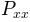by Welch’s average periodogram method. The vector x is divided into NFFT length segments. Each segment is detrended by function detrend and windowed by function window. noverlap gives the length of the overlap between segments. The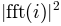of each segment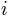are averaged to compute, with a scaling to correct for power loss due to windowing.

If len(x) < NFFT, it will be zero padded to NFFT.

Parameters:

x : 1-D array or sequence

Array or sequence containing the data

Fs : scalar

The sampling frequency (samples per time unit). It is used to calculate the Fourier frequencies, freqs, in cycles per time unit. The default value is 2.

window : callable or ndarray

A function or a vector of length NFFT. To create window vectors see `window_hanning()`, `window_none()`, `numpy.blackman()`, `numpy.hamming()`, `numpy.bartlett()`, `scipy.signal()`, `scipy.signal.get_window()`, etc. The default is `window_hanning()`. If a function is passed as the argument, it must take a data segment as an argument and return the windowed version of the segment.

sides : [ ‘default’ | ‘onesided’ | ‘twosided’ ]

Specifies which sides of the spectrum to return. Default gives the default behavior, which returns one-sided for real data and both for complex data. ‘onesided’ forces the return of a one-sided spectrum, while ‘twosided’ forces two-sided.

The number of points to which the data segment is padded when performing the FFT. This can be different from NFFT, which specifies the number of data points used. While not increasing the actual resolution of the spectrum (the minimum distance between resolvable peaks), this can give more points in the plot, allowing for more detail. This corresponds to the n parameter in the call to fft(). The default is None, which sets pad_to equal to NFFT

NFFT : integer

The number of data points used in each block for the FFT. A power 2 is most efficient. The default value is 256. This should NOT be used to get zero padding, or the scaling of the result will be incorrect. Use pad_to for this instead.

detrend : {‘default’, ‘constant’, ‘mean’, ‘linear’, ‘none’} or callable

The function applied to each segment before fft-ing, designed to remove the mean or linear trend. Unlike in MATLAB, where the detrend parameter is a vector, in matplotlib is it a function. The `pylab` module defines `detrend_none()`, `detrend_mean()`, and `detrend_linear()`, but you can use a custom function as well. You can also use a string to choose one of the functions. ‘default’, ‘constant’, and ‘mean’ call `detrend_mean()`. ‘linear’ calls `detrend_linear()`. ‘none’ calls `detrend_none()`.

scale_by_freq : boolean, optional

Specifies whether the resulting density values should be scaled by the scaling frequency, which gives density in units of Hz^-1. This allows for integration over the returned frequency values. The default is True for MATLAB compatibility.

noverlap : integer

The number of points of overlap between segments. The default value is 0 (no overlap).

Fc : integer

The center frequency of x (defaults to 0), which offsets the x extents of the plot to reflect the frequency range used when a signal is acquired and then filtered and downsampled to baseband.

return_line : bool

Whether to include the line object plotted in the returned values. Default is False.

**kwargs :

Keyword arguments control the `Line2D` properties:

Property Description
`agg_filter` unknown
`alpha` float (0.0 transparent through 1.0 opaque)
`animated` [True | False]
`antialiased` or aa [True | False]
`axes` an `Axes` instance
`clip_box` a `matplotlib.transforms.Bbox` instance
`clip_on` [True | False]
`clip_path` [ (`Path`, `Transform`) | `Patch` | None ]
`color` or c any matplotlib color
`contains` a callable function
`dash_capstyle` [‘butt’ | ‘round’ | ‘projecting’]
`dash_joinstyle` [‘miter’ | ‘round’ | ‘bevel’]
`dashes` sequence of on/off ink in points
`drawstyle` [‘default’ | ‘steps’ | ‘steps-pre’ | ‘steps-mid’ | ‘steps-post’]
`figure` a `matplotlib.figure.Figure` instance
`fillstyle` [‘full’ | ‘left’ | ‘right’ | ‘bottom’ | ‘top’ | ‘none’]
`gid` an id string
`label` string or anything printable with ‘%s’ conversion.
`linestyle` or ls [‘solid’ | ‘dashed’, ‘dashdot’, ‘dotted’ | (offset, on-off-dash-seq) | `'-'` | `'--'` | `'-.'` | `':'` | `'None'` | `' '` | `''`]
`linewidth` or lw float value in points
`marker` `A valid marker style`
`markeredgecolor` or mec any matplotlib color
`markeredgewidth` or mew float value in points
`markerfacecolor` or mfc any matplotlib color
`markerfacecoloralt` or mfcalt any matplotlib color
`markersize` or ms float
`markevery` [None | int | length-2 tuple of int | slice | list/array of int | float | length-2 tuple of float]
`path_effects` unknown
`picker` float distance in points or callable pick function `fn(artist, event)`
`pickradius` float distance in points
`rasterized` [True | False | None]
`sketch_params` unknown
`snap` unknown
`solid_capstyle` [‘butt’ | ‘round’ | ‘projecting’]
`solid_joinstyle` [‘miter’ | ‘round’ | ‘bevel’]
`transform` a `matplotlib.transforms.Transform` instance
`url` a url string
`visible` [True | False]
`xdata` 1D array
`ydata` 1D array
`zorder` any number
Returns:

Pxx : 1-D array

The values for the power spectrum `P_{xx}` before scaling (real valued)

freqs : 1-D array

The frequencies corresponding to the elements in Pxx

line : a `Line2D` instance

The line created by this function. Only returned if return_line is True.

`specgram()`
`specgram()` differs in the default overlap; in not returning the mean of the segment periodograms; in returning the times of the segments; and in plotting a colormap instead of a line.
`magnitude_spectrum()`
`magnitude_spectrum()` plots the magnitude spectrum.
`csd()`
`csd()` plots the spectral density between two signals.
In addition to the above described arguments, this function can take a data keyword argument. If such a data argument is given, the following arguments are replaced by data[<arg>]: * All arguments with the following names: ‘x’.

Notes

For plotting, the power is plotted as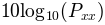for decibels, though Pxx itself is returned.

References

Bendat & Piersol – Random Data: Analysis and Measurement Procedures, John Wiley & Sons (1986)

Examples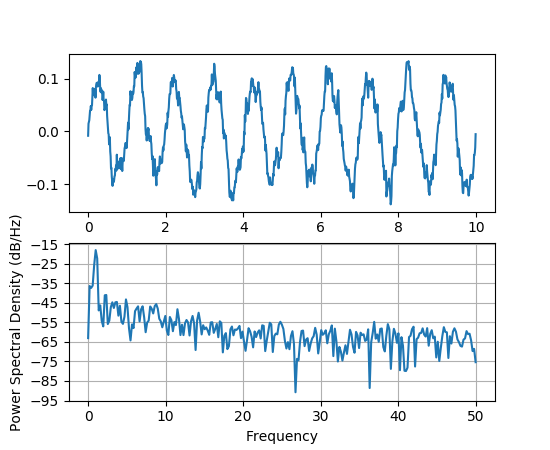`matplotlib.pyplot.``quiver`(*args, **kw)

Plot a 2-D field of arrows.

Call signatures:

```quiver(U, V, **kw)
quiver(U, V, C, **kw)
quiver(X, Y, U, V, **kw)
quiver(X, Y, U, V, C, **kw)
```

U and V are the arrow data, X and Y set the locaiton of the arrows, and C sets the color of the arrows. These arguments may be 1-D or 2-D arrays or sequences.

If X and Y are absent, they will be generated as a uniform grid. If U and V are 2-D arrays and X and Y are 1-D, and if `len(X)` and `len(Y)` match the column and row dimensions of U, then X and Y will be expanded with `numpy.meshgrid()`.

The default settings auto-scales the length of the arrows to a reasonable size. To change this behavior see the scale and scale_units kwargs.

The defaults give a slightly swept-back arrow; to make the head a triangle, make headaxislength the same as headlength. To make the arrow more pointed, reduce headwidth or increase headlength and headaxislength. To make the head smaller relative to the shaft, scale down all the head parameters. You will probably do best to leave minshaft alone.

linewidths and edgecolors can be used to customize the arrow outlines.

Parameters: X : 1D or 2D array, sequence, optional The x coordinates of the arrow locations Y : 1D or 2D array, sequence, optional The y coordinates of the arrow locations U : 1D or 2D array or masked array, sequence The x components of the arrow vectors V : 1D or 2D array or masked array, sequence The y components of the arrow vectors C : 1D or 2D array, sequence, optional The arrow colors units : [ ‘width’ | ‘height’ | ‘dots’ | ‘inches’ | ‘x’ | ‘y’ | ‘xy’ ] The arrow dimensions (except for length) are measured in multiples of this unit. ‘width’ or ‘height’: the width or height of the axis ‘dots’ or ‘inches’: pixels or inches, based on the figure dpi ‘x’, ‘y’, or ‘xy’: respectively X, Y, or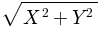in data units The arrows scale differently depending on the units. For ‘x’ or ‘y’, the arrows get larger as one zooms in; for other units, the arrow size is independent of the zoom state. For ‘width or ‘height’, the arrow size increases with the width and height of the axes, respectively, when the window is resized; for ‘dots’ or ‘inches’, resizing does not change the arrows. angles : [ ‘uv’ | ‘xy’ ], array, optional Method for determining the angle of the arrows. Default is ‘uv’. ‘uv’: the arrow axis aspect ratio is 1 so that if U*==*V the orientation of the arrow on the plot is 45 degrees counter-clockwise from the horizontal axis (positive to the right). ‘xy’: arrows point from (x,y) to (x+u, y+v). Use this for plotting a gradient field, for example. Alternatively, arbitrary angles may be specified as an array of values in degrees, counter-clockwise from the horizontal axis. Note: inverting a data axis will correspondingly invert the arrows only with `angles='xy'`. scale : None, float, optional Number of data units per arrow length unit, e.g., m/s per plot width; a smaller scale parameter makes the arrow longer. Default is None. If None, a simple autoscaling algorithm is used, based on the average vector length and the number of vectors. The arrow length unit is given by the scale_units parameter scale_units : [ ‘width’ | ‘height’ | ‘dots’ | ‘inches’ | ‘x’ | ‘y’ | ‘xy’ ], None, optional If the scale kwarg is None, the arrow length unit. Default is None. e.g. scale_units is ‘inches’, scale is 2.0, and `(u,v) = (1,0)`, then the vector will be 0.5 inches long. If scale_units is ‘width’/’height’, then the vector will be half the width/height of the axes. If scale_units is ‘x’ then the vector will be 0.5 x-axis units. To plot vectors in the x-y plane, with u and v having the same units as x and y, use `angles='xy', scale_units='xy', scale=1`. width : scalar, optional Shaft width in arrow units; default depends on choice of units, above, and number of vectors; a typical starting value is about 0.005 times the width of the plot. headwidth : scalar, optional Head width as multiple of shaft width, default is 3 headlength : scalar, optional Head length as multiple of shaft width, default is 5 headaxislength : scalar, optional Head length at shaft intersection, default is 4.5 minshaft : scalar, optional Length below which arrow scales, in units of head length. Do not set this to less than 1, or small arrows will look terrible! Default is 1 minlength : scalar, optional Minimum length as a multiple of shaft width; if an arrow length is less than this, plot a dot (hexagon) of this diameter instead. Default is 1. pivot : [ ‘tail’ | ‘mid’ | ‘middle’ | ‘tip’ ], optional The part of the arrow that is at the grid point; the arrow rotates about this point, hence the name pivot. color : [ color | color sequence ], optional This is a synonym for the `PolyCollection` facecolor kwarg. If C has been set, color has no effect.

`quiverkey`
Add a key to a quiver plot

Notes

Additional `PolyCollection` keyword arguments:

Property Description
`agg_filter` unknown
`alpha` float or None
`animated` [True | False]
`antialiased` or antialiaseds Boolean or sequence of booleans
`array` unknown
`axes` an `Axes` instance
`clim` a length 2 sequence of floats
`clip_box` a `matplotlib.transforms.Bbox` instance
`clip_on` [True | False]
`clip_path` [ (`Path`, `Transform`) | `Patch` | None ]
`cmap` a colormap or registered colormap name
`color` matplotlib color arg or sequence of rgba tuples
`contains` a callable function
`edgecolor` or edgecolors matplotlib color spec or sequence of specs
`facecolor` or facecolors matplotlib color spec or sequence of specs
`figure` a `matplotlib.figure.Figure` instance
`gid` an id string
`hatch` [ ‘/’ | ‘\’ | ‘|’ | ‘-‘ | ‘+’ | ‘x’ | ‘o’ | ‘O’ | ‘.’ | ‘*’ ]
`label` string or anything printable with ‘%s’ conversion.
`linestyle` or dashes or linestyles [‘solid’ | ‘dashed’, ‘dashdot’, ‘dotted’ | (offset, on-off-dash-seq) | `'-'` | `'--'` | `'-.'` | `':'` | `'None'` | `' '` | `''`]
`linewidth` or linewidths or lw float or sequence of floats
`norm` unknown
`offset_position` unknown
`offsets` float or sequence of floats
`path_effects` unknown
`picker` [None|float|boolean|callable]
`pickradius` unknown
`rasterized` [True | False | None]
`sketch_params` unknown
`snap` unknown
`transform` `Transform` instance
`url` a url string
`urls` unknown
`visible` [True | False]
`zorder` any number

Examples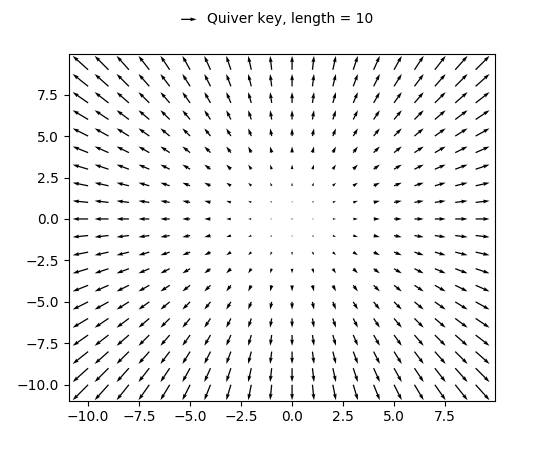`matplotlib.pyplot.``quiverkey`(*args, **kw)

Add a key to a quiver plot.

Call signature:

```quiverkey(Q, X, Y, U, label, **kw)
```

Arguments:

Q:
The Quiver instance returned by a call to quiver.
X, Y:
The location of the key; additional explanation follows.
U:
The length of the key
label:
A string with the length and units of the key

Keyword arguments:

coordinates = [ ‘axes’ | ‘figure’ | ‘data’ | ‘inches’ ]
Coordinate system and units for X, Y: ‘axes’ and ‘figure’ are normalized coordinate systems with 0,0 in the lower left and 1,1 in the upper right; ‘data’ are the axes data coordinates (used for the locations of the vectors in the quiver plot itself); ‘inches’ is position in the figure in inches, with 0,0 at the lower left corner.
color:
overrides face and edge colors from Q.
labelpos = [ ‘N’ | ‘S’ | ‘E’ | ‘W’ ]
Position the label above, below, to the right, to the left of the arrow, respectively.
labelsep:
Distance in inches between the arrow and the label. Default is 0.1
labelcolor:
defaults to default `Text` color.
fontproperties:
A dictionary with keyword arguments accepted by the `FontProperties` initializer: family, style, variant, size, weight

Any additional keyword arguments are used to override vector properties taken from Q.

The positioning of the key depends on X, Y, coordinates, and labelpos. If labelpos is ‘N’ or ‘S’, X, Y give the position of the middle of the key arrow. If labelpos is ‘E’, X, Y positions the head, and if labelpos is ‘W’, X, Y positions the tail; in either of these two cases, X, Y is somewhere in the middle of the arrow+label key object.

`matplotlib.pyplot.``rc`(*args, **kwargs)

Set the current rc params. Group is the grouping for the rc, e.g., for `lines.linewidth` the group is `lines`, for `axes.facecolor`, the group is `axes`, and so on. Group may also be a list or tuple of group names, e.g., (xtick, ytick). kwargs is a dictionary attribute name/value pairs, e.g.,:

```rc('lines', linewidth=2, color='r')
```

sets the current rc params and is equivalent to:

```rcParams['lines.linewidth'] = 2
rcParams['lines.color'] = 'r'
```

The following aliases are available to save typing for interactive users:

Alias Property
‘lw’ ‘linewidth’
‘ls’ ‘linestyle’
‘c’ ‘color’
‘fc’ ‘facecolor’
‘ec’ ‘edgecolor’
‘mew’ ‘markeredgewidth’
‘aa’ ‘antialiased’

Thus you could abbreviate the above rc command as:

```rc('lines', lw=2, c='r')
```

Note you can use python’s kwargs dictionary facility to store dictionaries of default parameters. e.g., you can customize the font rc as follows:

```font = {'family' : 'monospace',
'weight' : 'bold',
'size'   : 'larger'}

rc('font', **font)  # pass in the font dict as kwargs
```

This enables you to easily switch between several configurations. Use `matplotlib.style.use('default')` or `rcdefaults()` to restore the default rc params after changes.

`matplotlib.pyplot.``rc_context`(rc=None, fname=None)

Return a context manager for managing rc settings.

This allows one to do:

```with mpl.rc_context(fname='screen.rc'):
plt.plot(x, a)
with mpl.rc_context(fname='print.rc'):
plt.plot(x, b)
plt.plot(x, c)
```

The ‘a’ vs ‘x’ and ‘c’ vs ‘x’ plots would have settings from ‘screen.rc’, while the ‘b’ vs ‘x’ plot would have settings from ‘print.rc’.

A dictionary can also be passed to the context manager:

```with mpl.rc_context(rc={'text.usetex': True}, fname='screen.rc'):
plt.plot(x, a)
```

The ‘rc’ dictionary takes precedence over the settings loaded from ‘fname’. Passing a dictionary only is also valid.

`matplotlib.pyplot.``rcdefaults`()

Restore the rc params from Matplotlib’s internal defaults.

`rc_file_defaults`
Restore the rc params from the rc file originally loaded by Matplotlib.
`matplotlib.style.use`
Use a specific style file. Call `style.use('default')` to restore the default style.
`matplotlib.pyplot.``rgrids`(*args, **kwargs)

Get or set the radial gridlines on a polar plot.

call signatures:

```lines, labels = rgrids()
lines, labels = rgrids(radii, labels=None, angle=22.5, **kwargs)
```

When called with no arguments, `rgrid()` simply returns the tuple (lines, labels), where lines is an array of radial gridlines (`Line2D` instances) and labels is an array of tick labels (`Text` instances). When called with arguments, the labels will appear at the specified radial distances and angles.

labels, if not None, is a len(radii) list of strings of the labels to use at each angle.

If labels is None, the rformatter will be used

Examples:

```# set the locations of the radial gridlines and labels
lines, labels = rgrids( (0.25, 0.5, 1.0) )

# set the locations and labels of the radial gridlines and labels
lines, labels = rgrids( (0.25, 0.5, 1.0), ('Tom', 'Dick', 'Harry' )
```
`matplotlib.pyplot.``savefig`(*args, **kwargs)

Save the current figure.

Call signature:

```savefig(fname, dpi=None, facecolor='w', edgecolor='w',
orientation='portrait', papertype=None, format=None,
frameon=None)
```

The output formats available depend on the backend being used.

Arguments:

fname:

A string containing a path to a filename, or a Python file-like object, or possibly some backend-dependent object such as `PdfPages`.

If format is None and fname is a string, the output format is deduced from the extension of the filename. If the filename has no extension, the value of the rc parameter `savefig.format` is used.

If fname is not a string, remember to specify format to ensure that the correct backend is used.

Keyword arguments:

dpi: [ None | `scalar > 0` | ‘figure’]
The resolution in dots per inch. If None it will default to the value `savefig.dpi` in the matplotlibrc file. If ‘figure’ it will set the dpi to be the value of the figure.
facecolor, edgecolor:
the colors of the figure rectangle
orientation: [ ‘landscape’ | ‘portrait’ ]
not supported on all backends; currently only on postscript output
papertype:
One of ‘letter’, ‘legal’, ‘executive’, ‘ledger’, ‘a0’ through ‘a10’, ‘b0’ through ‘b10’. Only supported for postscript output.
format:
One of the file extensions supported by the active backend. Most backends support png, pdf, ps, eps and svg.
transparent:
If True, the axes patches will all be transparent; the figure patch will also be transparent unless facecolor and/or edgecolor are specified via kwargs. This is useful, for example, for displaying a plot on top of a colored background on a web page. The transparency of these patches will be restored to their original values upon exit of this function.
frameon:
If True, the figure patch will be colored, if False, the figure background will be transparent. If not provided, the rcParam ‘savefig.frameon’ will be used.
bbox_inches:
Bbox in inches. Only the given portion of the figure is saved. If ‘tight’, try to figure out the tight bbox of the figure.
Amount of padding around the figure when bbox_inches is ‘tight’.
bbox_extra_artists:
A list of extra artists that will be considered when the tight bbox is calculated.
`matplotlib.pyplot.``sca`(ax)

Set the current Axes instance to ax.

The current Figure is updated to the parent of ax.

`matplotlib.pyplot.``scatter`(x, y, s=None, c=None, marker=None, cmap=None, norm=None, vmin=None, vmax=None, alpha=None, linewidths=None, verts=None, edgecolors=None, hold=None, data=None, **kwargs)

Make a scatter plot of `x` vs `y`

Marker size is scaled by `s` and marker color is mapped to `c`

Parameters: Returns: x, y : array_like, shape (n, ) Input data s : scalar or array_like, shape (n, ), optional size in points^2. Default is `rcParams['lines.markersize'] ** 2`. c : color, sequence, or sequence of color, optional, default: ‘b’ `c` can be a single color format string, or a sequence of color specifications of length `N`, or a sequence of `N` numbers to be mapped to colors using the `cmap` and `norm` specified via kwargs (see below). Note that `c` should not be a single numeric RGB or RGBA sequence because that is indistinguishable from an array of values to be colormapped. `c` can be a 2-D array in which the rows are RGB or RGBA, however, including the case of a single row to specify the same color for all points. marker : `MarkerStyle`, optional, default: ‘o’ See `markers` for more information on the different styles of markers scatter supports. `marker` can be either an instance of the class or the text shorthand for a particular marker. cmap : `Colormap`, optional, default: None A `Colormap` instance or registered name. `cmap` is only used if `c` is an array of floats. If None, defaults to rc `image.cmap`. norm : `Normalize`, optional, default: None A `Normalize` instance is used to scale luminance data to 0, 1. `norm` is only used if `c` is an array of floats. If `None`, use the default `normalize()`. vmin, vmax : scalar, optional, default: None `vmin` and `vmax` are used in conjunction with `norm` to normalize luminance data. If either are `None`, the min and max of the color array is used. Note if you pass a `norm` instance, your settings for `vmin` and `vmax` will be ignored. alpha : scalar, optional, default: None The alpha blending value, between 0 (transparent) and 1 (opaque) linewidths : scalar or array_like, optional, default: None If None, defaults to (lines.linewidth,). verts : sequence of (x, y), optional If `marker` is None, these vertices will be used to construct the marker. The center of the marker is located at (0,0) in normalized units. The overall marker is rescaled by `s`. edgecolors : color or sequence of color, optional, default: None If None, defaults to ‘face’ If ‘face’, the edge color will always be the same as the face color. If it is ‘none’, the patch boundary will not be drawn. For non-filled markers, the `edgecolors` kwarg is ignored and forced to ‘face’ internally. paths : `PathCollection` kwargs : `Collection` properties

`plot`
to plot scatter plots when markers are identical in size and color

Notes

• The `plot` function will be faster for scatterplots where markers don’t vary in size or color.

• Any or all of `x`, `y`, `s`, and `c` may be masked arrays, in which case all masks will be combined and only unmasked points will be plotted.

Fundamentally, scatter works with 1-D arrays; `x`, `y`, `s`, and `c` may be input as 2-D arrays, but within scatter they will be flattened. The exception is `c`, which will be flattened only if its size matches the size of `x` and `y`.

Examples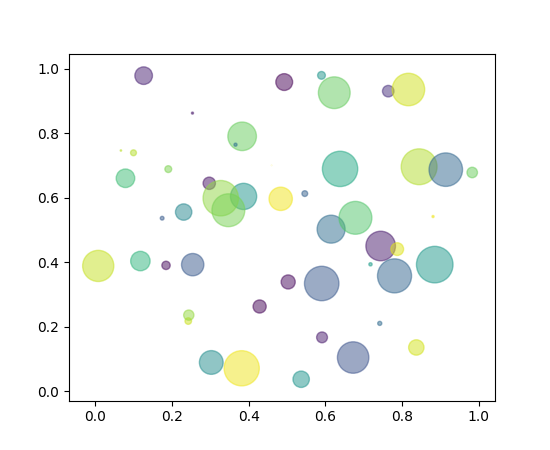Note

In addition to the above described arguments, this function can take a data keyword argument. If such a data argument is given, the following arguments are replaced by data[<arg>]:

• All arguments with the following names: ‘c’, ‘color’, ‘edgecolors’, ‘facecolor’, ‘facecolors’, ‘linewidths’, ‘s’, ‘x’, ‘y’.
`matplotlib.pyplot.``sci`(im)

Set the current image. This image will be the target of colormap commands like `jet()`, `hot()` or `clim()`). The current image is an attribute of the current axes.

`matplotlib.pyplot.``semilogx`(*args, **kwargs)

Make a plot with log scaling on the x axis.

Parameters:

basex : float, optional

Base of the x logarithm. The scalar should be larger than 1.

subsx : array_like, optional

The location of the minor xticks; None defaults to autosubs, which depend on the number of decades in the plot; see `set_xscale()` for details.

nonposx : string, optional, {‘mask’, ‘clip’}

Non-positive values in x can be masked as invalid, or clipped to a very small positive number.

Returns:

`plot`

Log-scaled plot on the x axis.

Other Parameters:

:class:`~matplotlib.lines.Line2D` properties:

Property Description
`agg_filter` unknown
`alpha` float (0.0 transparent through 1.0 opaque)
`animated` [True | False]
`antialiased` or aa [True | False]
`axes` an `Axes` instance
`clip_box` a `matplotlib.transforms.Bbox` instance
`clip_on` [True | False]
`clip_path` [ (`Path`, `Transform`) | `Patch` | None ]
`color` or c any matplotlib color
`contains` a callable function
`dash_capstyle` [‘butt’ | ‘round’ | ‘projecting’]
`dash_joinstyle` [‘miter’ | ‘round’ | ‘bevel’]
`dashes` sequence of on/off ink in points
`drawstyle` [‘default’ | ‘steps’ | ‘steps-pre’ | ‘steps-mid’ | ‘steps-post’]
`figure` a `matplotlib.figure.Figure` instance
`fillstyle` [‘full’ | ‘left’ | ‘right’ | ‘bottom’ | ‘top’ | ‘none’]
`gid` an id string
`label` string or anything printable with ‘%s’ conversion.
`linestyle` or ls [‘solid’ | ‘dashed’, ‘dashdot’, ‘dotted’ | (offset, on-off-dash-seq) | `'-'` | `'--'` | `'-.'` | `':'` | `'None'` | `' '` | `''`]
`linewidth` or lw float value in points
`marker` `A valid marker style`
`markeredgecolor` or mec any matplotlib color
`markeredgewidth` or mew float value in points
`markerfacecolor` or mfc any matplotlib color
`markerfacecoloralt` or mfcalt any matplotlib color
`markersize` or ms float
`markevery` [None | int | length-2 tuple of int | slice | list/array of int | float | length-2 tuple of float]
`path_effects` unknown
`picker` float distance in points or callable pick function `fn(artist, event)`
`pickradius` float distance in points
`rasterized` [True | False | None]
`sketch_params` unknown
`snap` unknown
`solid_capstyle` [‘butt’ | ‘round’ | ‘projecting’]
`solid_joinstyle` [‘miter’ | ‘round’ | ‘bevel’]
`transform` a `matplotlib.transforms.Transform` instance
`url` a url string
`visible` [True | False]
`xdata` 1D array
`ydata` 1D array
`zorder` any number

`loglog`
For example code and figure.

Notes

This function supports all the keyword arguments of `plot()` and `matplotlib.axes.Axes.set_xscale()`.

`matplotlib.pyplot.``semilogy`(*args, **kwargs)

Make a plot with log scaling on the `y` axis.

Parameters: Returns: basey : scalar > 1 Base of the `y` logarithm. subsy : None or iterable The location of the minor yticks. None defaults to autosubs, which depend on the number of decades in the plot. See `set_yscale()` for details. nonposy : {‘mask’ | ‘clip’} str Non-positive values in `y` can be masked as invalid, or clipped to a very small positive number. `Line2D` Line instance of the plot. kwargs : `Line2D` properties, `plot` and `matplotlib.axes.Axes.set_yscale` arguments. ===================================================================================== =============================================================================================================================================== Property Description ===================================================================================== =============================================================================================================================================== :meth:`agg_filter ` unknown :meth:`alpha ` float (0.0 transparent through 1.0 opaque) :meth:`animated ` [True | False] :meth:`antialiased ` or aa [True | False] :meth:`axes ` an :class:`~matplotlib.axes.Axes` instance :meth:`clip_box ` a :class:`matplotlib.transforms.Bbox` instance :meth:`clip_on ` [True | False] :meth:`clip_path ` [ (:class:`~matplotlib.path.Path`, :class:`~matplotlib.transforms.Transform`) | :class:`~matplotlib.patches.Patch` | None ] :meth:`color ` or c any matplotlib color :meth:`contains ` a callable function :meth:`dash_capstyle ` [‘butt’ | ‘round’ | ‘projecting’] :meth:`dash_joinstyle ` [‘miter’ | ‘round’ | ‘bevel’] :meth:`dashes ` sequence of on/off ink in points :meth:`drawstyle ` [‘default’ | ‘steps’ | ‘steps-pre’ | ‘steps-mid’ | ‘steps-post’] :meth:`figure ` a :class:`matplotlib.figure.Figure` instance :meth:`fillstyle ` [‘full’ | ‘left’ | ‘right’ | ‘bottom’ | ‘top’ | ‘none’] :meth:`gid ` an id string :meth:`label ` string or anything printable with ‘%s’ conversion. :meth:`linestyle ` or ls [‘solid’ | ‘dashed’, ‘dashdot’, ‘dotted’ | (offset, on-off-dash-seq) | ``’-‘`` | ``’–’`` | ``’-.’`` | ``’:’`` | ``’None’`` | ``’ ‘`` | ``’‘``] :meth:`linewidth ` or lw float value in points :meth:`marker ` :mod:`A valid marker style ` :meth:`markeredgecolor ` or mec any matplotlib color :meth:`markeredgewidth ` or mew float value in points :meth:`markerfacecolor ` or mfc any matplotlib color :meth:`markerfacecoloralt ` or mfcalt any matplotlib color :meth:`markersize ` or ms float :meth:`markevery ` [None | int | length-2 tuple of int | slice | list/array of int | float | length-2 tuple of float] :meth:`path_effects ` unknown :meth:`picker ` float distance in points or callable pick function ``fn(artist, event)`` :meth:`pickradius ` float distance in points :meth:`rasterized ` [True | False | None] :meth:`sketch_params ` unknown :meth:`snap ` unknown :meth:`solid_capstyle ` [‘butt’ | ‘round’ | ‘projecting’] :meth:`solid_joinstyle ` [‘miter’ | ‘round’ | ‘bevel’] :meth:`transform ` a :class:`matplotlib.transforms.Transform` instance :meth:`url ` a url string :meth:`visible ` [True | False] :meth:`xdata ` 1D array :meth:`ydata ` 1D array :meth:`zorder ` any number ===================================================================================== ===============================================================================================================================================

`loglog()`
For example code and figure.
`matplotlib.pyplot.``set_cmap`(cmap)

Set the default colormap. Applies to the current image if any. See help(colormaps) for more information.

cmap must be a `Colormap` instance, or the name of a registered colormap.

`matplotlib.pyplot.``setp`(*args, **kwargs)

Set a property on an artist object.

matplotlib supports the use of `setp()` (“set property”) and `getp()` to set and get object properties, as well as to do introspection on the object. For example, to set the linestyle of a line to be dashed, you can do:

```>>> line, = plot([1,2,3])
>>> setp(line, linestyle='--')
```

If you want to know the valid types of arguments, you can provide the name of the property you want to set without a value:

```>>> setp(line, 'linestyle')
linestyle: [ '-' | '--' | '-.' | ':' | 'steps' | 'None' ]
```

If you want to see all the properties that can be set, and their possible values, you can do:

```>>> setp(line)
... long output listing omitted
```

`setp()` operates on a single instance or a list of instances. If you are in query mode introspecting the possible values, only the first instance in the sequence is used. When actually setting values, all the instances will be set. e.g., suppose you have a list of two lines, the following will make both lines thicker and red:

```>>> x = arange(0,1.0,0.01)
>>> y1 = sin(2*pi*x)
>>> y2 = sin(4*pi*x)
>>> lines = plot(x, y1, x, y2)
>>> setp(lines, linewidth=2, color='r')
```

`setp()` works with the MATLAB style string/value pairs or with python kwargs. For example, the following are equivalent:

```>>> setp(lines, 'linewidth', 2, 'color', 'r')  # MATLAB style
>>> setp(lines, linewidth=2, color='r')        # python style
```
`matplotlib.pyplot.``show`(*args, **kw)

Display a figure. When running in ipython with its pylab mode, display all figures and return to the ipython prompt.

In non-interactive mode, display all figures and block until the figures have been closed; in interactive mode it has no effect unless figures were created prior to a change from non-interactive to interactive mode (not recommended). In that case it displays the figures but does not block.

A single experimental keyword argument, block, may be set to True or False to override the blocking behavior described above.

`matplotlib.pyplot.``specgram`(x, NFFT=None, Fs=None, Fc=None, detrend=None, window=None, noverlap=None, cmap=None, xextent=None, pad_to=None, sides=None, scale_by_freq=None, mode=None, scale=None, vmin=None, vmax=None, hold=None, data=None, **kwargs)

Plot a spectrogram.

Call signature:

```specgram(x, NFFT=256, Fs=2, Fc=0, detrend=mlab.detrend_none,
window=mlab.window_hanning, noverlap=128,
cmap=None, xextent=None, pad_to=None, sides='default',
scale_by_freq=None, mode='default', scale='default',
**kwargs)
```

Compute and plot a spectrogram of data in x. Data are split into NFFT length segments and the spectrum of each section is computed. The windowing function window is applied to each segment, and the amount of overlap of each segment is specified with noverlap. The spectrogram is plotted as a colormap (using imshow).

Parameters: x : 1-D array or sequence Array or sequence containing the data. Fs : scalar The sampling frequency (samples per time unit). It is used to calculate the Fourier frequencies, freqs, in cycles per time unit. The default value is 2. window : callable or ndarray A function or a vector of length NFFT. To create window vectors see `window_hanning()`, `window_none()`, `numpy.blackman()`, `numpy.hamming()`, `numpy.bartlett()`, `scipy.signal()`, `scipy.signal.get_window()`, etc. The default is `window_hanning()`. If a function is passed as the argument, it must take a data segment as an argument and return the windowed version of the segment. sides : [ ‘default’ | ‘onesided’ | ‘twosided’ ] Specifies which sides of the spectrum to return. Default gives the default behavior, which returns one-sided for real data and both for complex data. ‘onesided’ forces the return of a one-sided spectrum, while ‘twosided’ forces two-sided. pad_to : integer The number of points to which the data segment is padded when performing the FFT. This can be different from NFFT, which specifies the number of data points used. While not increasing the actual resolution of the spectrum (the minimum distance between resolvable peaks), this can give more points in the plot, allowing for more detail. This corresponds to the n parameter in the call to fft(). The default is None, which sets pad_to equal to NFFT NFFT : integer The number of data points used in each block for the FFT. A power 2 is most efficient. The default value is 256. This should NOT be used to get zero padding, or the scaling of the result will be incorrect. Use pad_to for this instead. detrend : {‘default’, ‘constant’, ‘mean’, ‘linear’, ‘none’} or callable The function applied to each segment before fft-ing, designed to remove the mean or linear trend. Unlike in MATLAB, where the detrend parameter is a vector, in matplotlib is it a function. The `pylab` module defines `detrend_none()`, `detrend_mean()`, and `detrend_linear()`, but you can use a custom function as well. You can also use a string to choose one of the functions. ‘default’, ‘constant’, and ‘mean’ call `detrend_mean()`. ‘linear’ calls `detrend_linear()`. ‘none’ calls `detrend_none()`. scale_by_freq : boolean, optional Specifies whether the resulting density values should be scaled by the scaling frequency, which gives density in units of Hz^-1. This allows for integration over the returned frequency values. The default is True for MATLAB compatibility. mode : [ ‘default’ | ‘psd’ | ‘magnitude’ | ‘angle’ | ‘phase’ ] What sort of spectrum to use. Default is ‘psd’, which takes the power spectral density. ‘complex’ returns the complex-valued frequency spectrum. ‘magnitude’ returns the magnitude spectrum. ‘angle’ returns the phase spectrum without unwrapping. ‘phase’ returns the phase spectrum with unwrapping. noverlap : integer The number of points of overlap between blocks. The default value is 128. scale : [ ‘default’ | ‘linear’ | ‘dB’ ] The scaling of the values in the spec. ‘linear’ is no scaling. ‘dB’ returns the values in dB scale. When mode is ‘psd’, this is dB power (10 * log10). Otherwise this is dB amplitude (20 * log10). ‘default’ is ‘dB’ if mode is ‘psd’ or ‘magnitude’ and ‘linear’ otherwise. This must be ‘linear’ if mode is ‘angle’ or ‘phase’. Fc : integer The center frequency of x (defaults to 0), which offsets the x extents of the plot to reflect the frequency range used when a signal is acquired and then filtered and downsampled to baseband. cmap : A `matplotlib.colors.Colormap` instance; if None, use default determined by rc xextent : [None | (xmin, xmax)] The image extent along the x-axis. The default sets xmin to the left border of the first bin (spectrum column) and xmax to the right border of the last bin. Note that for noverlap>0 the width of the bins is smaller than those of the segments. **kwargs : Additional kwargs are passed on to imshow which makes the specgram image spectrum : 2-D array Columns are the periodograms of successive segments. freqs : 1-D array The frequencies corresponding to the rows in spectrum. t : 1-D array The times corresponding to midpoints of segments (i.e., the columns in spectrum). im : instance of class `AxesImage` The image created by imshow containing the spectrogram

`psd()`
`psd()` differs in the default overlap; in returning the mean of the segment periodograms; in not returning times; and in generating a line plot instead of colormap.
`magnitude_spectrum()`
A single spectrum, similar to having a single segment when mode is ‘magnitude’. Plots a line instead of a colormap.
`angle_spectrum()`
A single spectrum, similar to having a single segment when mode is ‘angle’. Plots a line instead of a colormap.
`phase_spectrum()`
A single spectrum, similar to having a single segment when mode is ‘phase’. Plots a line instead of a colormap.
In addition to the above described arguments, this function can take a data keyword argument. If such a data argument is given, the following arguments are replaced by data[<arg>]: * All arguments with the following names: ‘x’.

Notes

detrend and scale_by_freq only apply when mode is set to ‘psd’

Examples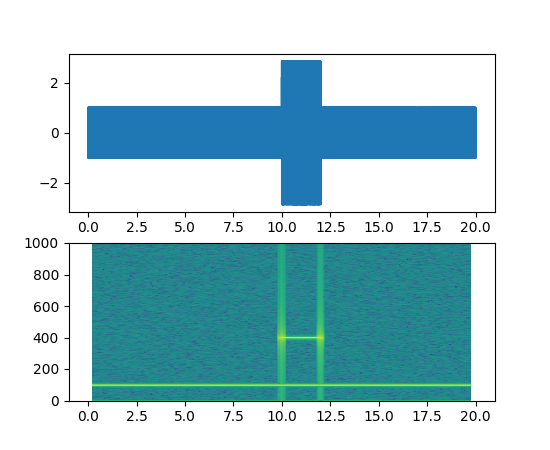`matplotlib.pyplot.``spectral`()

set the default colormap to spectral and apply to current image if any. See help(colormaps) for more information

`matplotlib.pyplot.``spring`()

set the default colormap to spring and apply to current image if any. See help(colormaps) for more information

`matplotlib.pyplot.``spy`(Z, precision=0, marker=None, markersize=None, aspect='equal', **kwargs)

Plot the sparsity pattern on a 2-D array.

`spy(Z)` plots the sparsity pattern of the 2-D array Z.

Parameters: Z : sparse array (n, m) The array to be plotted. precision : float, optional, default: 0 If precision is 0, any non-zero value will be plotted; else, values of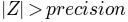will be plotted. For `scipy.sparse.spmatrix` instances, there is a special case: if precision is ‘present’, any value present in the array will be plotted, even if it is identically zero. origin : [“upper”, “lower”], optional, default: “upper” Place the [0,0] index of the array in the upper left or lower left corner of the axes. aspect : [‘auto’ | ‘equal’ | scalar], optional, default: “equal” If ‘equal’, and `extent` is None, changes the axes aspect ratio to match that of the image. If `extent` is not `None`, the axes aspect ratio is changed to match that of the extent. If ‘auto’, changes the image aspect ratio to match that of the axes. If None, default to rc `image.aspect` value. Two plotting styles are available: image or marker. Both are available for full arrays, but only the marker style works for :class:`scipy.sparse.spmatrix` instances. If *marker* and *markersize* are *None*, an image will be returned and any remaining kwargs are passed to :func:`~matplotlib.pyplot.imshow`; else, a :class:`~matplotlib.lines.Line2D` object will be returned with the value of marker determining the marker type, and any remaining kwargs passed to the :meth:`~matplotlib.axes.Axes.plot` method. If *marker* and *markersize* are *None*, useful kwargs include: * *cmap* * *alpha*

`imshow`
for image options.
`plot`
for plotting options
`matplotlib.pyplot.``stackplot`(x, *args, **kwargs)

Draws a stacked area plot.

x : 1d array of dimension N

y : 2d array of dimension MxN, OR any number 1d arrays each of dimension

1xN. The data is assumed to be unstacked. Each of the following calls is legal:

```stackplot(x, y)               # where y is MxN
stackplot(x, y1, y2, y3, y4)  # where y1, y2, y3, y4, are all 1xNm
```

Keyword arguments:

baseline : [‘zero’, ‘sym’, ‘wiggle’, ‘weighted_wiggle’]
Method used to calculate the baseline. ‘zero’ is just a simple stacked plot. ‘sym’ is symmetric around zero and is sometimes called `ThemeRiver`. ‘wiggle’ minimizes the sum of the squared slopes. ‘weighted_wiggle’ does the same but weights to account for size of each layer. It is also called `Streamgraph`-layout. More details can be found at http://leebyron.com/streamgraph/.

labels : A list or tuple of labels to assign to each data series.

colors : A list or tuple of colors. These will be cycled through and
used to colour the stacked areas. All other keyword arguments are passed to `fill_between()`

Returns r : A list of `PolyCollection`, one for each element in the stacked area plot.

`matplotlib.pyplot.``stem`(*args, **kwargs)

Create a stem plot.

Call signatures:

```stem(y, linefmt='b-', markerfmt='bo', basefmt='r-')
stem(x, y, linefmt='b-', markerfmt='bo', basefmt='r-')
```

A stem plot plots vertical lines (using linefmt) at each x location from the baseline to y, and places a marker there using markerfmt. A horizontal line at 0 is is plotted using basefmt.

If no x values are provided, the default is (0, 1, ..., len(y) - 1)

Return value is a tuple (markerline, stemlines, baseline).

This document for details.

Example: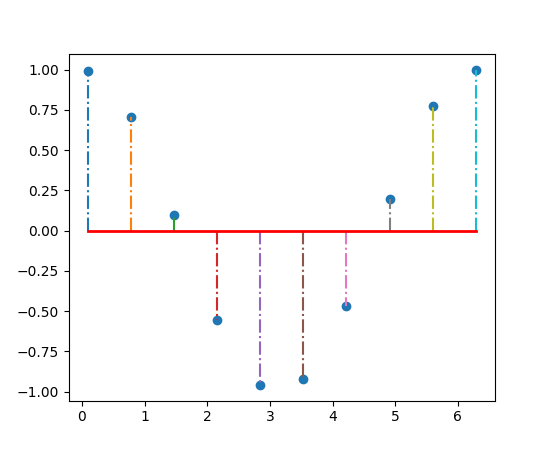Note

In addition to the above described arguments, this function can take a data keyword argument. If such a data argument is given, the following arguments are replaced by data[<arg>]:

• All positional and all keyword arguments.
`matplotlib.pyplot.``step`(x, y, *args, **kwargs)

Make a step plot.

Parameters: Returns: x : array_like 1-D sequence, and it is assumed, but not checked, that it is uniformly increasing. y : array_like 1-D sequence, and it is assumed, but not checked, that it is uniformly increasing. list List of lines that were added. where : [ ‘pre’ | ‘post’ | ‘mid’ ] If ‘pre’ (the default), the interval from x[i] to x[i+1] has level y[i+1]. If ‘post’, that interval has level y[i]. If ‘mid’, the jumps in y occur half-way between the x-values.

Notes

Additional parameters are the same as those for `plot()`.

Note

In addition to the above described arguments, this function can take a data keyword argument. If such a data argument is given, the following arguments are replaced by data[<arg>]:

• All arguments with the following names: ‘x’, ‘y’.
`matplotlib.pyplot.``streamplot`(x, y, u, v, density=1, linewidth=None, color=None, cmap=None, norm=None, arrowsize=1, arrowstyle='-|>', minlength=0.1, transform=None, zorder=None, start_points=None, hold=None, data=None)

Draws streamlines of a vector flow.

x, y : 1d arrays
an evenly spaced grid.
u, v : 2d arrays
x and y-velocities. Number of rows should match length of y, and the number of columns should match x.
density : float or 2-tuple
Controls the closeness of streamlines. When `density = 1`, the domain is divided into a 30x30 grid—density linearly scales this grid. Each cell in the grid can have, at most, one traversing streamline. For different densities in each direction, use [density_x, density_y].
linewidth : numeric or 2d array
vary linewidth when given a 2d array with the same shape as velocities.
color : matplotlib color code, or 2d array
Streamline color. When given an array with the same shape as velocities, color values are converted to colors using cmap.
cmap : `Colormap`
Colormap used to plot streamlines and arrows. Only necessary when using an array input for color.
norm : `Normalize`
Normalize object used to scale luminance data to 0, 1. If None, stretch (min, max) to (0, 1). Only necessary when color is an array.
arrowsize : float
Factor scale arrow size.
arrowstyle : str
Arrow style specification. See `FancyArrowPatch`.
minlength : float
Minimum length of streamline in axes coordinates.
start_points: Nx2 array
Coordinates of starting points for the streamlines. In data coordinates, the same as the `x` and `y` arrays.
zorder : int
any number

Returns:

stream_container : StreamplotSet

Container object with attributes

This container will probably change in the future to allow changes to the colormap, alpha, etc. for both lines and arrows, but these changes should be backward compatible.

`matplotlib.pyplot.``subplot`(*args, **kwargs)

Return a subplot axes positioned by the given grid definition.

Typical call signature:

```subplot(nrows, ncols, plot_number)
```

Where nrows and ncols are used to notionally split the figure into `nrows * ncols` sub-axes, and plot_number is used to identify the particular subplot that this function is to create within the notional grid. plot_number starts at 1, increments across rows first and has a maximum of `nrows * ncols`.

In the case when nrows, ncols and plot_number are all less than 10, a convenience exists, such that the a 3 digit number can be given instead, where the hundreds represent nrows, the tens represent ncols and the units represent plot_number. For instance:

```subplot(211)
```

produces a subaxes in a figure which represents the top plot (i.e. the first) in a 2 row by 1 column notional grid (no grid actually exists, but conceptually this is how the returned subplot has been positioned).

Note

Creating a subplot will delete any pre-existing subplot that overlaps with it beyond sharing a boundary:

```import matplotlib.pyplot as plt
# plot a line, implicitly creating a subplot(111)
plt.plot([1,2,3])
# now create a subplot which represents the top plot of a grid
# with 2 rows and 1 column. Since this subplot will overlap the
# first, the plot (and its axes) previously created, will be removed
plt.subplot(211)
plt.plot(range(12))
plt.subplot(212, facecolor='y') # creates 2nd subplot with yellow background
```

If you do not want this behavior, use the `add_subplot()` method or the `axes()` function instead.

Keyword arguments:

facecolor:
The background color of the subplot, which can be any valid color specifier. See `matplotlib.colors` for more information.
polar:
A boolean flag indicating whether the subplot plot should be a polar projection. Defaults to False.
projection:
A string giving the name of a custom projection to be used for the subplot. This projection must have been previously registered. See `matplotlib.projections`.

`axes()`
For additional information on `axes()` and `subplot()` keyword arguments.
`examples/pie_and_polar_charts/polar_scatter_demo.py`
For an example

Example: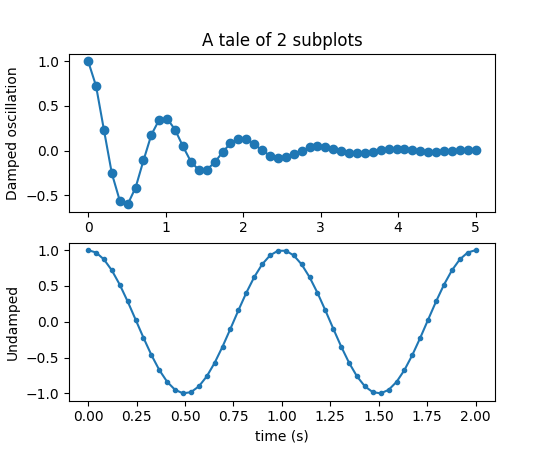`matplotlib.pyplot.``subplot2grid`(shape, loc, rowspan=1, colspan=1, **kwargs)

Create a subplot in a grid. The grid is specified by shape, at location of loc, spanning rowspan, colspan cells in each direction. The index for loc is 0-based.

```subplot2grid(shape, loc, rowspan=1, colspan=1)
```

is identical to

```gridspec=GridSpec(shape, shape)
subplotspec=gridspec.new_subplotspec(loc, rowspan, colspan)
subplot(subplotspec)
```
`matplotlib.pyplot.``subplot_tool`(targetfig=None)

Launch a subplot tool window for a figure.

A `matplotlib.widgets.SubplotTool` instance is returned.

`matplotlib.pyplot.``subplots`(nrows=1, ncols=1, sharex=False, sharey=False, squeeze=True, subplot_kw=None, gridspec_kw=None, **fig_kw)

Create a figure and a set of subplots

This utility wrapper makes it convenient to create common layouts of subplots, including the enclosing figure object, in a single call.

Parameters: nrows, ncols : int, optional, default: 1 Number of rows/columns of the subplot grid. sharex, sharey : bool or {‘none’, ‘all’, ‘row’, ‘col’}, default: False Controls sharing of properties among x (`sharex`) or y (`sharey`) axes: True or ‘all’: x- or y-axis will be shared among all subplots. False or ‘none’: each subplot x- or y-axis will be independent. ‘row’: each subplot row will share an x- or y-axis. ‘col’: each subplot column will share an x- or y-axis. When subplots have a shared x-axis along a column, only the x tick labels of the bottom subplot are visible. Similarly, when subplots have a shared y-axis along a row, only the y tick labels of the first column subplot are visible. squeeze : bool, optional, default: True If True, extra dimensions are squeezed out from the returned Axes object: if only one subplot is constructed (nrows=ncols=1), the resulting single Axes object is returned as a scalar. for Nx1 or 1xN subplots, the returned object is a 1D numpy object array of Axes objects are returned as numpy 1D arrays. for NxM, subplots with N>1 and M>1 are returned as a 2D arrays. If False, no squeezing at all is done: the returned Axes object is always a 2D array containing Axes instances, even if it ends up being 1x1. subplot_kw : dict, optional Dict with keywords passed to the `add_subplot()` call used to create each subplot. gridspec_kw : dict, optional Dict with keywords passed to the `GridSpec` constructor used to create the grid the subplots are placed on. **fig_kw : All additional keyword arguments are passed to the `figure()` call. fig : `matplotlib.figure.Figure` object ax : Axes object or array of Axes objects. ax can be either a single `matplotlib.axes.Axes` object or an array of Axes objects if more than one subplot was created. The dimensions of the resulting array can be controlled with the squeeze keyword, see above.

Examples

First create some toy data:

```>>> x = np.linspace(0, 2*np.pi, 400)
>>> y = np.sin(x**2)
```

Creates just a figure and only one subplot

```>>> fig, ax = plt.subplots()
>>> ax.plot(x, y)
>>> ax.set_title('Simple plot')
```

Creates two subplots and unpacks the output array immediately

```>>> f, (ax1, ax2) = plt.subplots(1, 2, sharey=True)
>>> ax1.plot(x, y)
>>> ax1.set_title('Sharing Y axis')
>>> ax2.scatter(x, y)
```

Creates four polar axes, and accesses them through the returned array

```>>> fig, axes = plt.subplots(2, 2, subplot_kw=dict(polar=True))
>>> axes[0, 0].plot(x, y)
>>> axes[1, 1].scatter(x, y)
```

Share a X axis with each column of subplots

```>>> plt.subplots(2, 2, sharex='col')
```

Share a Y axis with each row of subplots

```>>> plt.subplots(2, 2, sharey='row')
```

Share both X and Y axes with all subplots

```>>> plt.subplots(2, 2, sharex='all', sharey='all')
```

Note that this is the same as

```>>> plt.subplots(2, 2, sharex=True, sharey=True)
```
`matplotlib.pyplot.``subplots_adjust`(*args, **kwargs)

Tune the subplot layout.

call signature:

```subplots_adjust(left=None, bottom=None, right=None, top=None,
wspace=None, hspace=None)
```

The parameter meanings (and suggested defaults) are:

```left  = 0.125  # the left side of the subplots of the figure
right = 0.9    # the right side of the subplots of the figure
bottom = 0.1   # the bottom of the subplots of the figure
top = 0.9      # the top of the subplots of the figure
wspace = 0.2   # the amount of width reserved for blank space between subplots,
# expressed as a fraction of the average axis width
hspace = 0.2   # the amount of height reserved for white space between subplots,
# expressed as a fraction of the average axis height
```

The actual defaults are controlled by the rc file

`matplotlib.pyplot.``summer`()

set the default colormap to summer and apply to current image if any. See help(colormaps) for more information

`matplotlib.pyplot.``suptitle`(*args, **kwargs)

Add a centered title to the figure.

kwargs are `matplotlib.text.Text` properties. Using figure coordinates, the defaults are:

x : 0.5
The x location of the text in figure coords
y : 0.98
The y location of the text in figure coords
horizontalalignment : ‘center’
The horizontal alignment of the text
verticalalignment : ‘top’
The vertical alignment of the text

If the `fontproperties` keyword argument is given then the rcParams defaults for `fontsize` (`figure.titlesize`) and `fontweight` (`figure.titleweight`) will be ignored in favour of the `FontProperties` defaults.

A `matplotlib.text.Text` instance is returned.

Example:

```fig.suptitle('this is the figure title', fontsize=12)
```
`matplotlib.pyplot.``switch_backend`(newbackend)

Switch the default backend. This feature is experimental, and is only expected to work switching to an image backend. e.g., if you have a bunch of PostScript scripts that you want to run from an interactive ipython session, you may want to switch to the PS backend before running them to avoid having a bunch of GUI windows popup. If you try to interactively switch from one GUI backend to another, you will explode.

Calling this command will close all open windows.

`matplotlib.pyplot.``table`(**kwargs)

Add a table to the current axes.

Call signature:

```table(cellText=None, cellColours=None,
cellLoc='right', colWidths=None,
rowLabels=None, rowColours=None, rowLoc='left',
colLabels=None, colColours=None, colLoc='center',
loc='bottom', bbox=None):
```

Returns a `matplotlib.table.Table` instance. Either `cellText` or `cellColours` must be provided. For finer grained control over tables, use the `Table` class and add it to the axes with `add_table()`.

Thanks to John Gill for providing the class and table.

kwargs control the `Table` properties:

Property Description
`agg_filter` unknown
`alpha` float (0.0 transparent through 1.0 opaque)
`animated` [True | False]
`axes` an `Axes` instance
`clip_box` a `matplotlib.transforms.Bbox` instance
`clip_on` [True | False]
`clip_path` [ (`Path`, `Transform`) | `Patch` | None ]
`contains` a callable function
`figure` a `matplotlib.figure.Figure` instance
`fontsize` a float in points
`gid` an id string
`label` string or anything printable with ‘%s’ conversion.
`path_effects` unknown
`picker` [None|float|boolean|callable]
`rasterized` [True | False | None]
`sketch_params` unknown
`snap` unknown
`transform` `Transform` instance
`url` a url string
`visible` [True | False]
`zorder` any number
`matplotlib.pyplot.``text`(x, y, s, fontdict=None, withdash=False, **kwargs)

Add text to the axes.

Add text in string `s` to axis at location `x`, `y`, data coordinates.

Parameters: Other Parameters: x, y : scalars data coordinates s : string text fontdict : dictionary, optional, default: None A dictionary to override the default text properties. If fontdict is None, the defaults are determined by your rc parameters. withdash : boolean, optional, default: False Creates a `TextWithDash` instance instead of a `Text` instance. kwargs : `Text` properties. Other miscellaneous text parameters.

Examples

Individual keyword arguments can be used to override any given parameter:

```>>> text(x, y, s, fontsize=12)
```

The default transform specifies that text is in data coords, alternatively, you can specify text in axis coords (0,0 is lower-left and 1,1 is upper-right). The example below places text in the center of the axes:

```>>> text(0.5, 0.5,'matplotlib', horizontalalignment='center',
...      verticalalignment='center',
...      transform=ax.transAxes)
```

You can put a rectangular box around the text instance (e.g., to set a background color) by using the keyword `bbox`. `bbox` is a dictionary of `Rectangle` properties. For example:

```>>> text(x, y, s, bbox=dict(facecolor='red', alpha=0.5))
```
`matplotlib.pyplot.``thetagrids`(*args, **kwargs)

Get or set the theta locations of the gridlines in a polar plot.

If no arguments are passed, return a tuple (lines, labels) where lines is an array of radial gridlines (`Line2D` instances) and labels is an array of tick labels (`Text` instances):

```lines, labels = thetagrids()
```

Otherwise the syntax is:

```lines, labels = thetagrids(angles, labels=None, fmt='%d', frac = 1.1)
```

set the angles at which to place the theta grids (these gridlines are equal along the theta dimension).

angles is in degrees.

labels, if not None, is a len(angles) list of strings of the labels to use at each angle.

If labels is None, the labels will be `fmt%angle`.

frac is the fraction of the polar axes radius at which to place the label (1 is the edge). e.g., 1.05 is outside the axes and 0.95 is inside the axes.

Return value is a list of tuples (lines, labels):

Note that on input, the labels argument is a list of strings, and on output it is a list of `Text` instances.

Examples:

```# set the locations of the radial gridlines and labels
lines, labels = thetagrids( range(45,360,90) )

# set the locations and labels of the radial gridlines and labels
lines, labels = thetagrids( range(45,360,90), ('NE', 'NW', 'SW','SE') )
```
`matplotlib.pyplot.``tick_params`(axis='both', **kwargs)

Change the appearance of ticks and tick labels.

Parameters: Other Parameters: axis : {‘x’, ‘y’, ‘both’}, optional Which axis to apply the parameters to. axis : {‘x’, ‘y’, ‘both’} Axis on which to operate; default is ‘both’. reset : bool If True, set all parameters to defaults before processing other keyword arguments. Default is False. which : {‘major’, ‘minor’, ‘both’} Default is ‘major’; apply arguments to which ticks. direction : {‘in’, ‘out’, ‘inout’} Puts ticks inside the axes, outside the axes, or both. length : float Tick length in points. width : float Tick width in points. color : color Tick color; accepts any mpl color spec. pad : float Distance in points between tick and label. labelsize : float or str Tick label font size in points or as a string (e.g., ‘large’). labelcolor : color Tick label color; mpl color spec. colors : color Changes the tick color and the label color to the same value: mpl color spec. zorder : float Tick and label zorder. bottom, top, left, right : bool or {‘on’, ‘off’} controls whether to draw the respective ticks. labelbottom, labeltop, labelleft, labelright : bool or {‘on’, ‘off’} controls whether to draw the respective tick labels.

Examples

Usage

```ax.tick_params(direction='out', length=6, width=2, colors='r')
```

This will make all major ticks be red, pointing out of the box, and with dimensions 6 points by 2 points. Tick labels will also be red.

`matplotlib.pyplot.``ticklabel_format`(**kwargs)

Change the `ScalarFormatter` used by default for linear axes.

Optional keyword arguments:

Keyword Description
style [ ‘sci’ (or ‘scientific’) | ‘plain’ ] plain turns off scientific notation
scilimits (m, n), pair of integers; if style is ‘sci’, scientific notation will be used for numbers outside the range 10`m`:sup: to 10`n`:sup:. Use (0,0) to include all numbers.
useOffset [True | False | offset]; if True, the offset will be calculated as needed; if False, no offset will be used; if a numeric offset is specified, it will be used.
axis [ ‘x’ | ‘y’ | ‘both’ ]
useLocale If True, format the number according to the current locale. This affects things such as the character used for the decimal separator. If False, use C-style (English) formatting. The default setting is controlled by the axes.formatter.use_locale rcparam.

Only the major ticks are affected. If the method is called when the `ScalarFormatter` is not the `Formatter` being used, an `AttributeError` will be raised.

`matplotlib.pyplot.``tight_layout`(pad=1.08, h_pad=None, w_pad=None, rect=None)

Automatically adjust subplot parameters to give specified padding.

Parameters:

padding between the figure edge and the edges of subplots, as a fraction of the font-size.
padding (height/width) between edges of adjacent subplots. Defaults to `pad_inches`.
rect : if rect is given, it is interpreted as a rectangle
(left, bottom, right, top) in the normalized figure coordinate that the whole subplots area (including labels) will fit into. Default is (0, 0, 1, 1).
`matplotlib.pyplot.``title`(s, *args, **kwargs)

Set a title of the current axes.

Set one of the three available axes titles. The available titles are positioned above the axes in the center, flush with the left edge, and flush with the right edge.

See `text()` for adding text to the current axes

Parameters: Returns: label : str Text to use for the title fontdict : dict A dictionary controlling the appearance of the title text, the default `fontdict` is: {‘fontsize’: rcParams[‘axes.titlesize’], ‘fontweight’ : rcParams[‘axes.titleweight’], ‘verticalalignment’: ‘baseline’, ‘horizontalalignment’: loc} loc : {‘center’, ‘left’, ‘right’}, str, optional Which title to set, defaults to ‘center’ text : `Text` The matplotlib text instance representing the title kwargs : text properties Other keyword arguments are text properties, see `Text` for a list of valid text properties.
`matplotlib.pyplot.``tricontour`(*args, **kwargs)

Draw contours on an unstructured triangular grid. `tricontour()` and `tricontourf()` draw contour lines and filled contours, respectively. Except as noted, function signatures and return values are the same for both versions.

The triangulation can be specified in one of two ways; either:

```tricontour(triangulation, ...)
```

where triangulation is a `matplotlib.tri.Triangulation` object, or

```tricontour(x, y, ...)
tricontour(x, y, triangles, ...)
tricontour(x, y, triangles=triangles, ...)
```

in which case a Triangulation object will be created. See `Triangulation` for a explanation of these possibilities.

The remaining arguments may be:

```tricontour(..., Z)
```

where Z is the array of values to contour, one per point in the triangulation. The level values are chosen automatically.

```tricontour(..., Z, N)
```

contour N automatically-chosen levels.

```tricontour(..., Z, V)
```

draw contour lines at the values specified in sequence V, which must be in increasing order.

```tricontourf(..., Z, V)
```

fill the (len(V)-1) regions between the values in V, which must be in increasing order.

```tricontour(Z, **kwargs)
```

Use keyword args to control colors, linewidth, origin, cmap ... see below for more details.

`C = tricontour(...)` returns a `TriContourSet` object.

Optional keyword arguments:

colors: [ None | string | (mpl_colors) ]

If None, the colormap specified by cmap will be used.

If a string, like ‘r’ or ‘red’, all levels will be plotted in this color.

If a tuple of matplotlib color args (string, float, rgb, etc), different levels will be plotted in different colors in the order specified.

alpha: float
The alpha blending value
cmap: [ None | Colormap ]
A cm `Colormap` instance or None. If cmap is None and colors is None, a default Colormap is used.
norm: [ None | Normalize ]
A `matplotlib.colors.Normalize` instance for scaling data values to colors. If norm is None and colors is None, the default linear scaling is used.
levels [level0, level1, ..., leveln]
A list of floating point numbers indicating the level curves to draw, in increasing order; e.g., to draw just the zero contour pass `levels=`
origin: [ None | ‘upper’ | ‘lower’ | ‘image’ ]

If None, the first value of Z will correspond to the lower left corner, location (0,0). If ‘image’, the rc value for `image.origin` will be used.

This keyword is not active if X and Y are specified in the call to contour.

extent: [ None | (x0,x1,y0,y1) ]

If origin is not None, then extent is interpreted as in `matplotlib.pyplot.imshow()`: it gives the outer pixel boundaries. In this case, the position of Z[0,0] is the center of the pixel, not a corner. If origin is None, then (x0, y0) is the position of Z[0,0], and (x1, y1) is the position of Z[-1,-1].

This keyword is not active if X and Y are specified in the call to contour.

locator: [ None | ticker.Locator subclass ]
If locator is None, the default `MaxNLocator` is used. The locator is used to determine the contour levels if they are not given explicitly via the V argument.
extend: [ ‘neither’ | ‘both’ | ‘min’ | ‘max’ ]
Unless this is ‘neither’, contour levels are automatically added to one or both ends of the range so that all data are included. These added ranges are then mapped to the special colormap values which default to the ends of the colormap range, but can be set via `matplotlib.colors.Colormap.set_under()` and `matplotlib.colors.Colormap.set_over()` methods.
xunits, yunits: [ None | registered units ]
Override axis units by specifying an instance of a `matplotlib.units.ConversionInterface`.

tricontour-only keyword arguments:

linewidths: [ None | number | tuple of numbers ]

If linewidths is None, the default width in `lines.linewidth` in `matplotlibrc` is used.

If a number, all levels will be plotted with this linewidth.

If a tuple, different levels will be plotted with different linewidths in the order specified

linestyles: [ None | ‘solid’ | ‘dashed’ | ‘dashdot’ | ‘dotted’ ]

If linestyles is None, the ‘solid’ is used.

linestyles can also be an iterable of the above strings specifying a set of linestyles to be used. If this iterable is shorter than the number of contour levels it will be repeated as necessary.

If contour is using a monochrome colormap and the contour level is less than 0, then the linestyle specified in `contour.negative_linestyle` in `matplotlibrc` will be used.

tricontourf-only keyword arguments:

antialiased: [ True | False ]
enable antialiasing

Note: tricontourf fills intervals that are closed at the top; that is, for boundaries z1 and z2, the filled region is:

```z1 < z <= z2
```

There is one exception: if the lowest boundary coincides with the minimum value of the z array, then that minimum value will be included in the lowest interval.

Examples: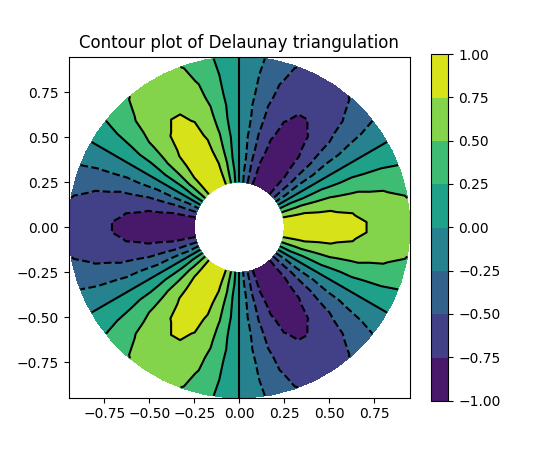(png, pdf)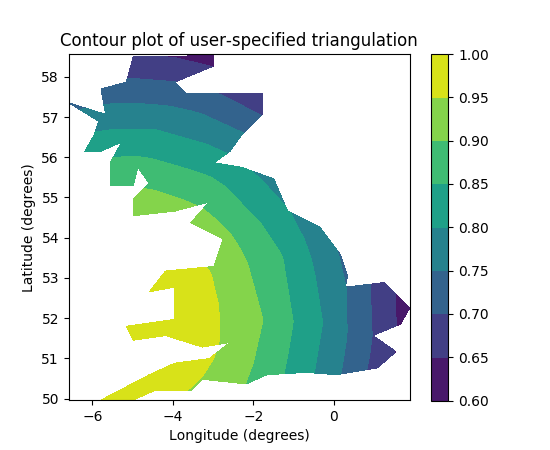(png, pdf)

`matplotlib.pyplot.``tricontourf`(*args, **kwargs)

Draw contours on an unstructured triangular grid. `tricontour()` and `tricontourf()` draw contour lines and filled contours, respectively. Except as noted, function signatures and return values are the same for both versions.

The triangulation can be specified in one of two ways; either:

```tricontour(triangulation, ...)
```

where triangulation is a `matplotlib.tri.Triangulation` object, or

```tricontour(x, y, ...)
tricontour(x, y, triangles, ...)
tricontour(x, y, triangles=triangles, ...)
```

in which case a Triangulation object will be created. See `Triangulation` for a explanation of these possibilities.

The remaining arguments may be:

```tricontour(..., Z)
```

where Z is the array of values to contour, one per point in the triangulation. The level values are chosen automatically.

```tricontour(..., Z, N)
```

contour N automatically-chosen levels.

```tricontour(..., Z, V)
```

draw contour lines at the values specified in sequence V, which must be in increasing order.

```tricontourf(..., Z, V)
```

fill the (len(V)-1) regions between the values in V, which must be in increasing order.

```tricontour(Z, **kwargs)
```

Use keyword args to control colors, linewidth, origin, cmap ... see below for more details.

`C = tricontour(...)` returns a `TriContourSet` object.

Optional keyword arguments:

colors: [ None | string | (mpl_colors) ]

If None, the colormap specified by cmap will be used.

If a string, like ‘r’ or ‘red’, all levels will be plotted in this color.

If a tuple of matplotlib color args (string, float, rgb, etc), different levels will be plotted in different colors in the order specified.

alpha: float
The alpha blending value
cmap: [ None | Colormap ]
A cm `Colormap` instance or None. If cmap is None and colors is None, a default Colormap is used.
norm: [ None | Normalize ]
A `matplotlib.colors.Normalize` instance for scaling data values to colors. If norm is None and colors is None, the default linear scaling is used.
levels [level0, level1, ..., leveln]
A list of floating point numbers indicating the level curves to draw, in increasing order; e.g., to draw just the zero contour pass `levels=`
origin: [ None | ‘upper’ | ‘lower’ | ‘image’ ]

If None, the first value of Z will correspond to the lower left corner, location (0,0). If ‘image’, the rc value for `image.origin` will be used.

This keyword is not active if X and Y are specified in the call to contour.

extent: [ None | (x0,x1,y0,y1) ]

If origin is not None, then extent is interpreted as in `matplotlib.pyplot.imshow()`: it gives the outer pixel boundaries. In this case, the position of Z[0,0] is the center of the pixel, not a corner. If origin is None, then (x0, y0) is the position of Z[0,0], and (x1, y1) is the position of Z[-1,-1].

This keyword is not active if X and Y are specified in the call to contour.

locator: [ None | ticker.Locator subclass ]
If locator is None, the default `MaxNLocator` is used. The locator is used to determine the contour levels if they are not given explicitly via the V argument.
extend: [ ‘neither’ | ‘both’ | ‘min’ | ‘max’ ]
Unless this is ‘neither’, contour levels are automatically added to one or both ends of the range so that all data are included. These added ranges are then mapped to the special colormap values which default to the ends of the colormap range, but can be set via `matplotlib.colors.Colormap.set_under()` and `matplotlib.colors.Colormap.set_over()` methods.
xunits, yunits: [ None | registered units ]
Override axis units by specifying an instance of a `matplotlib.units.ConversionInterface`.

tricontour-only keyword arguments:

linewidths: [ None | number | tuple of numbers ]

If linewidths is None, the default width in `lines.linewidth` in `matplotlibrc` is used.

If a number, all levels will be plotted with this linewidth.

If a tuple, different levels will be plotted with different linewidths in the order specified

linestyles: [ None | ‘solid’ | ‘dashed’ | ‘dashdot’ | ‘dotted’ ]

If linestyles is None, the ‘solid’ is used.

linestyles can also be an iterable of the above strings specifying a set of linestyles to be used. If this iterable is shorter than the number of contour levels it will be repeated as necessary.

If contour is using a monochrome colormap and the contour level is less than 0, then the linestyle specified in `contour.negative_linestyle` in `matplotlibrc` will be used.

tricontourf-only keyword arguments:

antialiased: [ True | False ]
enable antialiasing

Note: tricontourf fills intervals that are closed at the top; that is, for boundaries z1 and z2, the filled region is:

```z1 < z <= z2
```

There is one exception: if the lowest boundary coincides with the minimum value of the z array, then that minimum value will be included in the lowest interval.

Examples:(png, pdf)(png, pdf)

`matplotlib.pyplot.``tripcolor`(*args, **kwargs)

Create a pseudocolor plot of an unstructured triangular grid.

The triangulation can be specified in one of two ways; either:

```tripcolor(triangulation, ...)
```

where triangulation is a `matplotlib.tri.Triangulation` object, or

```tripcolor(x, y, ...)
tripcolor(x, y, triangles, ...)
tripcolor(x, y, triangles=triangles, ...)
```

in which case a Triangulation object will be created. See `Triangulation` for a explanation of these possibilities.

The next argument must be C, the array of color values, either one per point in the triangulation if color values are defined at points, or one per triangle in the triangulation if color values are defined at triangles. If there are the same number of points and triangles in the triangulation it is assumed that color values are defined at points; to force the use of color values at triangles use the kwarg facecolors*=C instead of just *C.

shading may be ‘flat’ (the default) or ‘gouraud’. If shading is ‘flat’ and C values are defined at points, the color values used for each triangle are from the mean C of the triangle’s three points. If shading is ‘gouraud’ then color values must be defined at points.

The remaining kwargs are the same as for `pcolor()`.

Example: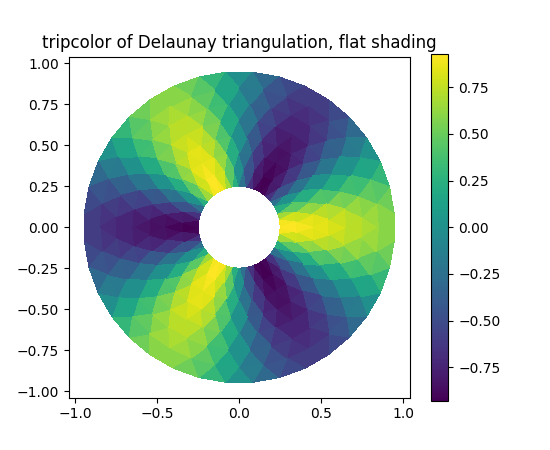(png, pdf)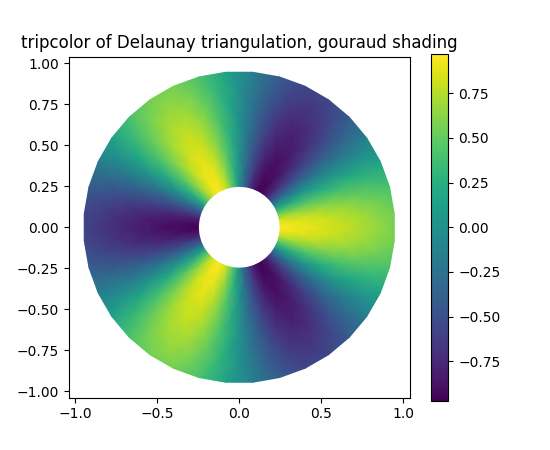(png, pdf)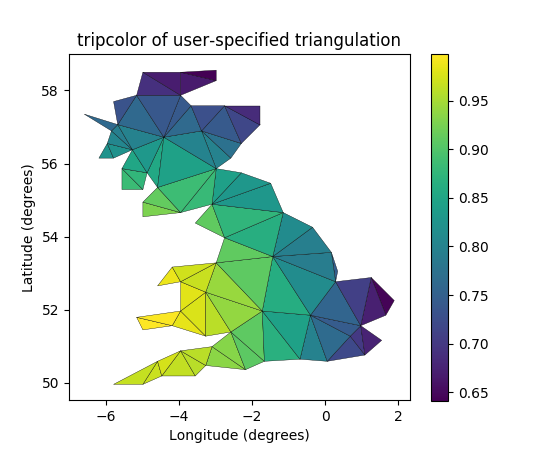(png, pdf)

`matplotlib.pyplot.``triplot`(*args, **kwargs)

Draw a unstructured triangular grid as lines and/or markers.

The triangulation to plot can be specified in one of two ways; either:

```triplot(triangulation, ...)
```

where triangulation is a `matplotlib.tri.Triangulation` object, or

```triplot(x, y, ...)
triplot(x, y, triangles, ...)
triplot(x, y, triangles=triangles, ...)
```

in which case a Triangulation object will be created. See `Triangulation` for a explanation of these possibilities.

The remaining args and kwargs are the same as for `plot()`.

Return a list of 2 `Line2D` containing respectively:

• the lines plotted for triangles edges
• the markers plotted for triangles nodes

Example: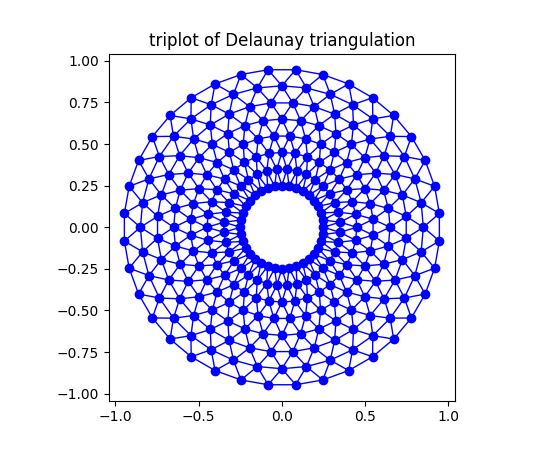(png, pdf)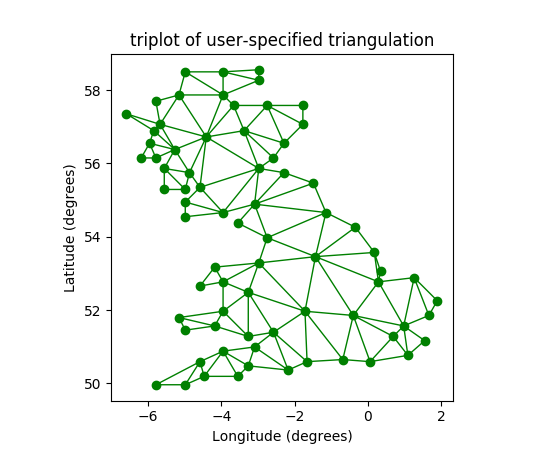(png, pdf)

`matplotlib.pyplot.``twinx`(ax=None)

Make a second axes that shares the x-axis. The new axes will overlay ax (or the current axes if ax is None). The ticks for ax2 will be placed on the right, and the ax2 instance is returned.

`examples/api_examples/two_scales.py`
For an example
`matplotlib.pyplot.``twiny`(ax=None)

Make a second axes that shares the y-axis. The new axis will overlay ax (or the current axes if ax is None). The ticks for ax2 will be placed on the top, and the ax2 instance is returned.

`matplotlib.pyplot.``uninstall_repl_displayhook`()

Uninstalls the matplotlib display hook.

`matplotlib.pyplot.``violinplot`(dataset, positions=None, vert=True, widths=0.5, showmeans=False, showextrema=True, showmedians=False, points=100, bw_method=None, hold=None, data=None)

Make a violin plot.

Make a violin plot for each column of dataset or each vector in sequence dataset. Each filled area extends to represent the entire data range, with optional lines at the mean, the median, the minimum, and the maximum.

Parameters: dataset : Array or a sequence of vectors. The input data. positions : array-like, default = [1, 2, ..., n] Sets the positions of the violins. The ticks and limits are automatically set to match the positions. vert : bool, default = True. If true, creates a vertical violin plot. Otherwise, creates a horizontal violin plot. widths : array-like, default = 0.5 Either a scalar or a vector that sets the maximal width of each violin. The default is 0.5, which uses about half of the available horizontal space. showmeans : bool, default = False If `True`, will toggle rendering of the means. showextrema : bool, default = True If `True`, will toggle rendering of the extrema. showmedians : bool, default = False If `True`, will toggle rendering of the medians. points : scalar, default = 100 Defines the number of points to evaluate each of the gaussian kernel density estimations at. bw_method : str, scalar or callable, optional The method used to calculate the estimator bandwidth. This can be ‘scott’, ‘silverman’, a scalar constant or a callable. If a scalar, this will be used directly as `kde.factor`. If a callable, it should take a `GaussianKDE` instance as its only parameter and return a scalar. If None (default), ‘scott’ is used. result : dict A dictionary mapping each component of the violinplot to a list of the corresponding collection instances created. The dictionary has the following keys: `bodies`: A list of the `matplotlib.collections.PolyCollection` instances containing the filled area of each violin. `cmeans`: A `matplotlib.collections.LineCollection` instance created to identify the mean values of each of the violin’s distribution. `cmins`: A `matplotlib.collections.LineCollection` instance created to identify the bottom of each violin’s distribution. `cmaxes`: A `matplotlib.collections.LineCollection` instance created to identify the top of each violin’s distribution. `cbars`: A `matplotlib.collections.LineCollection` instance created to identify the centers of each violin’s distribution. `cmedians`: A `matplotlib.collections.LineCollection` instance created to identify the median values of each of the violin’s distribution. Note In addition to the above described arguments, this function can take a data keyword argument. If such a data argument is given, the following arguments are replaced by data[]: All arguments with the following names: ‘dataset’.
`matplotlib.pyplot.``viridis`()

set the default colormap to viridis and apply to current image if any. See help(colormaps) for more information

`matplotlib.pyplot.``vlines`(x, ymin, ymax, colors='k', linestyles='solid', label='', hold=None, data=None, **kwargs)

Plot vertical lines.

Plot vertical lines at each `x` from `ymin` to `ymax`.

Parameters: Returns: x : scalar or 1D array_like x-indexes where to plot the lines. ymin, ymax : scalar or 1D array_like Respective beginning and end of each line. If scalars are provided, all lines will have same length. colors : array_like of colors, optional, default: ‘k’ linestyles : [‘solid’ | ‘dashed’ | ‘dashdot’ | ‘dotted’], optional label : string, optional, default: ‘’ lines : `LineCollection` kwargs : `LineCollection` properties.

`hlines`
horizontal lines

ExamplesNote

In addition to the above described arguments, this function can take a data keyword argument. If such a data argument is given, the following arguments are replaced by data[<arg>]:

• All arguments with the following names: ‘colors’, ‘x’, ‘ymax’, ‘ymin’.
`matplotlib.pyplot.``waitforbuttonpress`(*args, **kwargs)

Blocking call to interact with the figure.

This will return True is a key was pressed, False if a mouse button was pressed and None if timeout was reached without either being pressed.

If timeout is negative, does not timeout.

`matplotlib.pyplot.``winter`()

set the default colormap to winter and apply to current image if any. See help(colormaps) for more information

`matplotlib.pyplot.``xcorr`(x, y, normed=True, detrend=<function detrend_none>, usevlines=True, maxlags=10, hold=None, data=None, **kwargs)

Plot the cross correlation between x and y.

The correlation with lag k is defined as sum_n x[n+k] * conj(y[n]).

Parameters: Returns: x : sequence of scalars of length n y : sequence of scalars of length n hold : boolean, optional, deprecated, default: True detrend : callable, optional, default: `mlab.detrend_none` x is detrended by the `detrend` callable. Default is no normalization. normed : boolean, optional, default: True if True, input vectors are normalised to unit length. usevlines : boolean, optional, default: True if True, Axes.vlines is used to plot the vertical lines from the origin to the acorr. Otherwise, Axes.plot is used. maxlags : integer, optional, default: 10 number of lags to show. If None, will return all 2 * len(x) - 1 lags. (lags, c, line, b) : where: `lags` are a length 2`maxlags+1 lag vector. `c` is the 2`maxlags+1 auto correlation vectorI `line` is a `Line2D` instance returned by `plot`. `b` is the x-axis (none, if plot is used). linestyle : `Line2D` prop, optional, default: None Only used if usevlines is False. marker : string, optional, default: ‘o’

Notes

The cross correlation is performed with `numpy.correlate()` with `mode` = 2.

Note

In addition to the above described arguments, this function can take a data keyword argument. If such a data argument is given, the following arguments are replaced by data[<arg>]:

• All arguments with the following names: ‘x’, ‘y’.
`matplotlib.pyplot.``xkcd`(scale=1, length=100, randomness=2)

Turns on xkcd sketch-style drawing mode. This will only have effect on things drawn after this function is called.

For best results, the “Humor Sans” font should be installed: it is not included with matplotlib.

Parameters: scale : float, optional The amplitude of the wiggle perpendicular to the source line. length : float, optional The length of the wiggle along the line. randomness : float, optional The scale factor by which the length is shrunken or expanded.

Notes

This function works by a number of rcParams, so it will probably override others you have set before.

If you want the effects of this function to be temporary, it can be used as a context manager, for example:

```with plt.xkcd():
# This figure will be in XKCD-style
fig1 = plt.figure()
# ...

# This figure will be in regular style
fig2 = plt.figure()
```
`matplotlib.pyplot.``xlabel`(s, *args, **kwargs)

Set the x axis label of the current axis.

Default override is:

```override = {
'fontsize'            : 'small',
'verticalalignment'   : 'top',
'horizontalalignment' : 'center'
}
```

`text()`
For information on how override and the optional args work
`matplotlib.pyplot.``xlim`(*args, **kwargs)

Get or set the x limits of the current axes.

```xmin, xmax = xlim()   # return the current xlim
xlim( (xmin, xmax) )  # set the xlim to xmin, xmax
xlim( xmin, xmax )    # set the xlim to xmin, xmax
```

If you do not specify args, you can pass the xmin and xmax as kwargs, e.g.:

```xlim(xmax=3) # adjust the max leaving min unchanged
xlim(xmin=1) # adjust the min leaving max unchanged
```

Setting limits turns autoscaling off for the x-axis.

The new axis limits are returned as a length 2 tuple.

`matplotlib.pyplot.``xscale`(*args, **kwargs)

Set the scaling of the x-axis.

call signature:

```xscale(scale, **kwargs)
```

The available scales are: ‘linear’ | ‘log’ | ‘logit’ | ‘symlog’

Different keywords may be accepted, depending on the scale:

‘linear’

‘log’

basex/basey:
The base of the logarithm
nonposx/nonposy: [‘mask’ | ‘clip’ ]
non-positive values in x or y can be masked as invalid, or clipped to a very small positive number
subsx/subsy:

Where to place the subticks between each major tick. Should be a sequence of integers. For example, in a log10 scale: `[2, 3, 4, 5, 6, 7, 8, 9]`

will place 8 logarithmically spaced minor ticks between each major tick.

‘logit’

nonpos: [‘mask’ | ‘clip’ ]
values beyond ]0, 1[ can be masked as invalid, or clipped to a number very close to 0 or 1

‘symlog’

basex/basey:
The base of the logarithm
linthreshx/linthreshy:
The range (-x, x) within which the plot is linear (to avoid having the plot go to infinity around zero).
subsx/subsy:

Where to place the subticks between each major tick. Should be a sequence of integers. For example, in a log10 scale: `[2, 3, 4, 5, 6, 7, 8, 9]`

will place 8 logarithmically spaced minor ticks between each major tick.

linscalex/linscaley:
This allows the linear range (-linthresh to linthresh) to be stretched relative to the logarithmic range. Its value is the number of decades to use for each half of the linear range. For example, when linscale == 1.0 (the default), the space used for the positive and negative halves of the linear range will be equal to one decade in the logarithmic range.
`matplotlib.pyplot.``xticks`(*args, **kwargs)

Get or set the x-limits of the current tick locations and labels.